# RD Sharma Solutions for Class 10 Maths Chapter 1 Real Numbers

Mathematics is all about numbers and understanding their types, properties and operations. Having strong knowledge of numbers is very important for further studies in Class 10 Maths. Mathematics textbook by RD Sharma is one of the best books for reference. We at BYJU’S have developed RD Sharma Solutions in order to enhance the learning and understanding of concepts for students.

## Download the PDF of RD Sharma For Class 10 Maths Chapter 1 Real Numbers here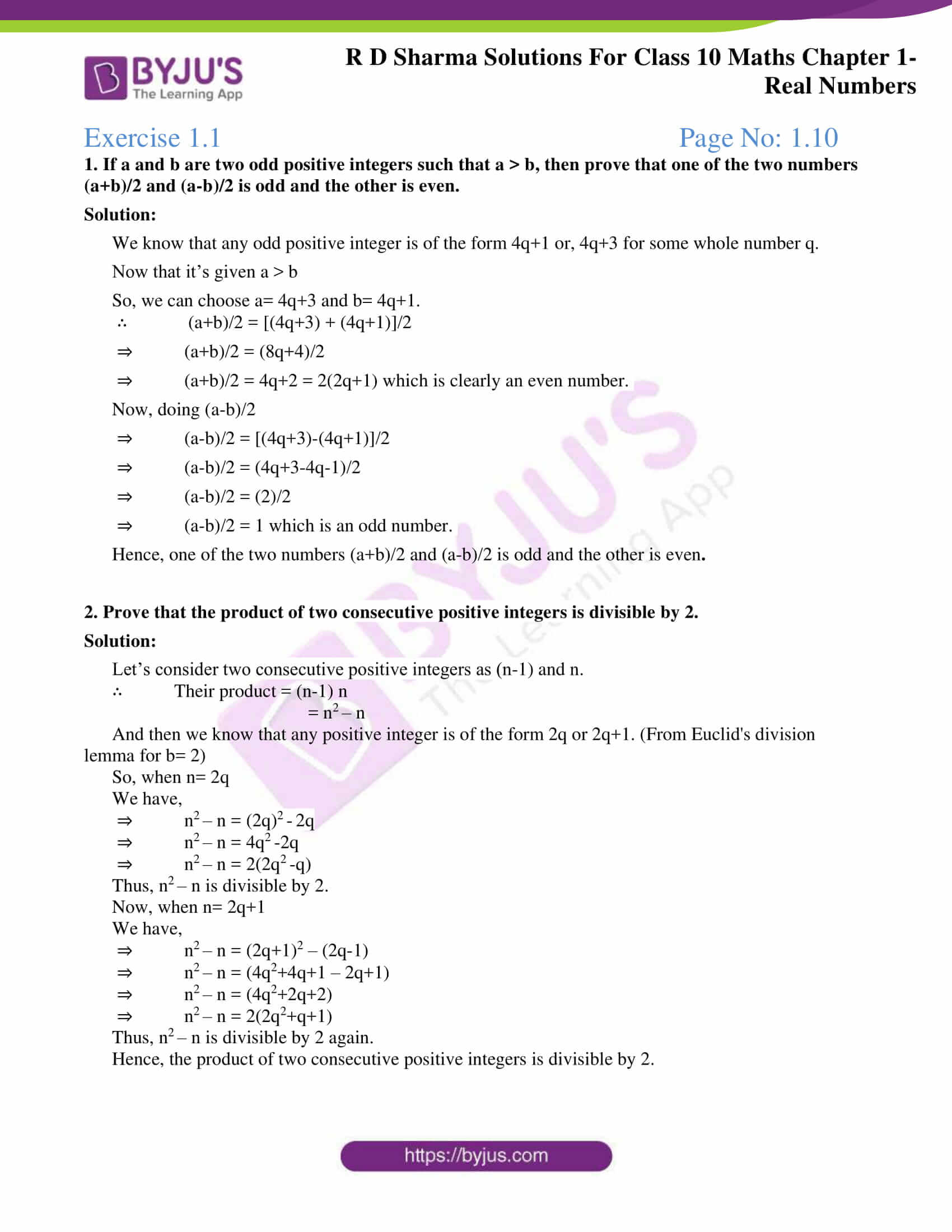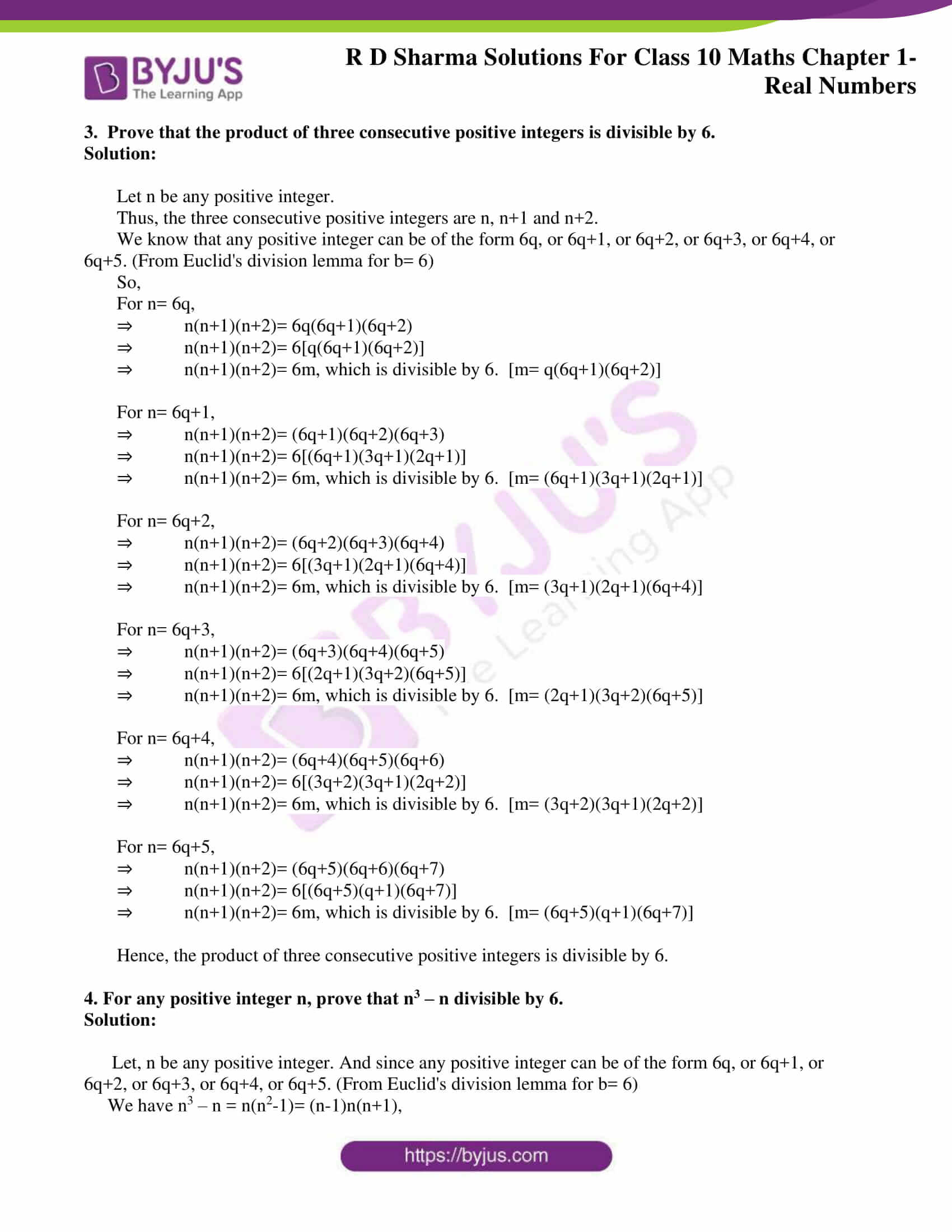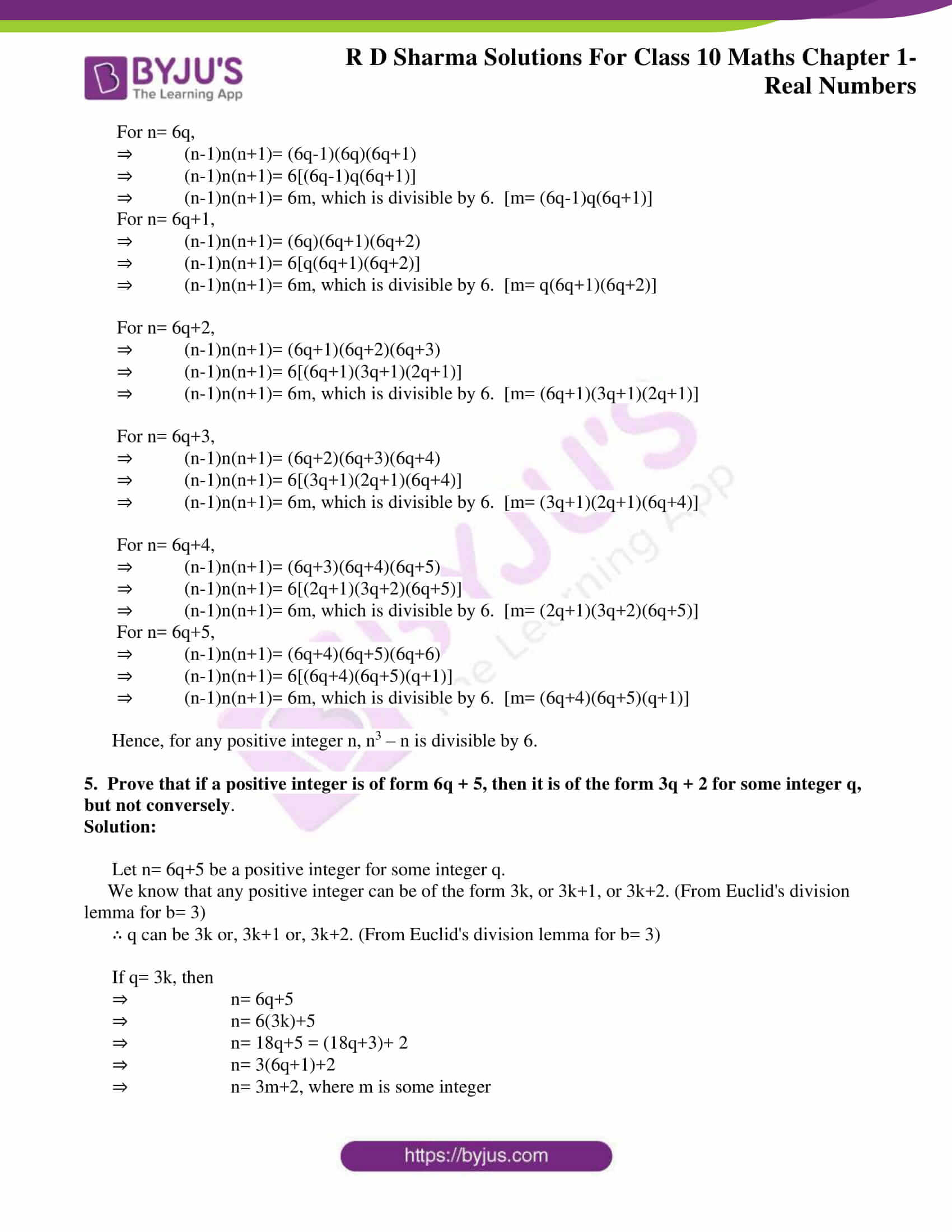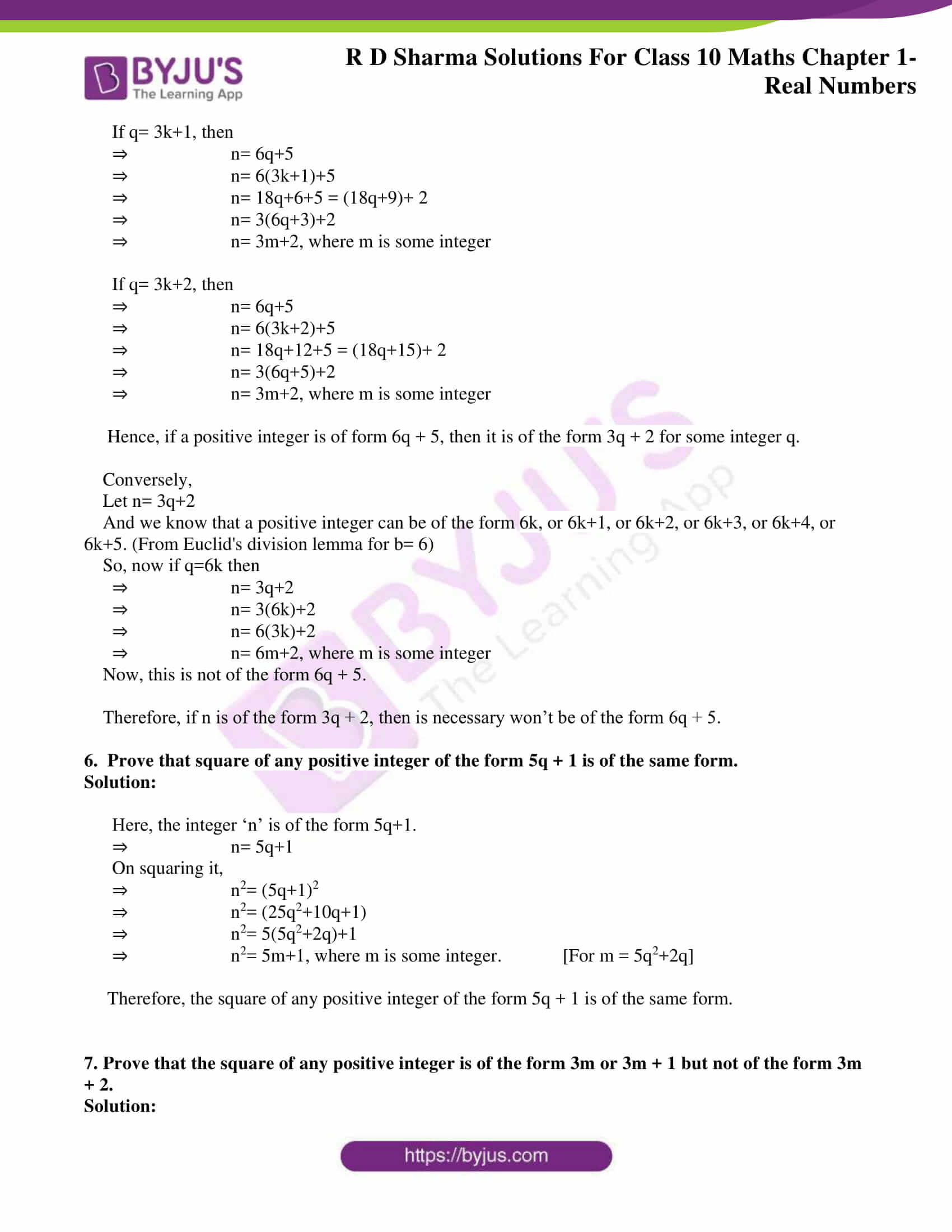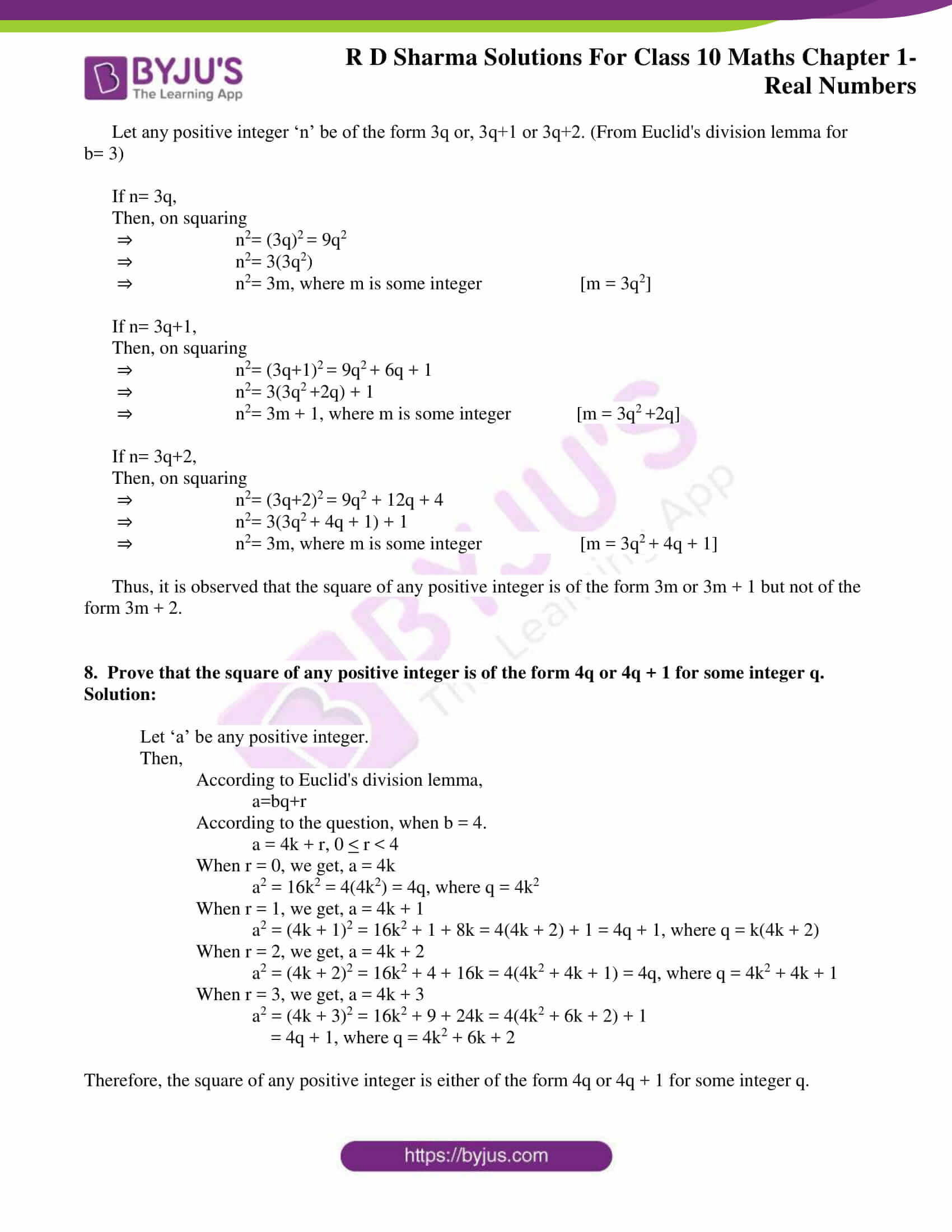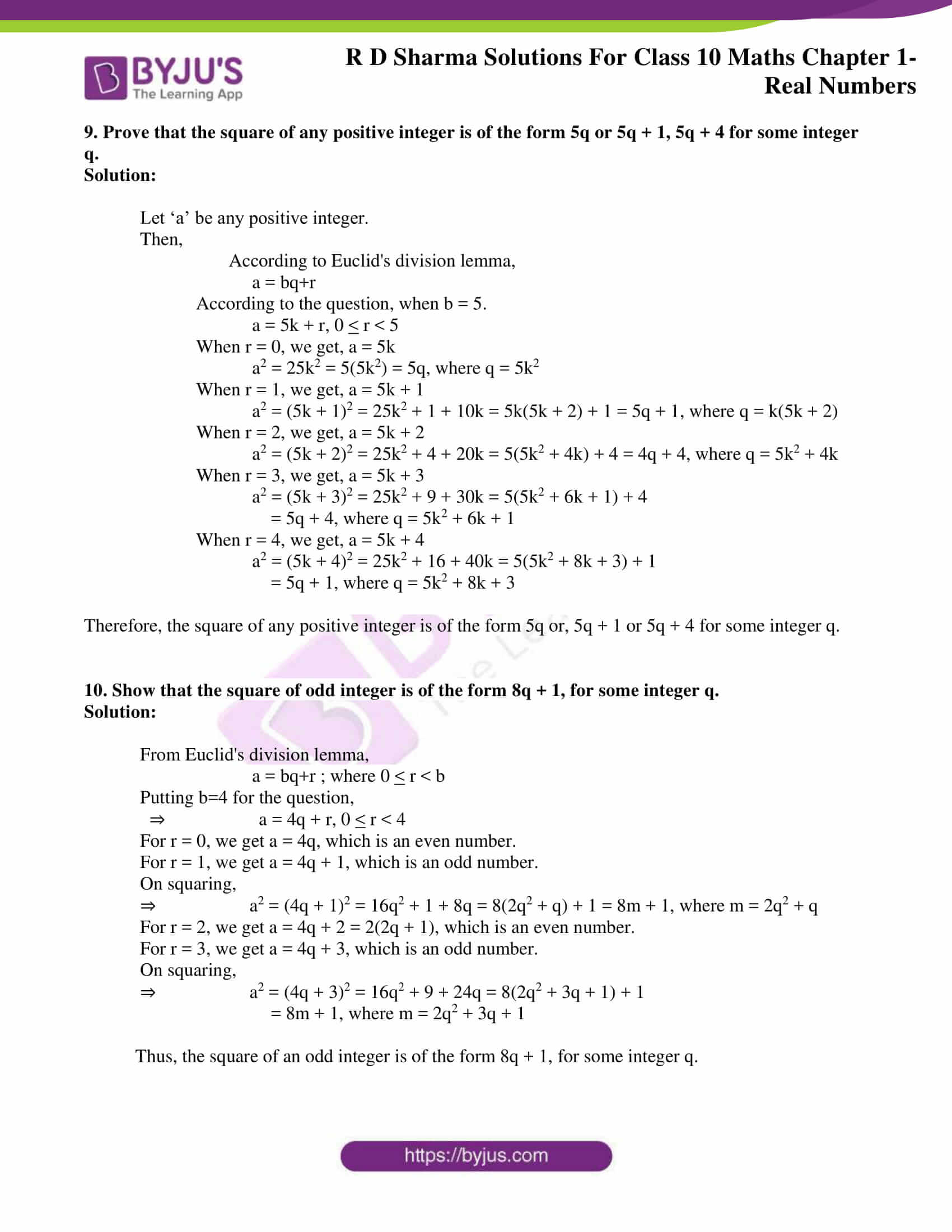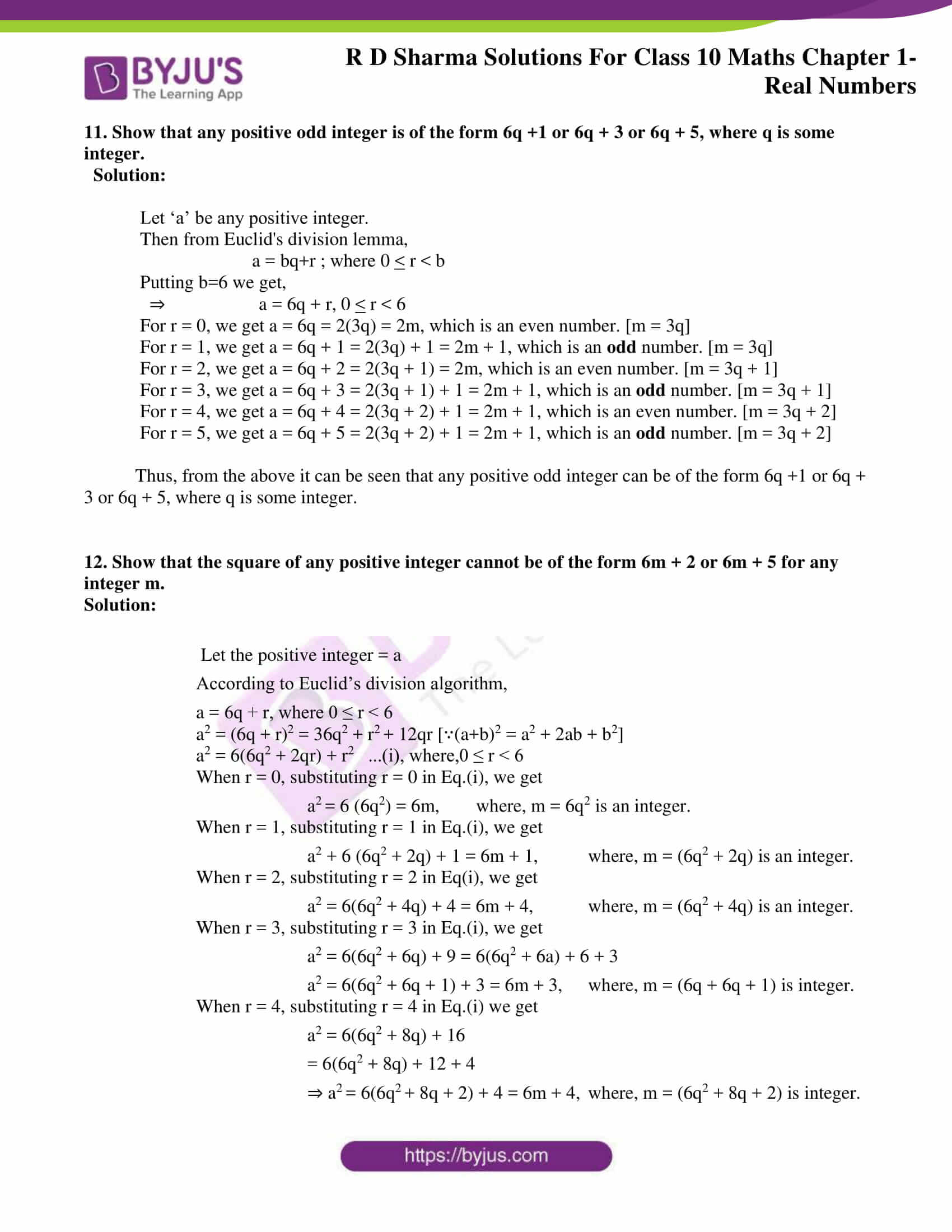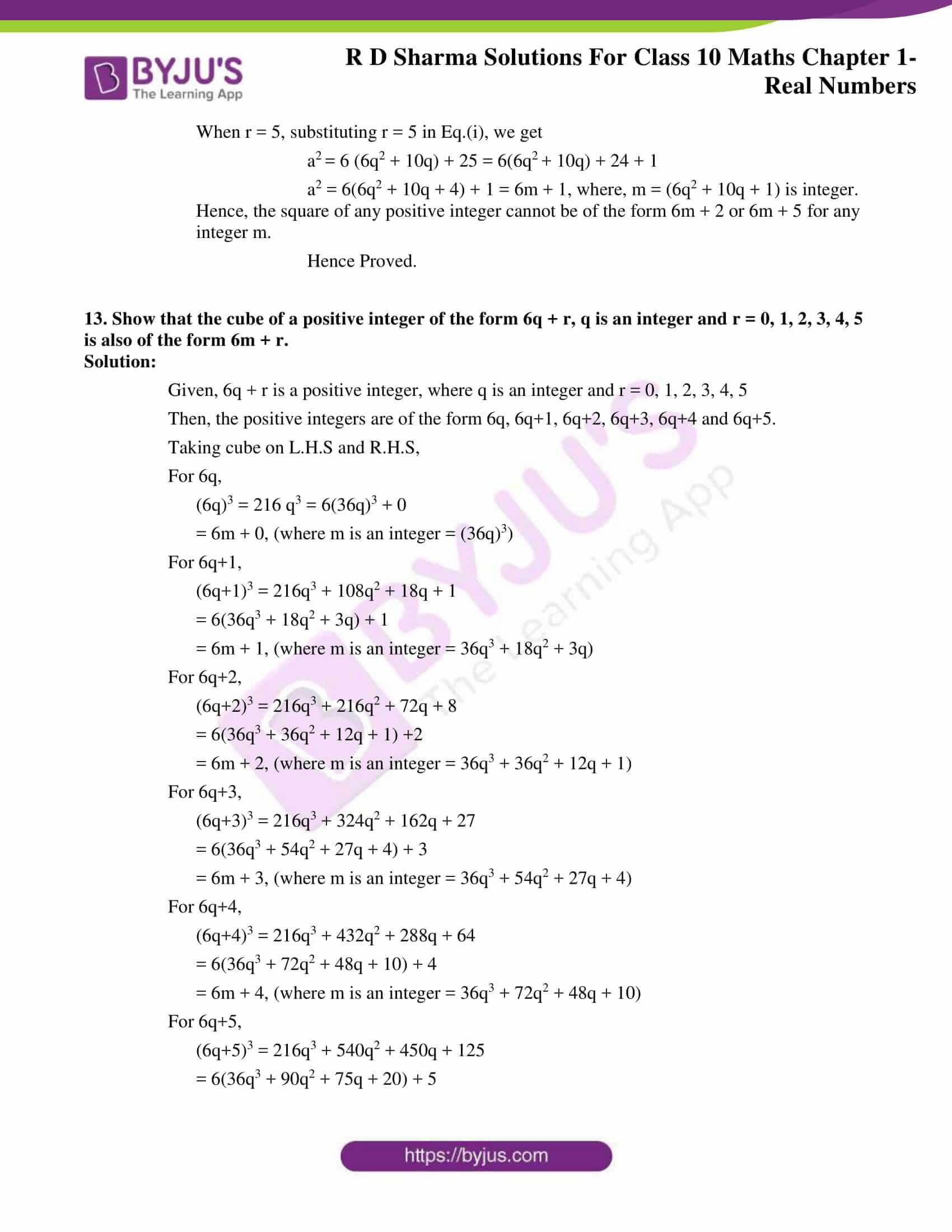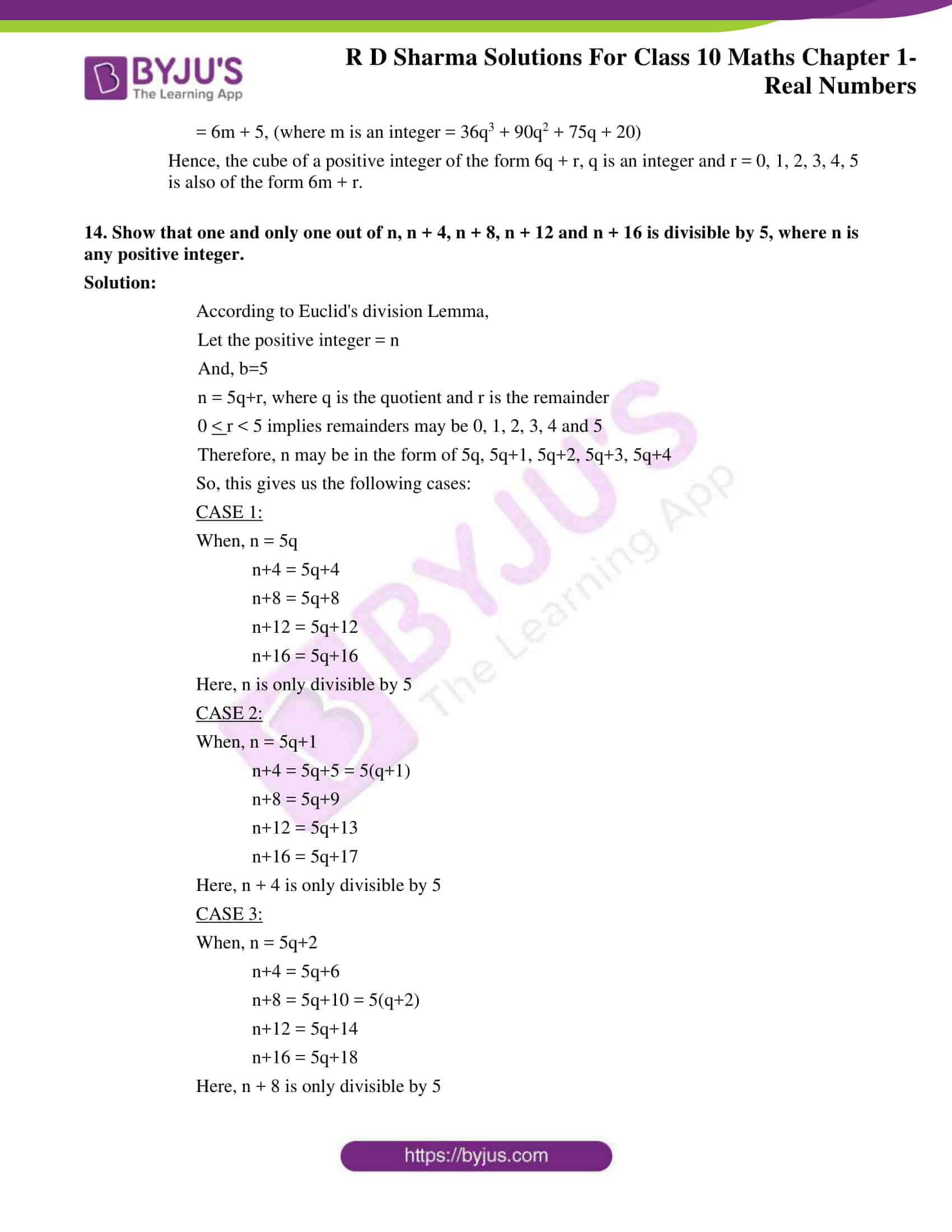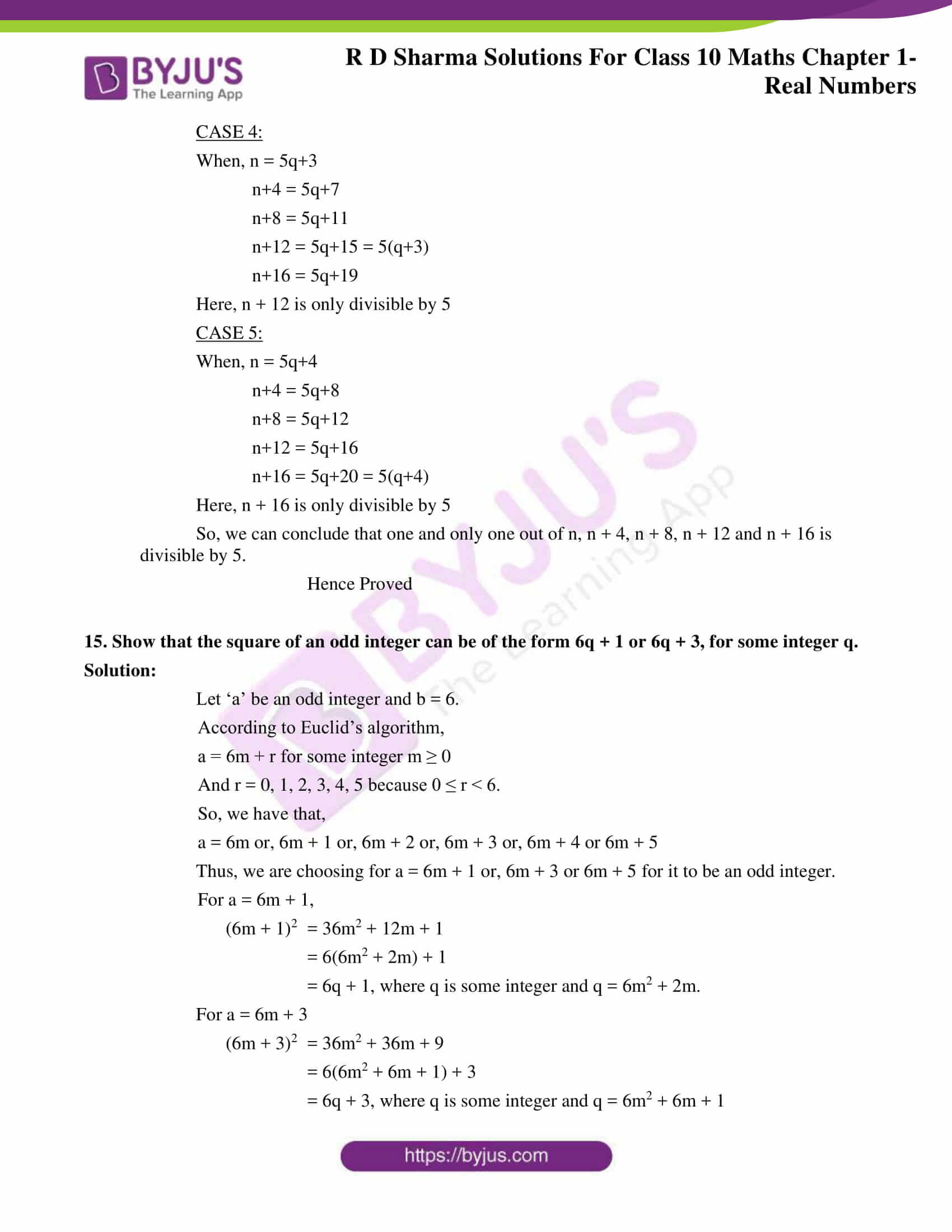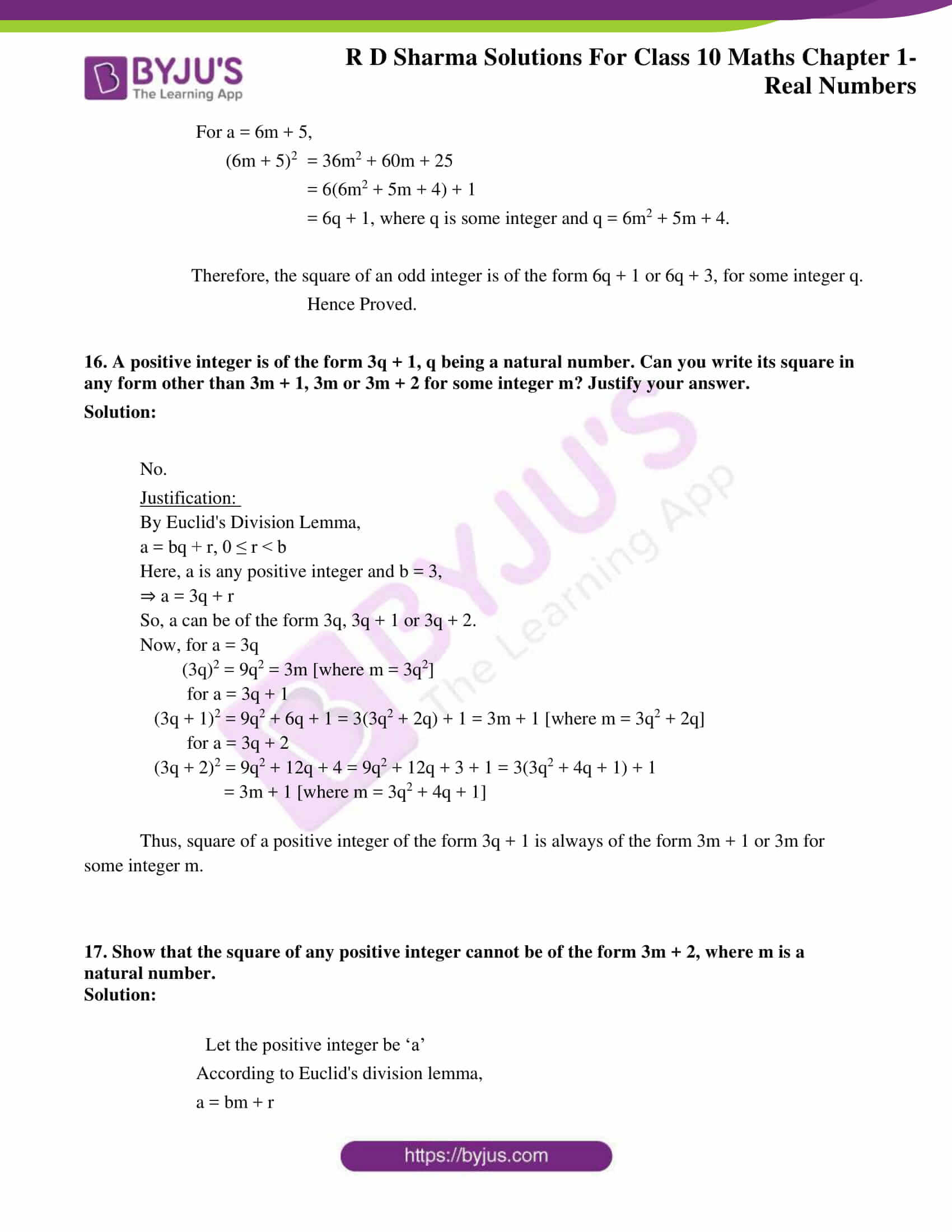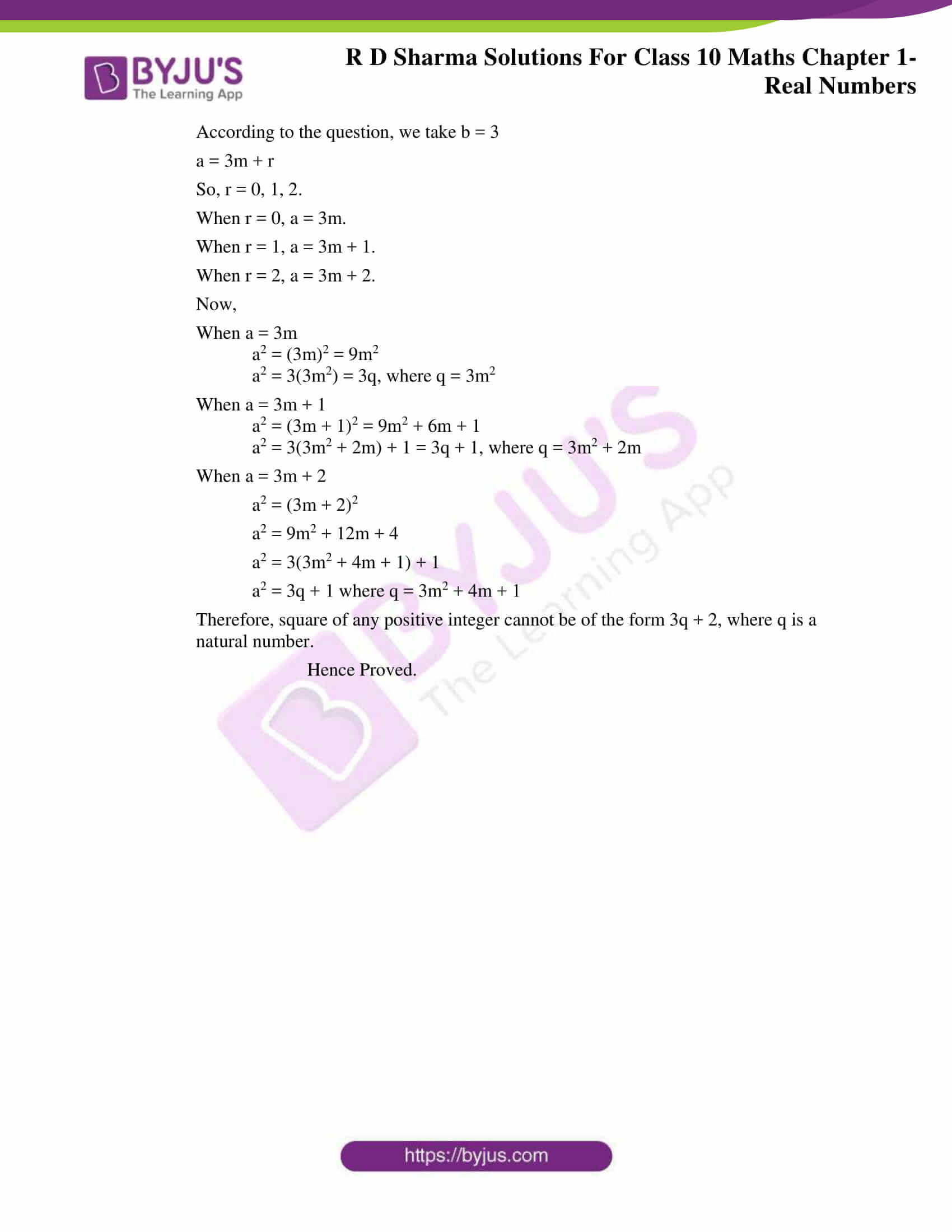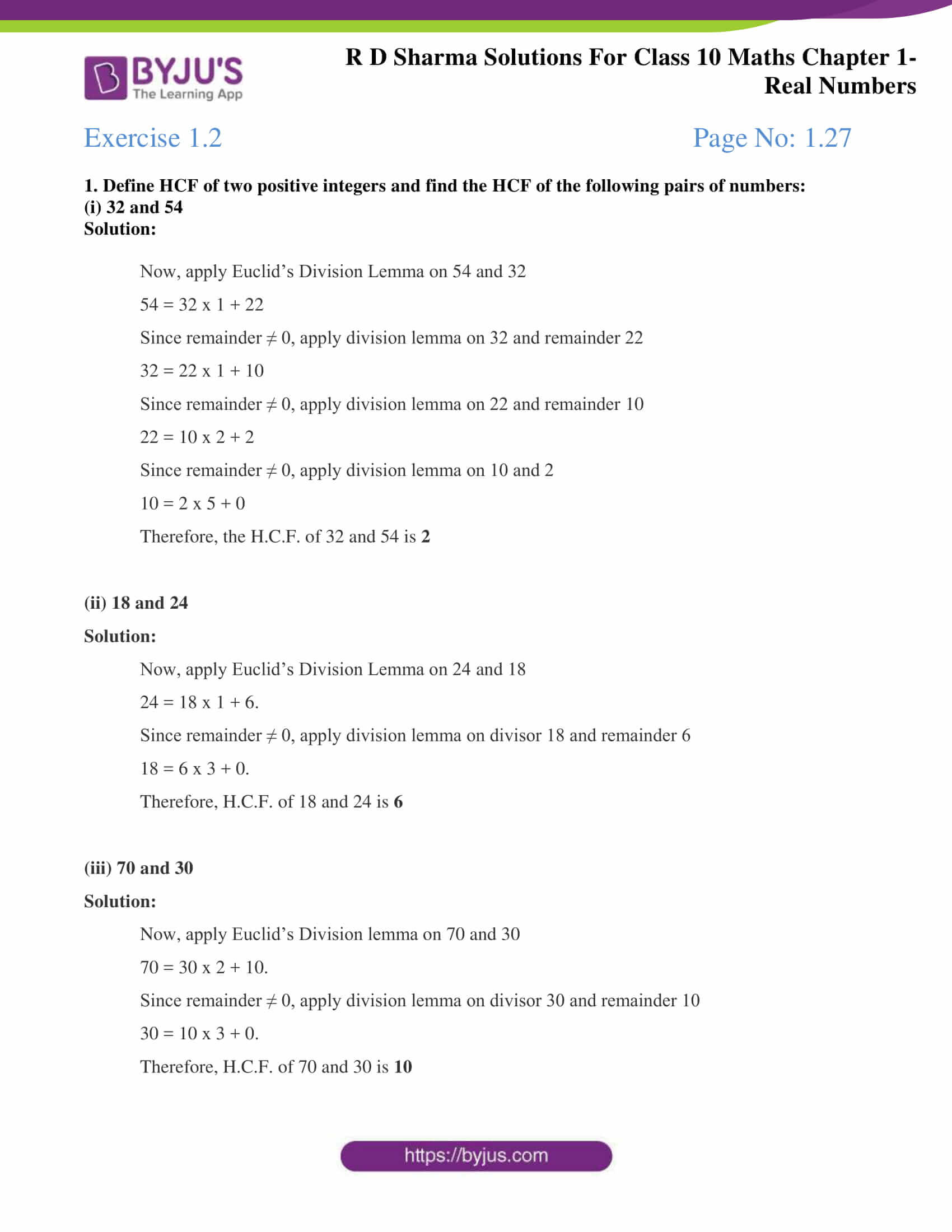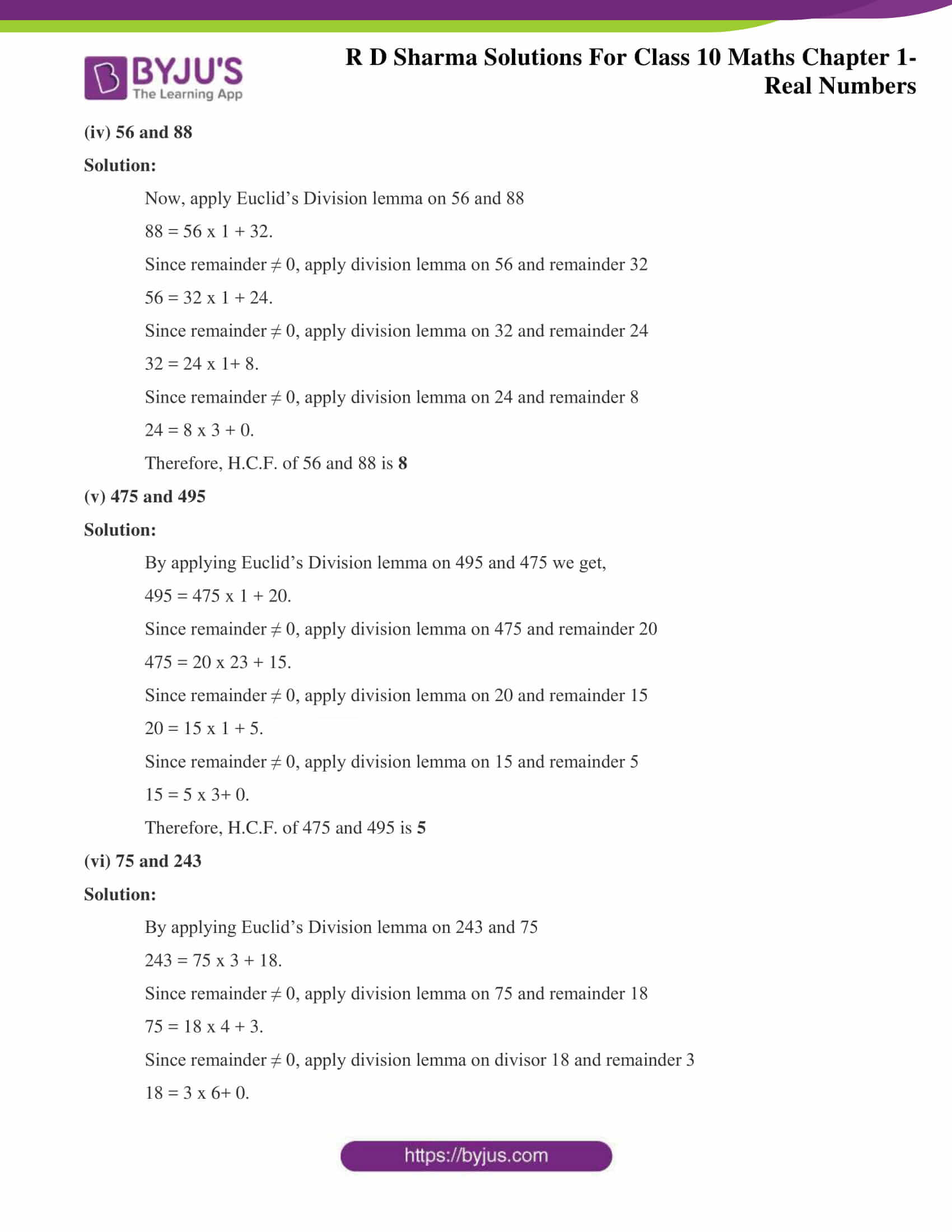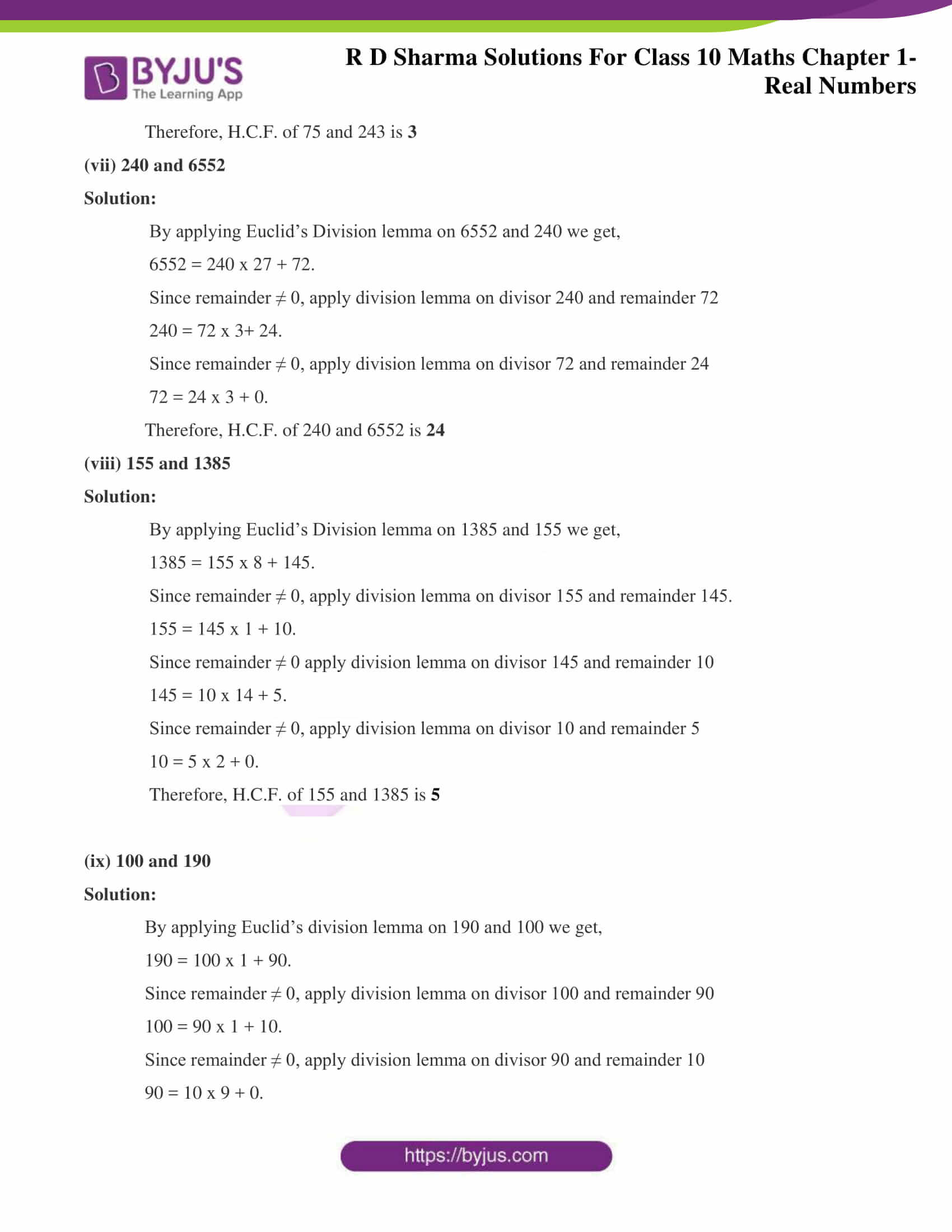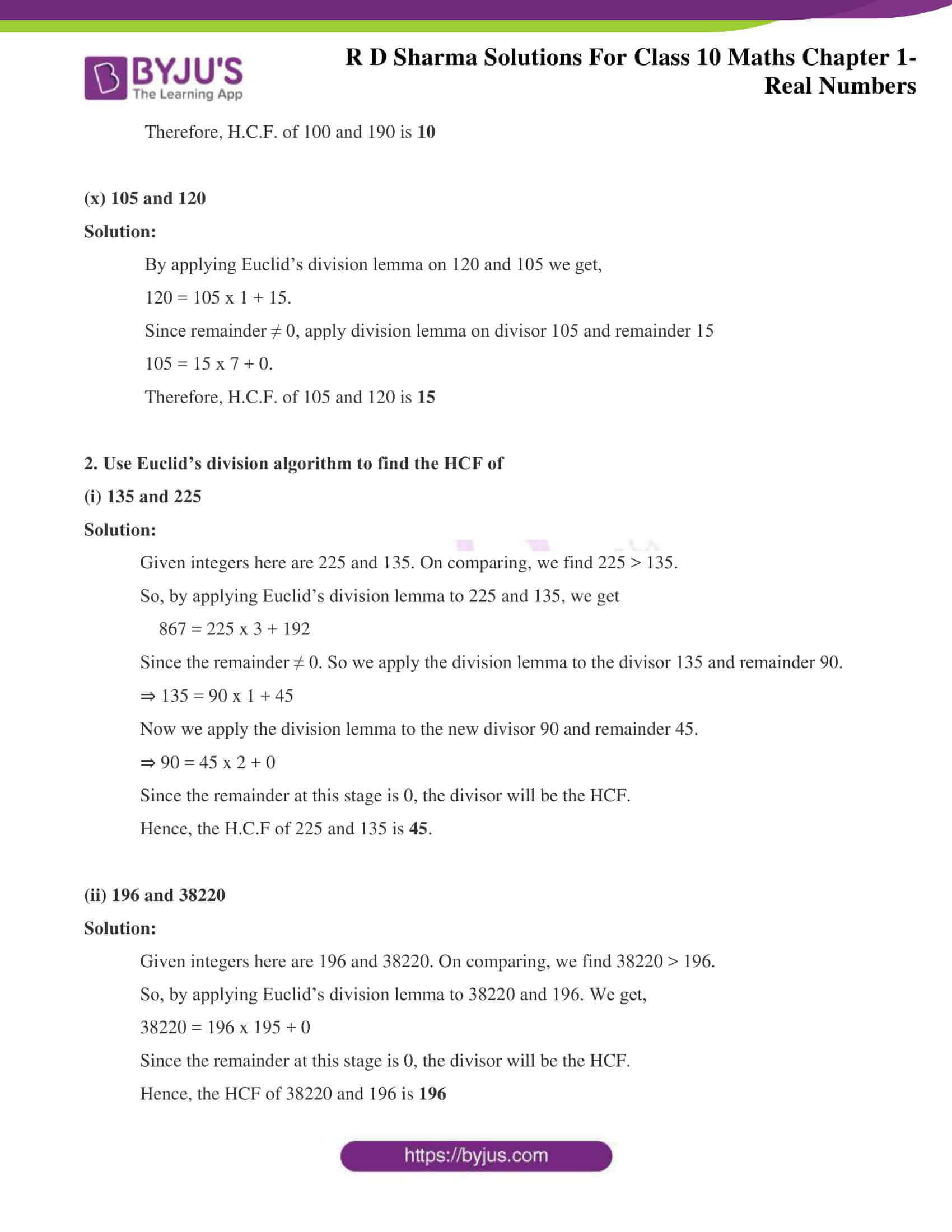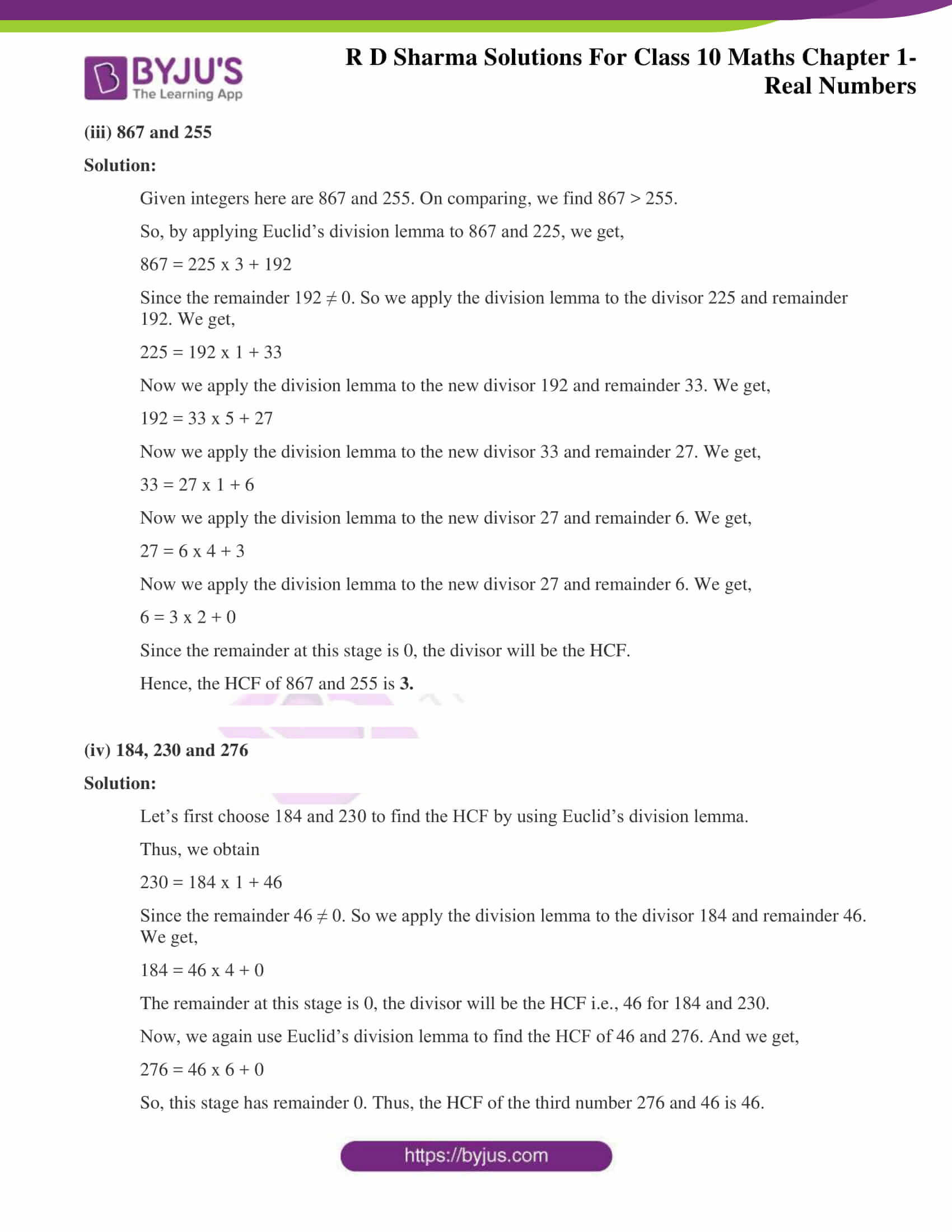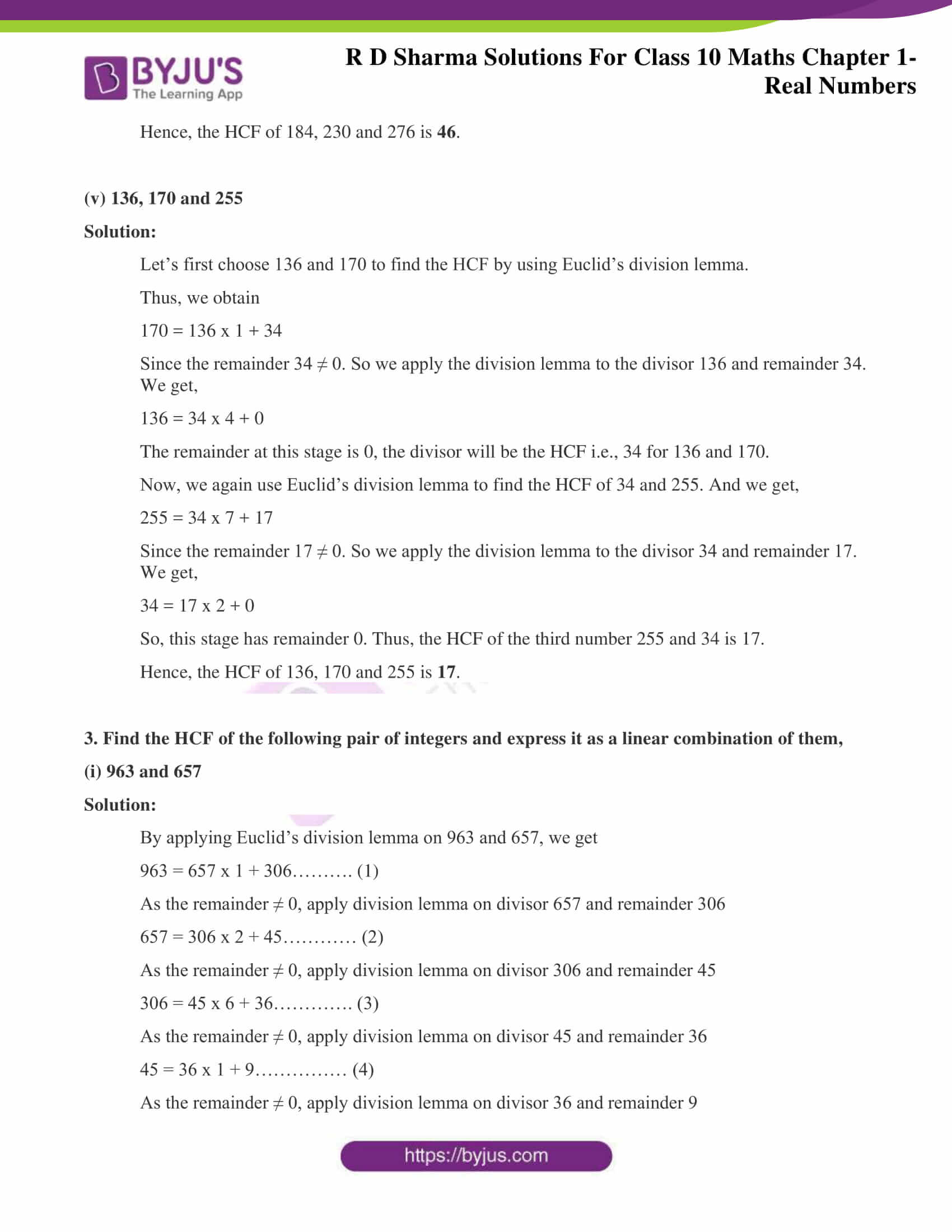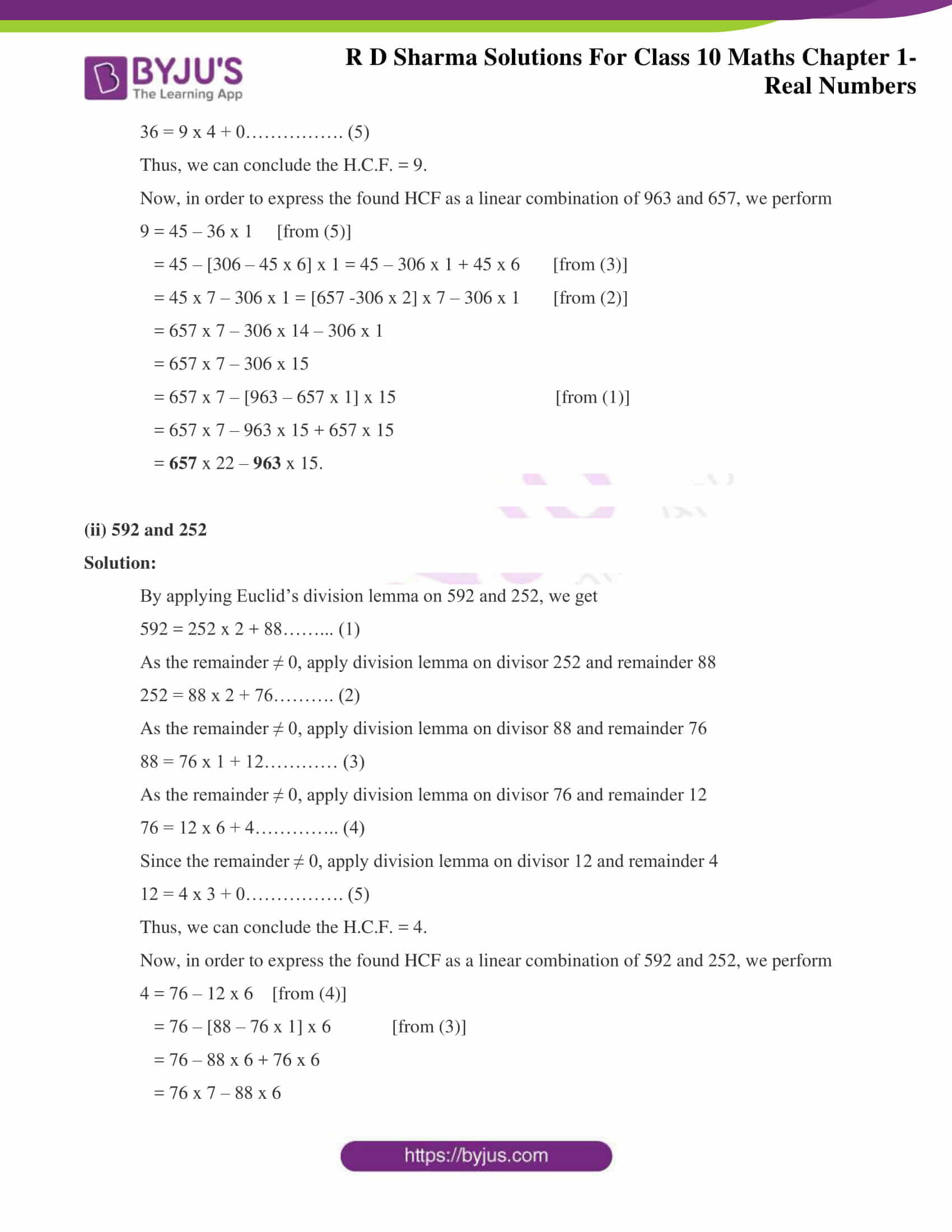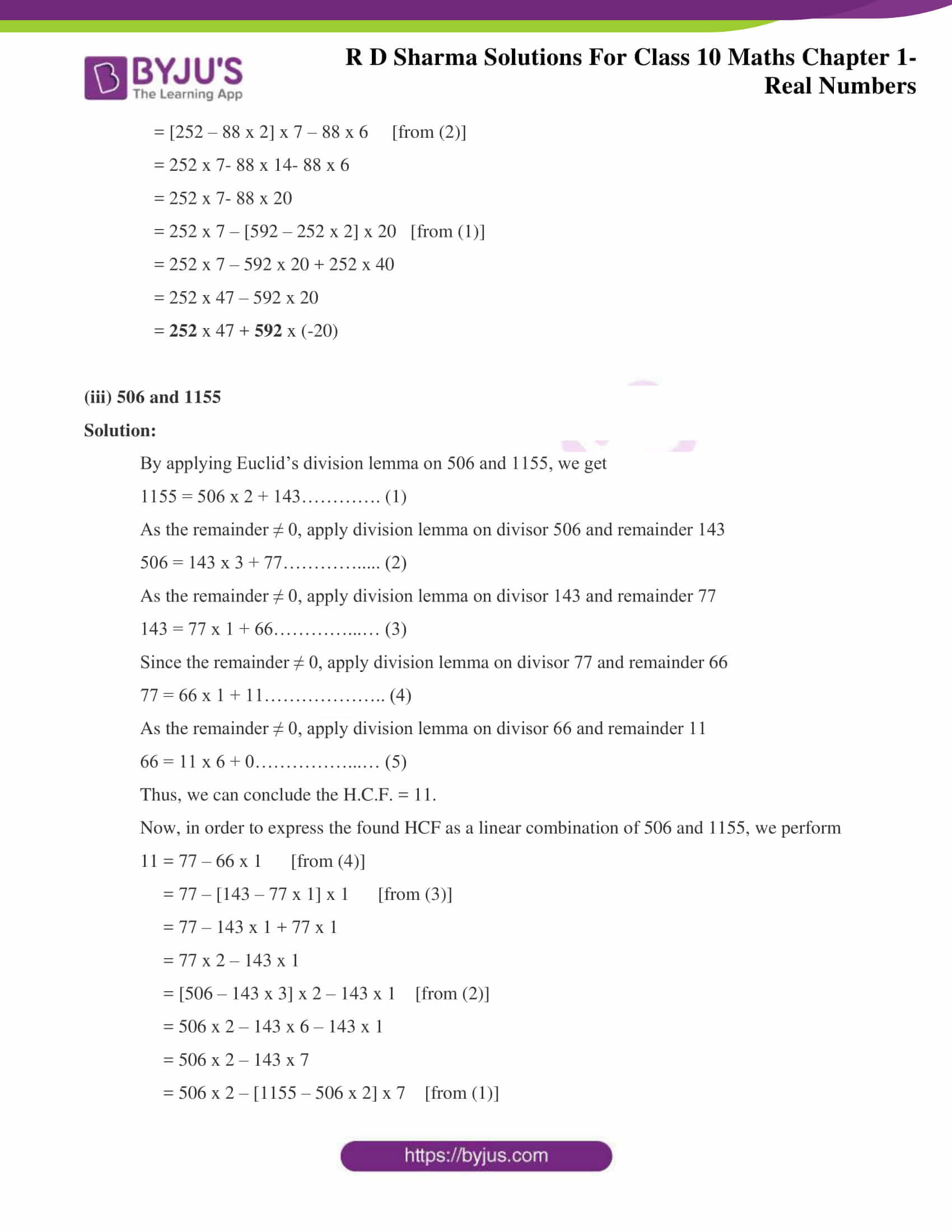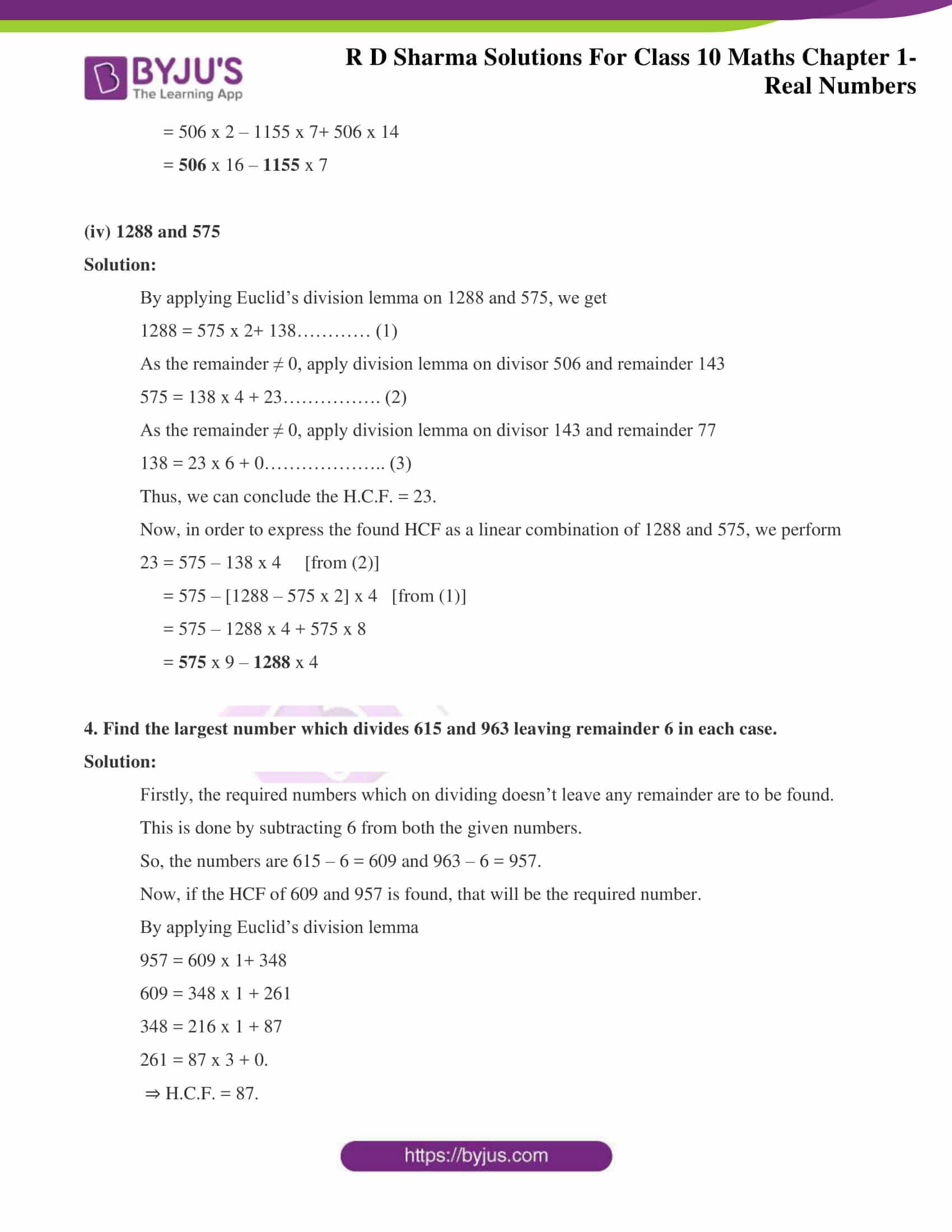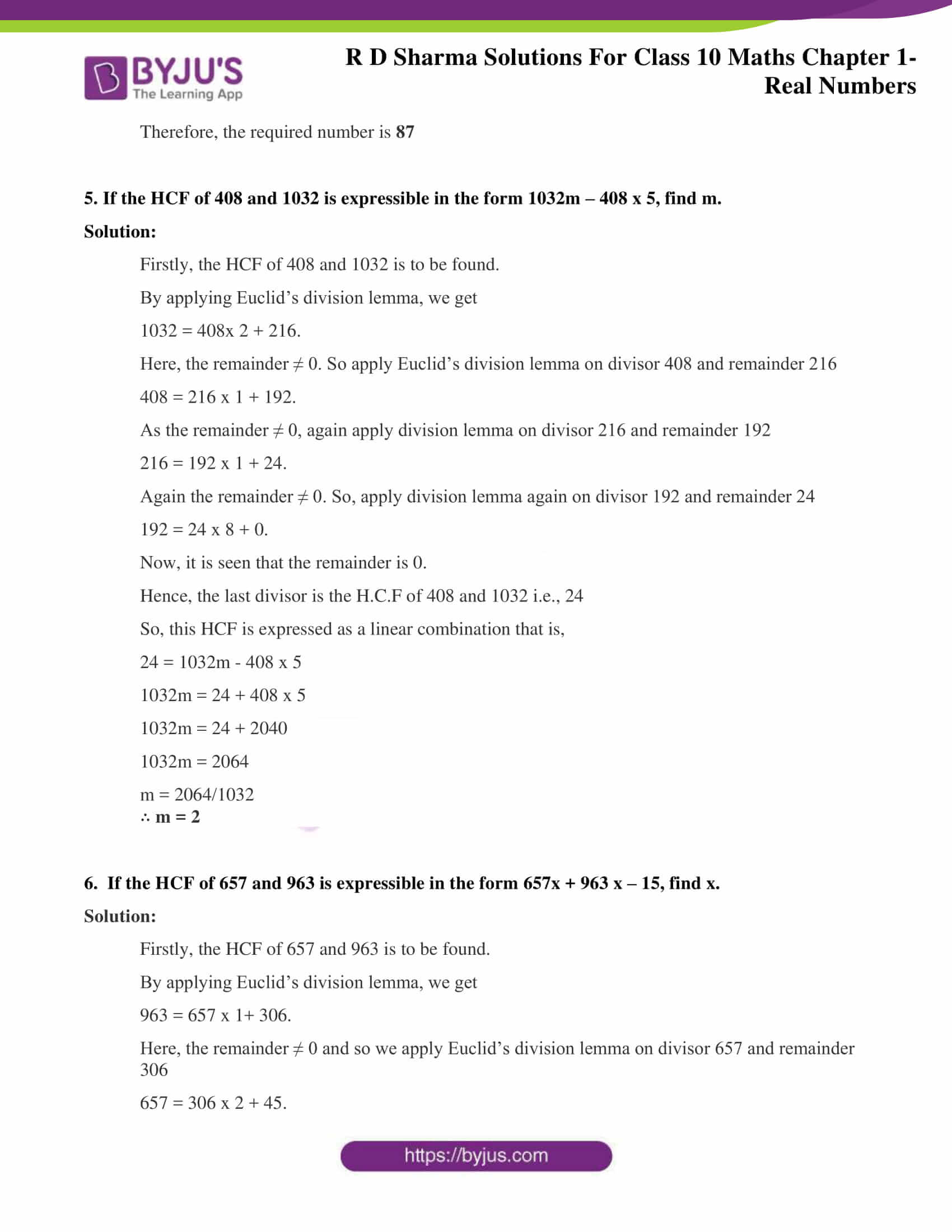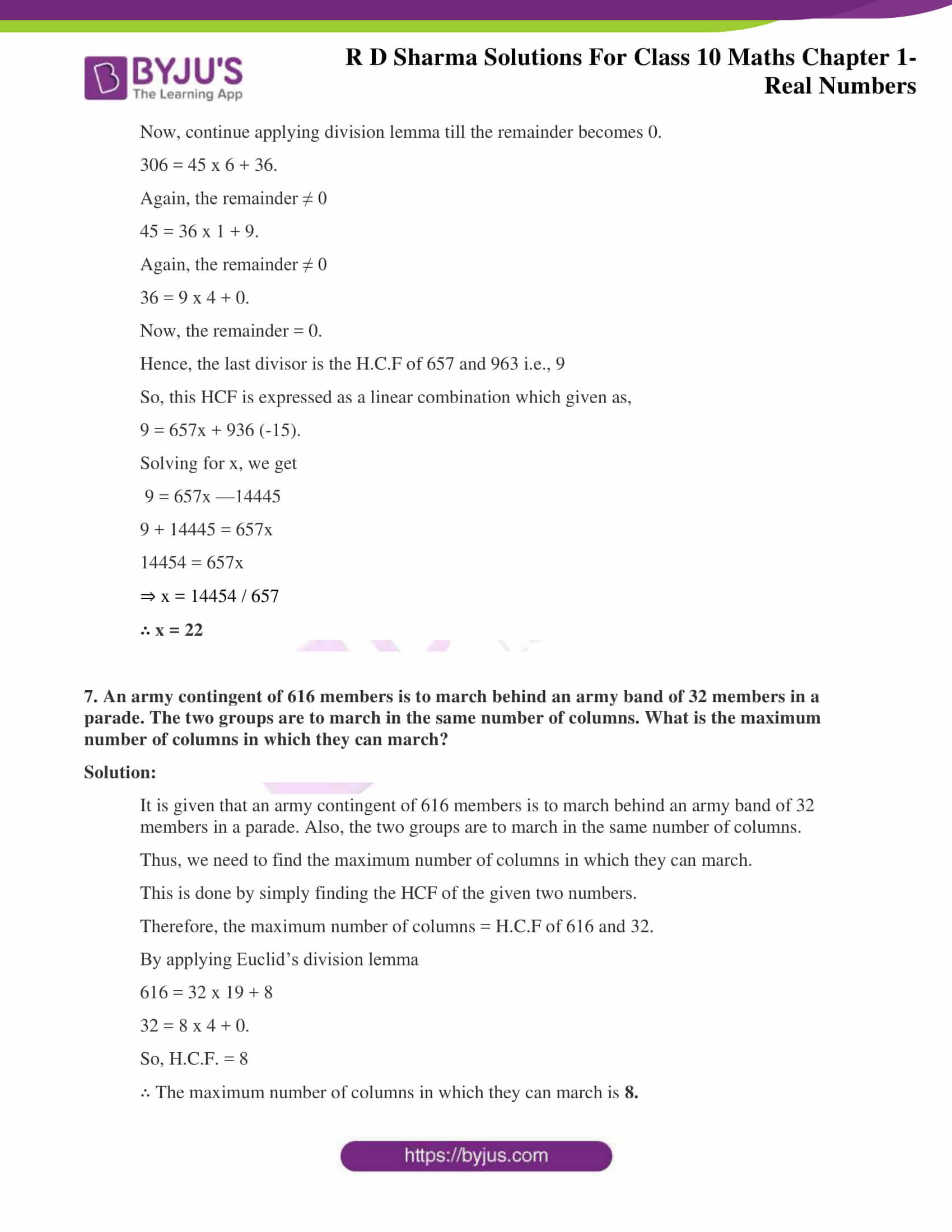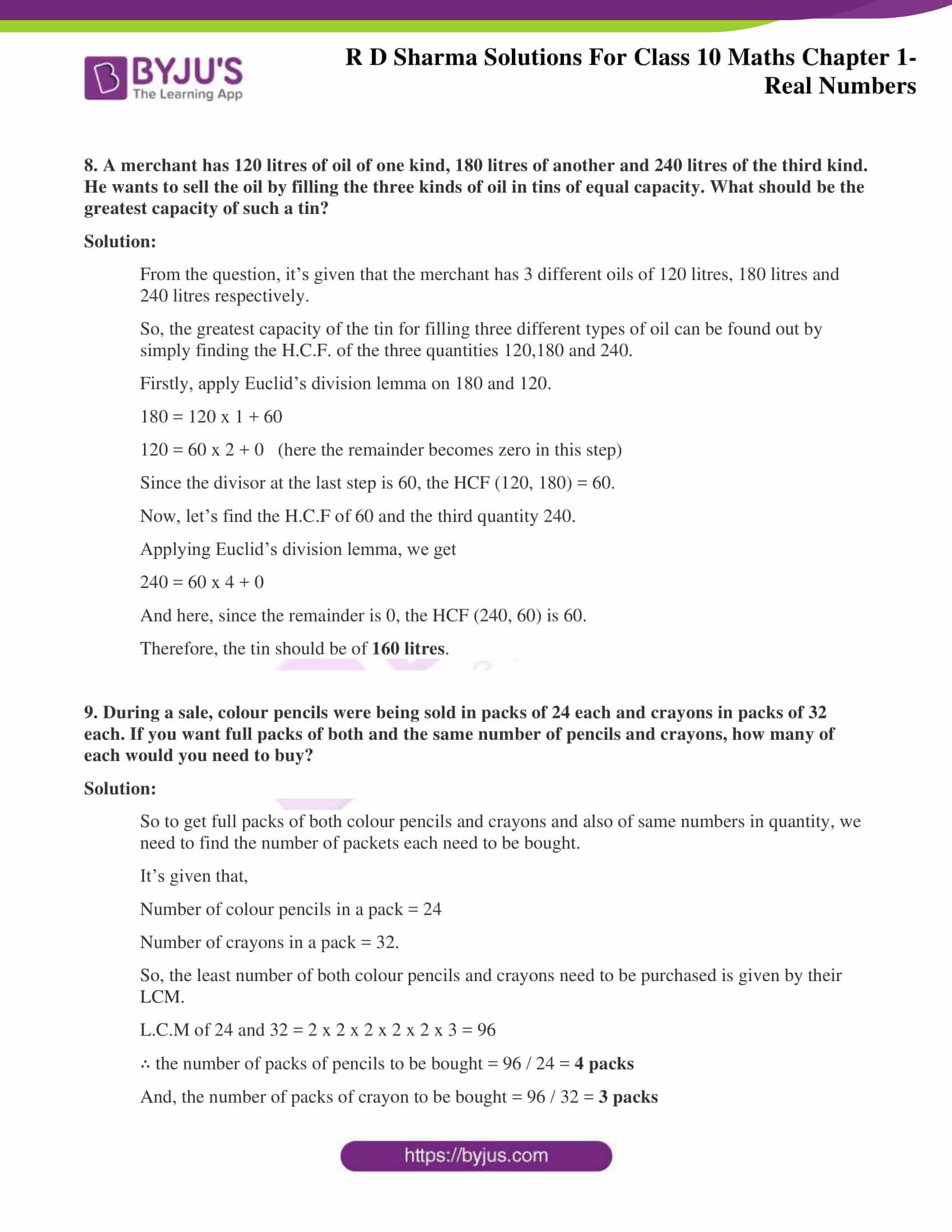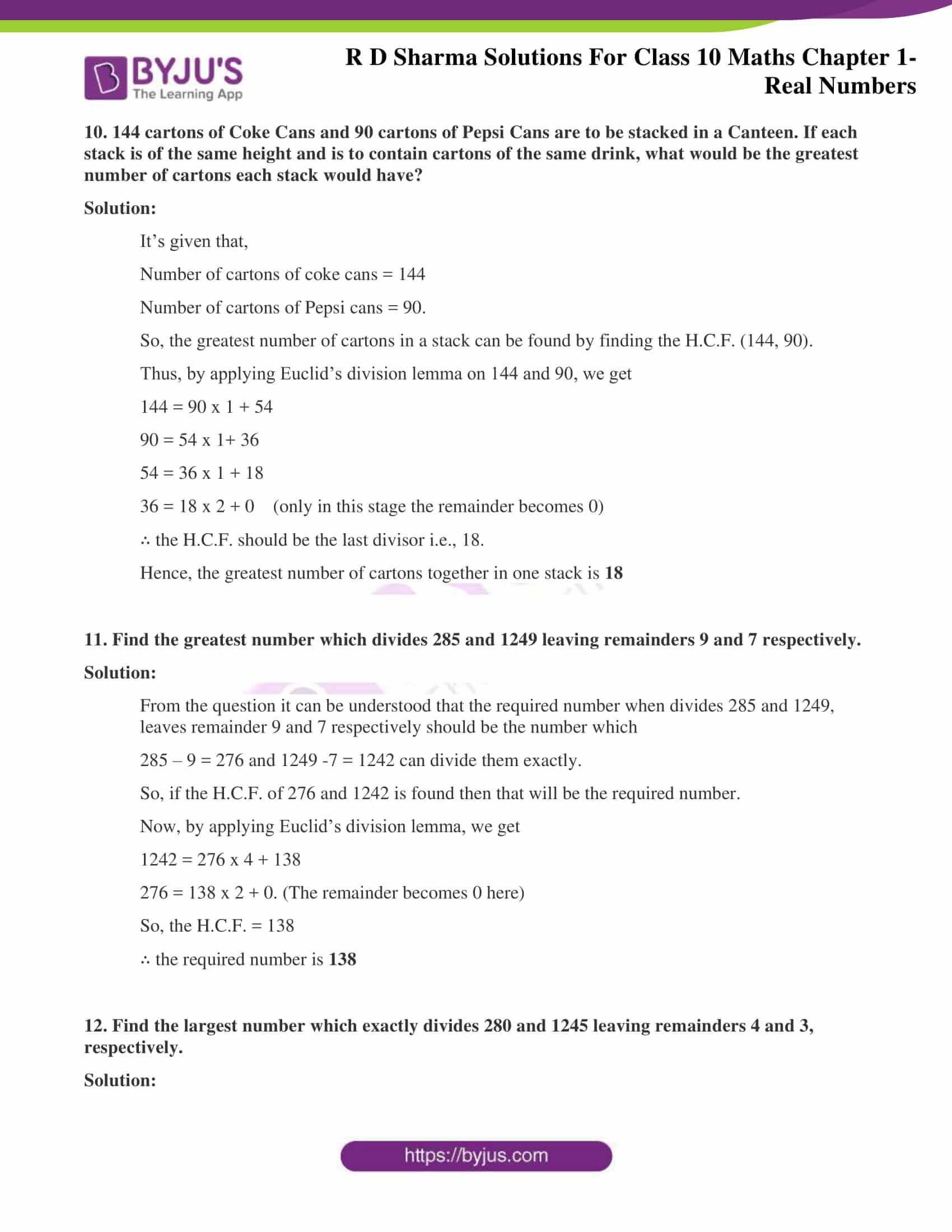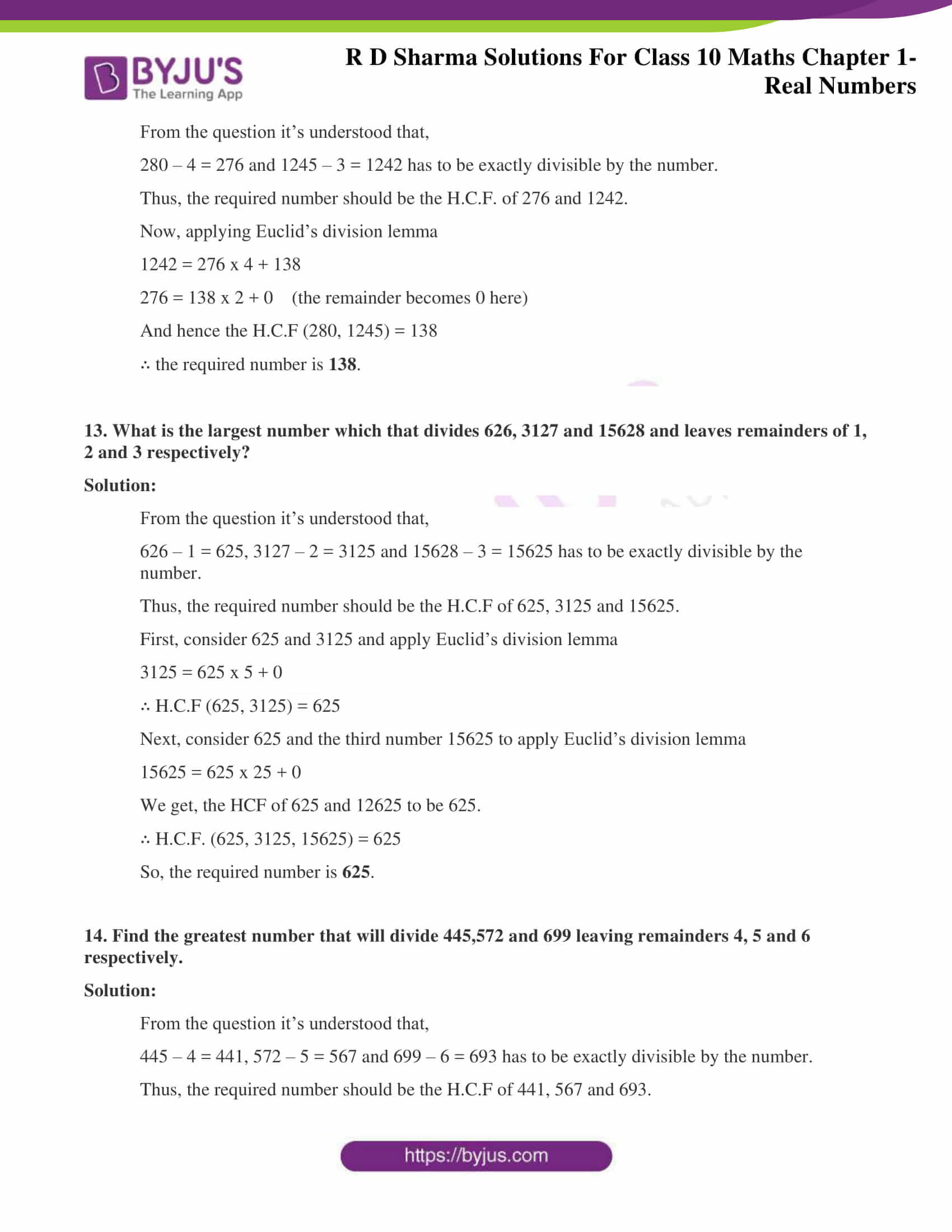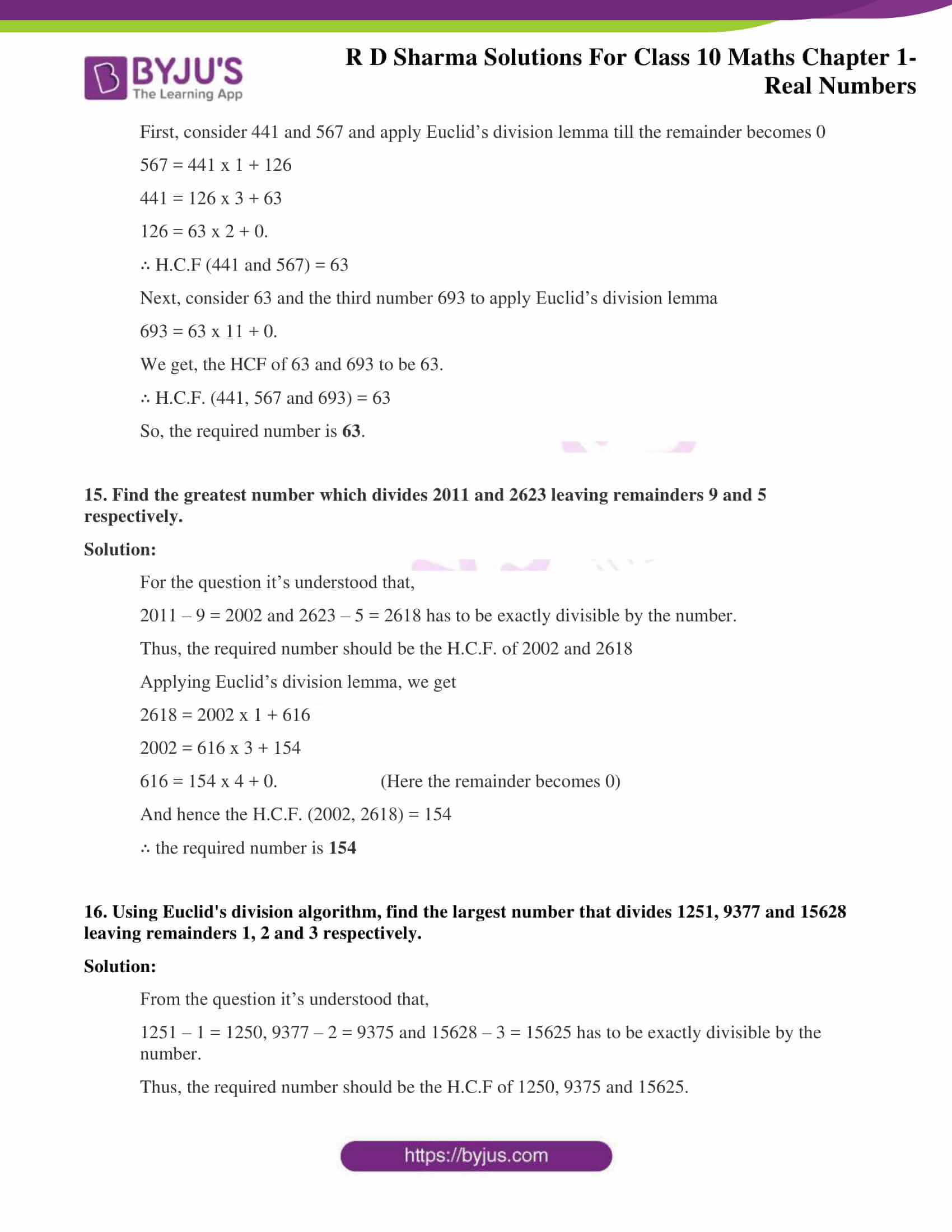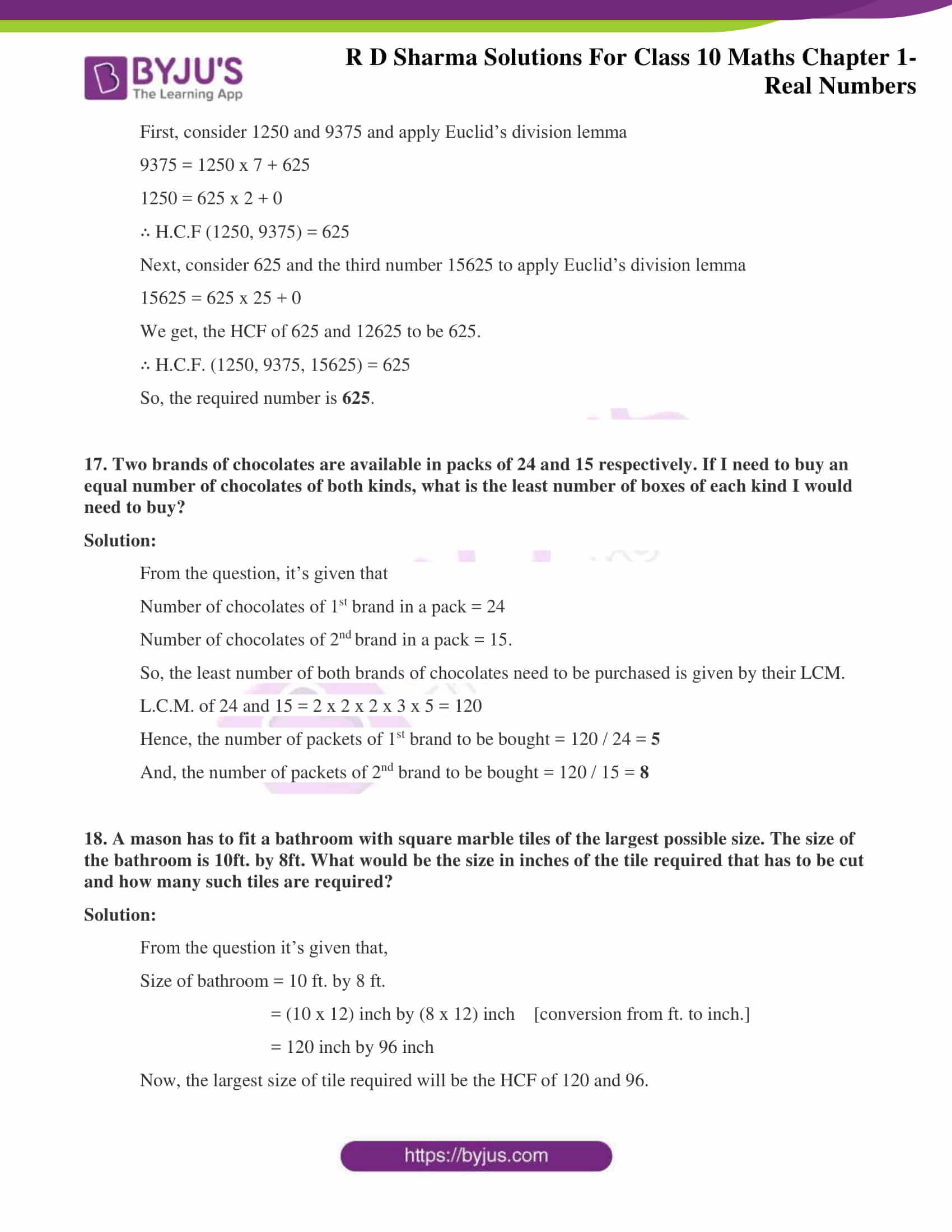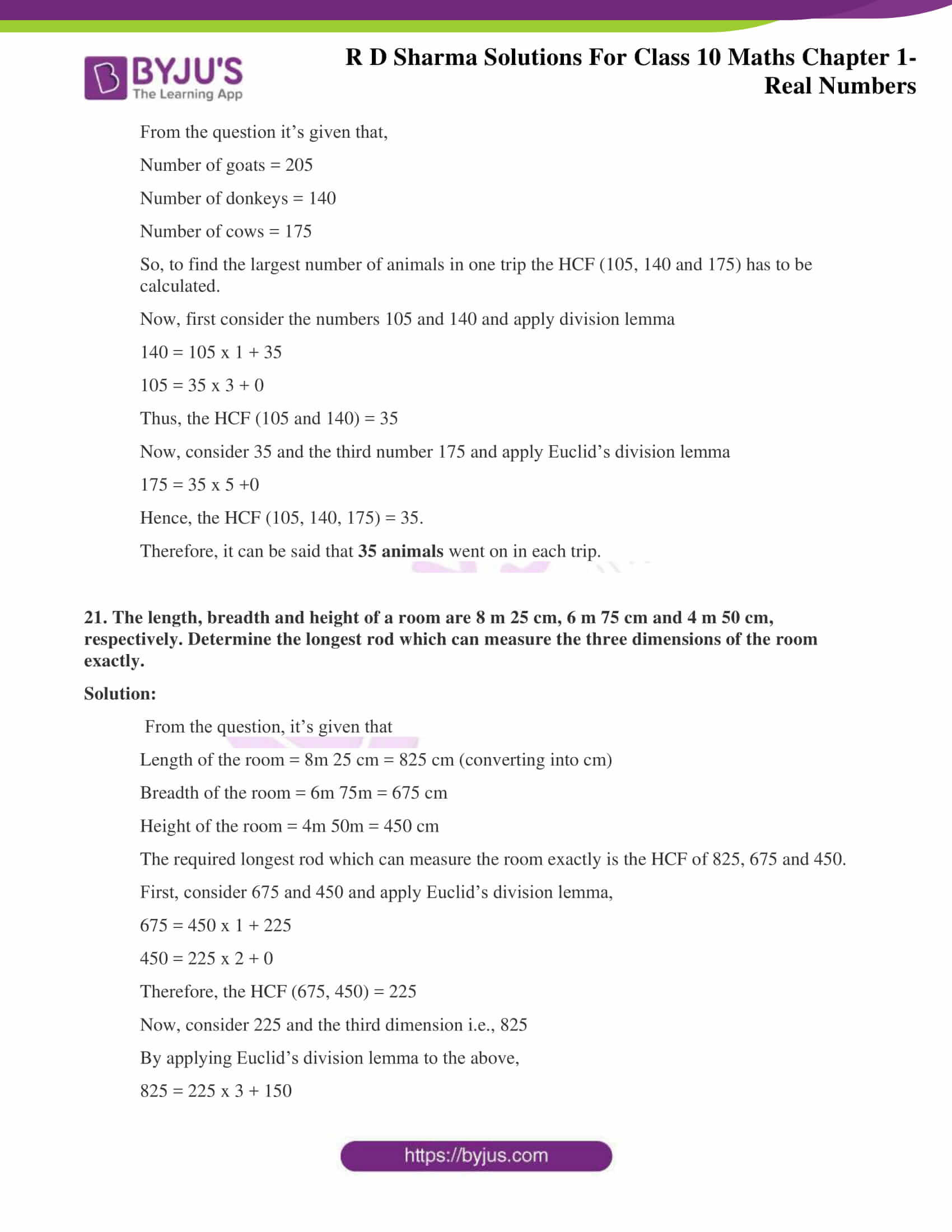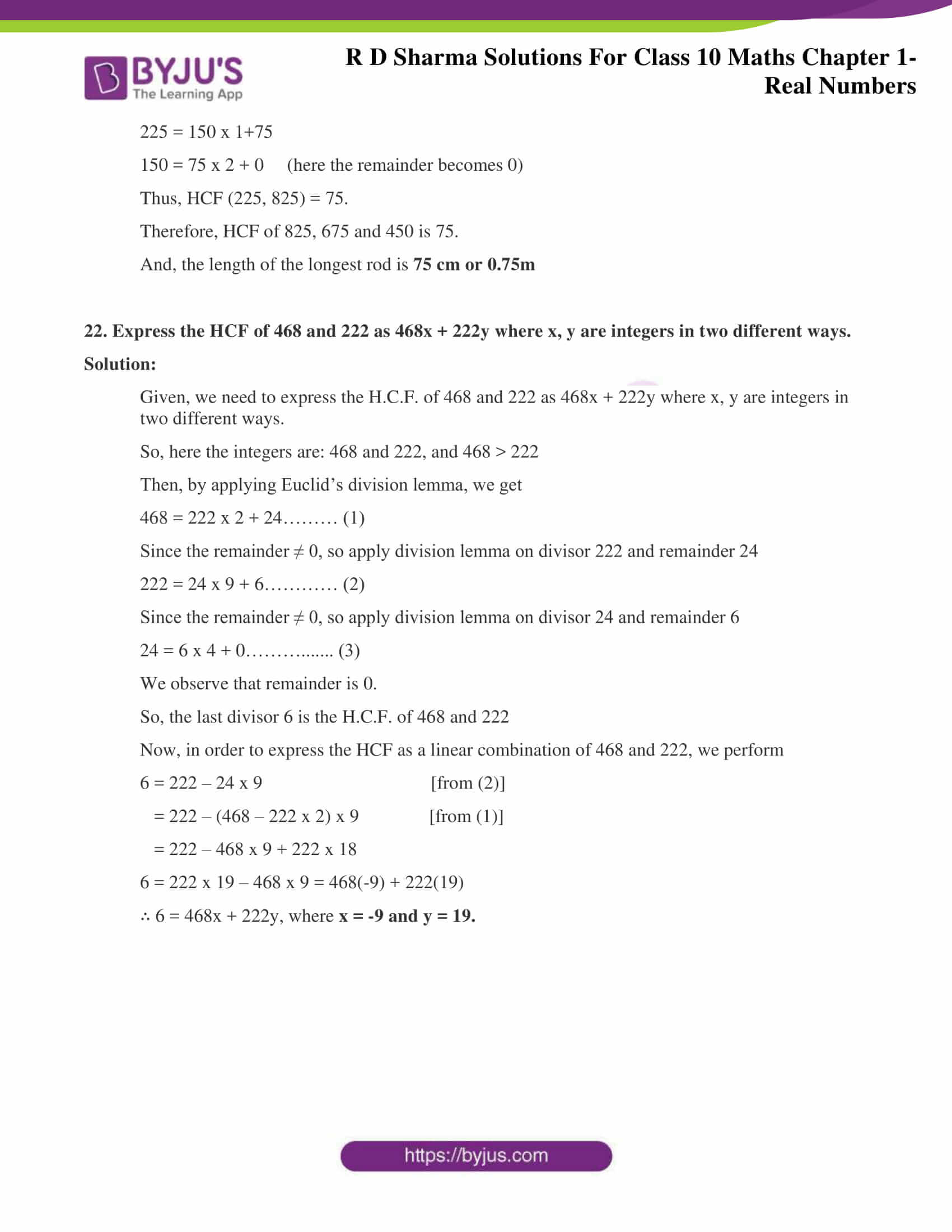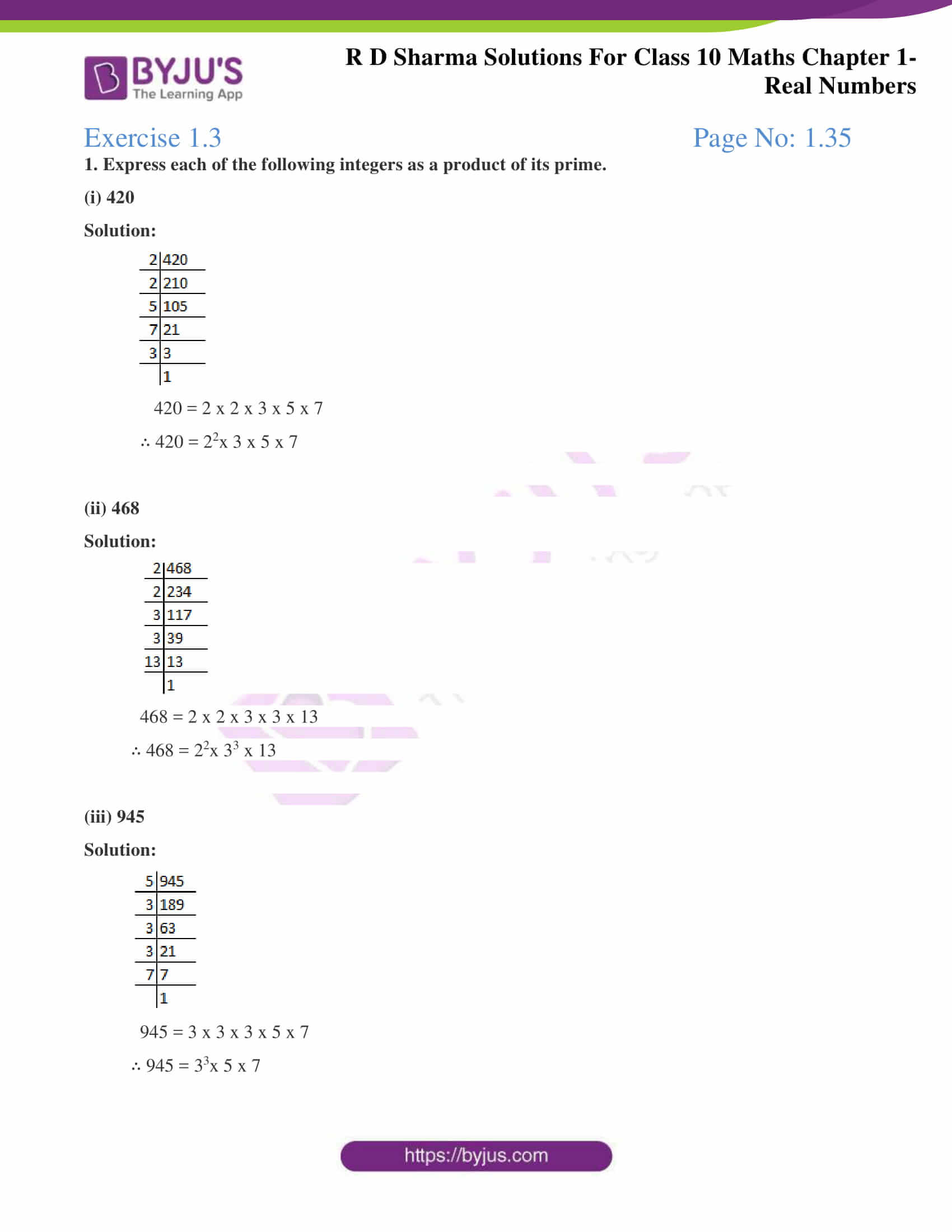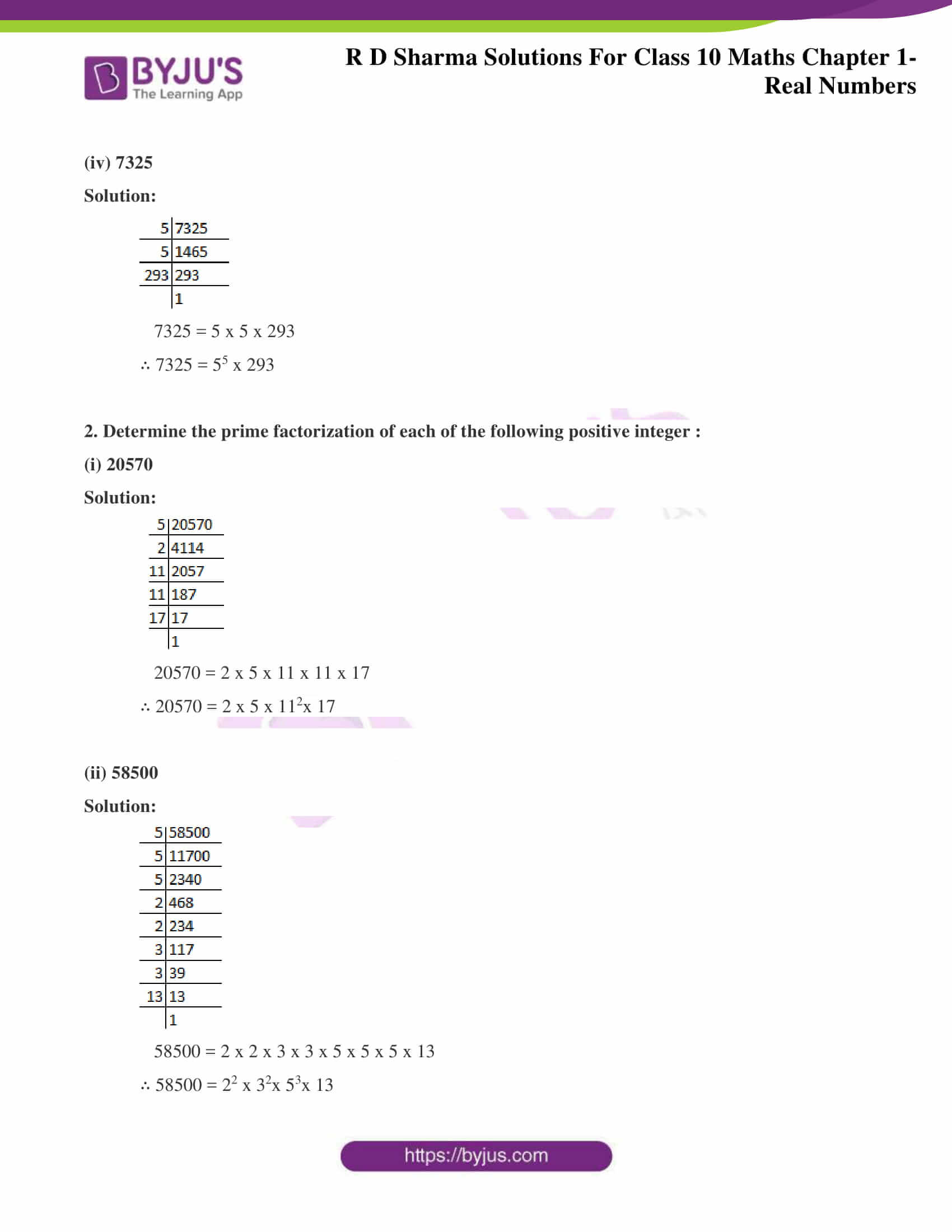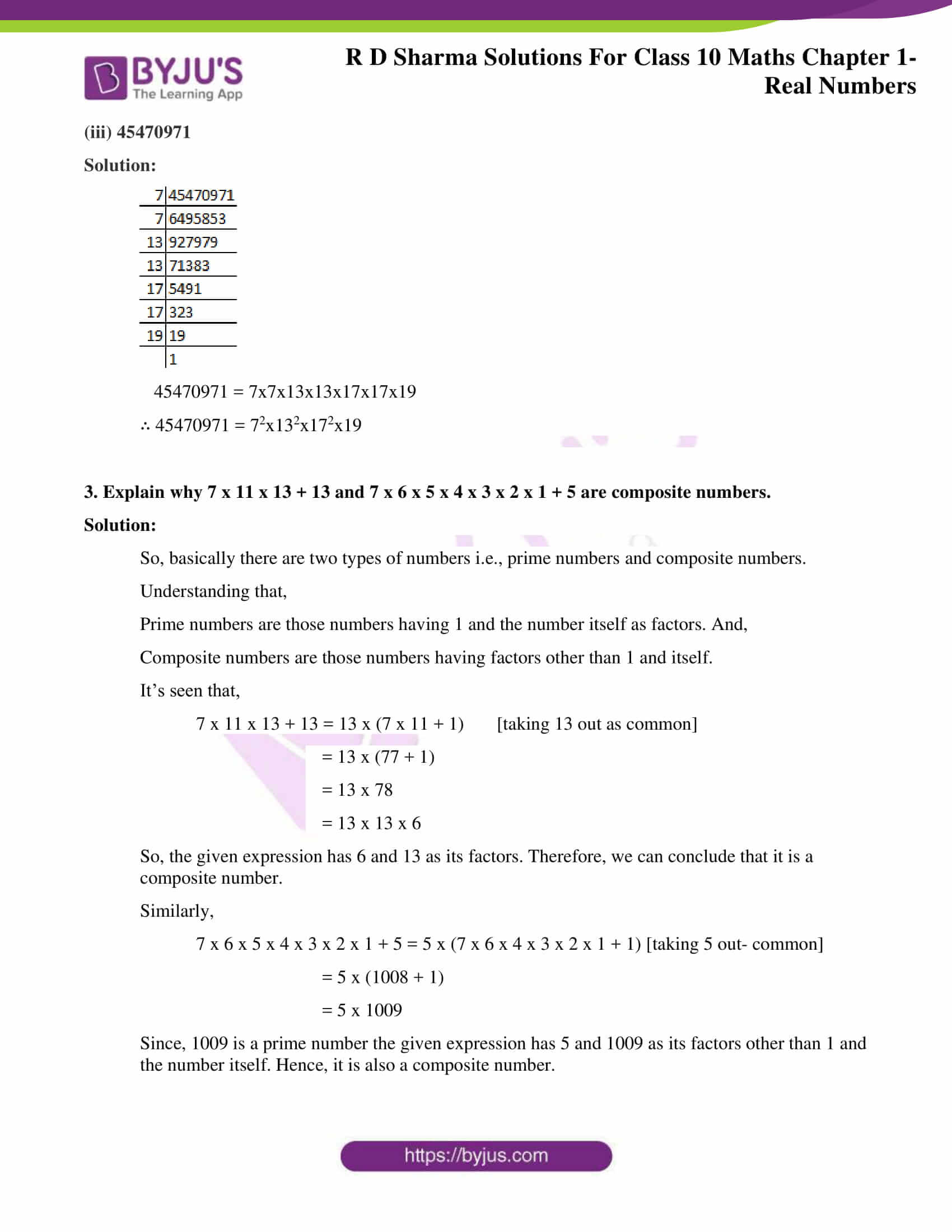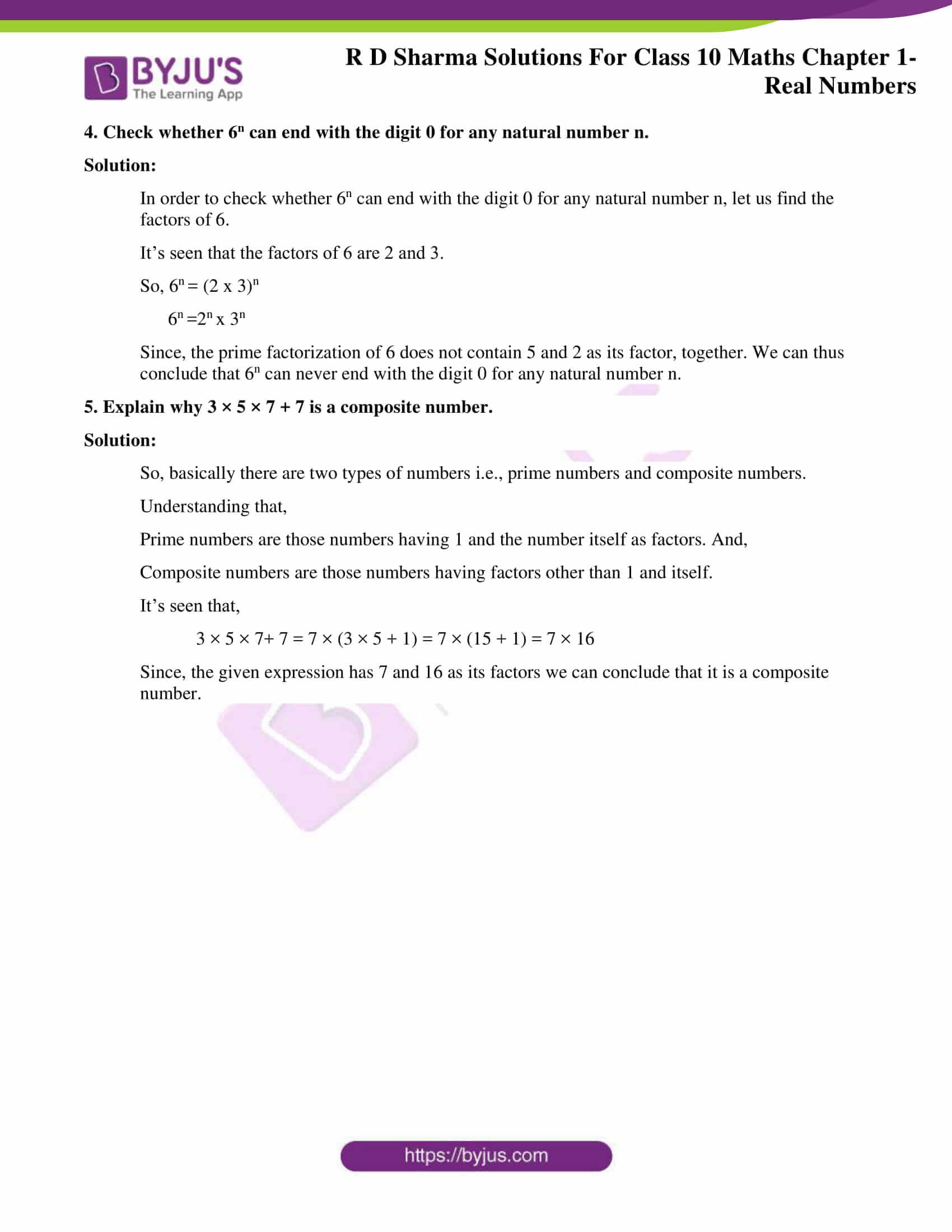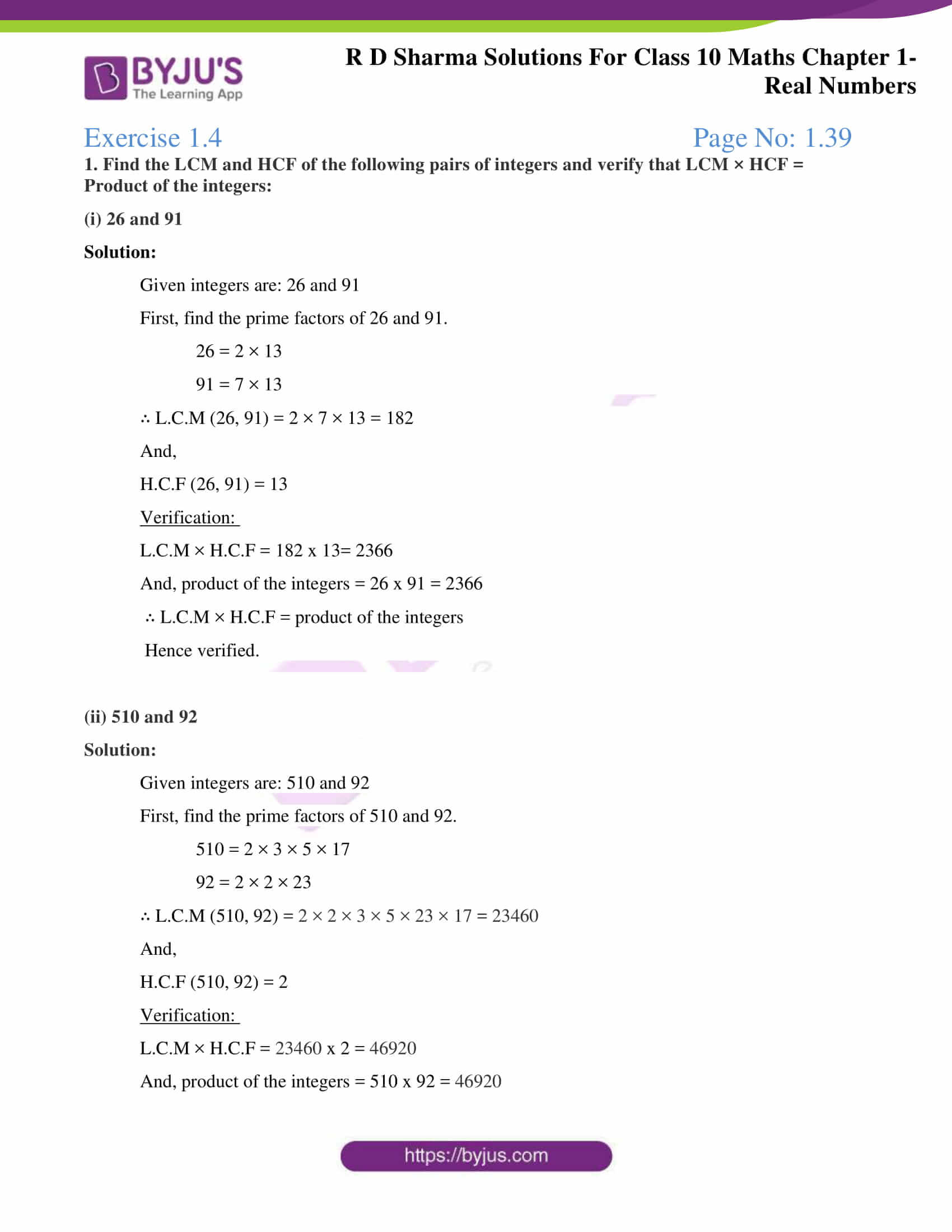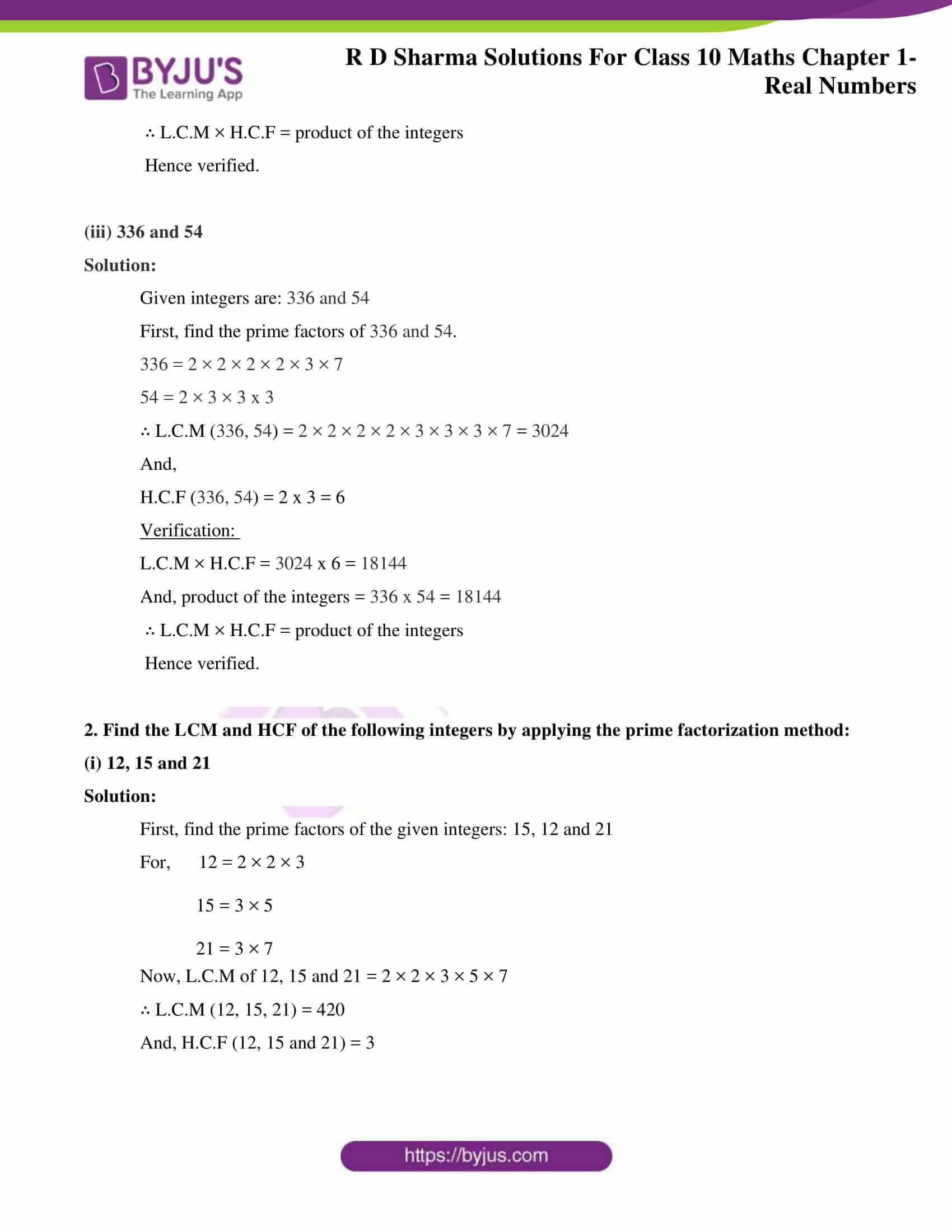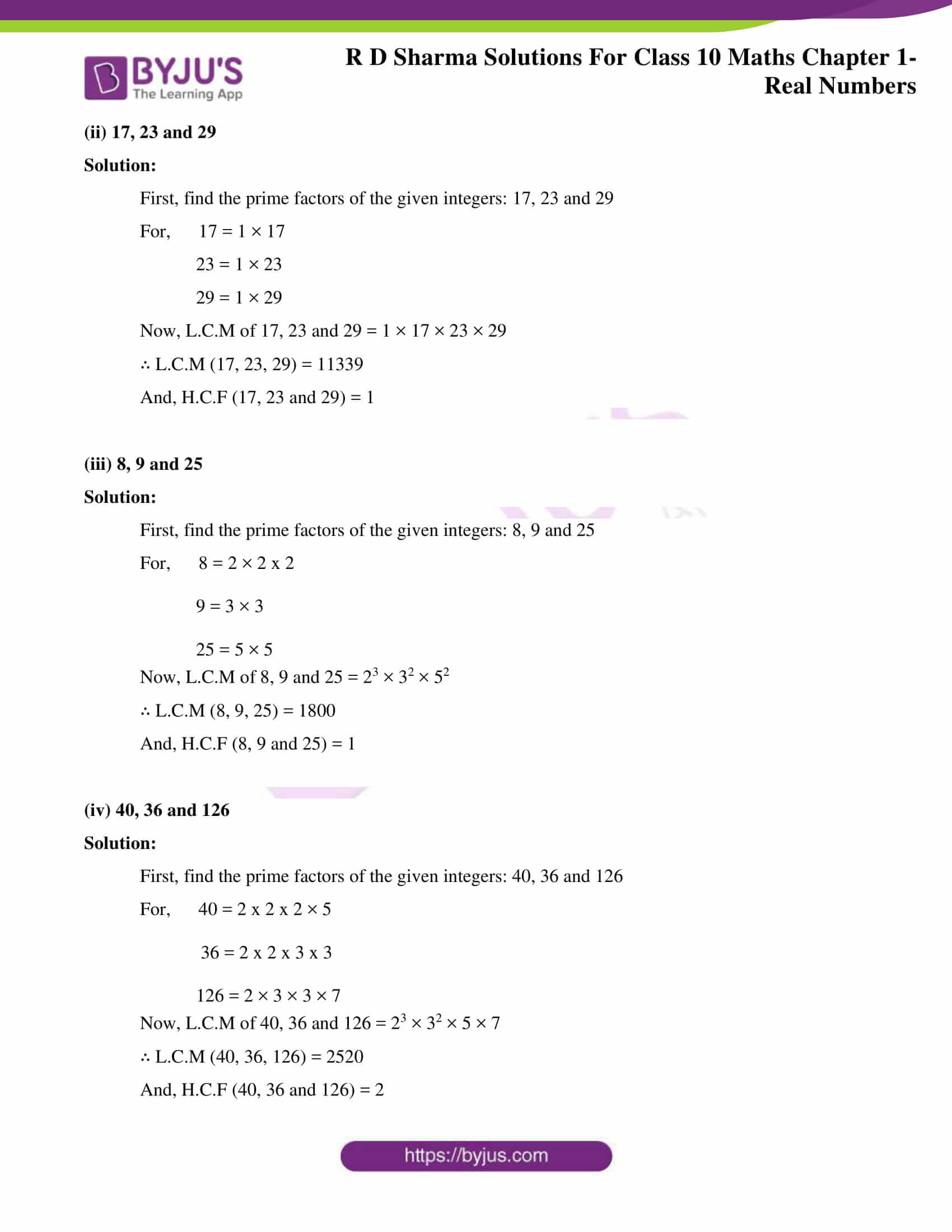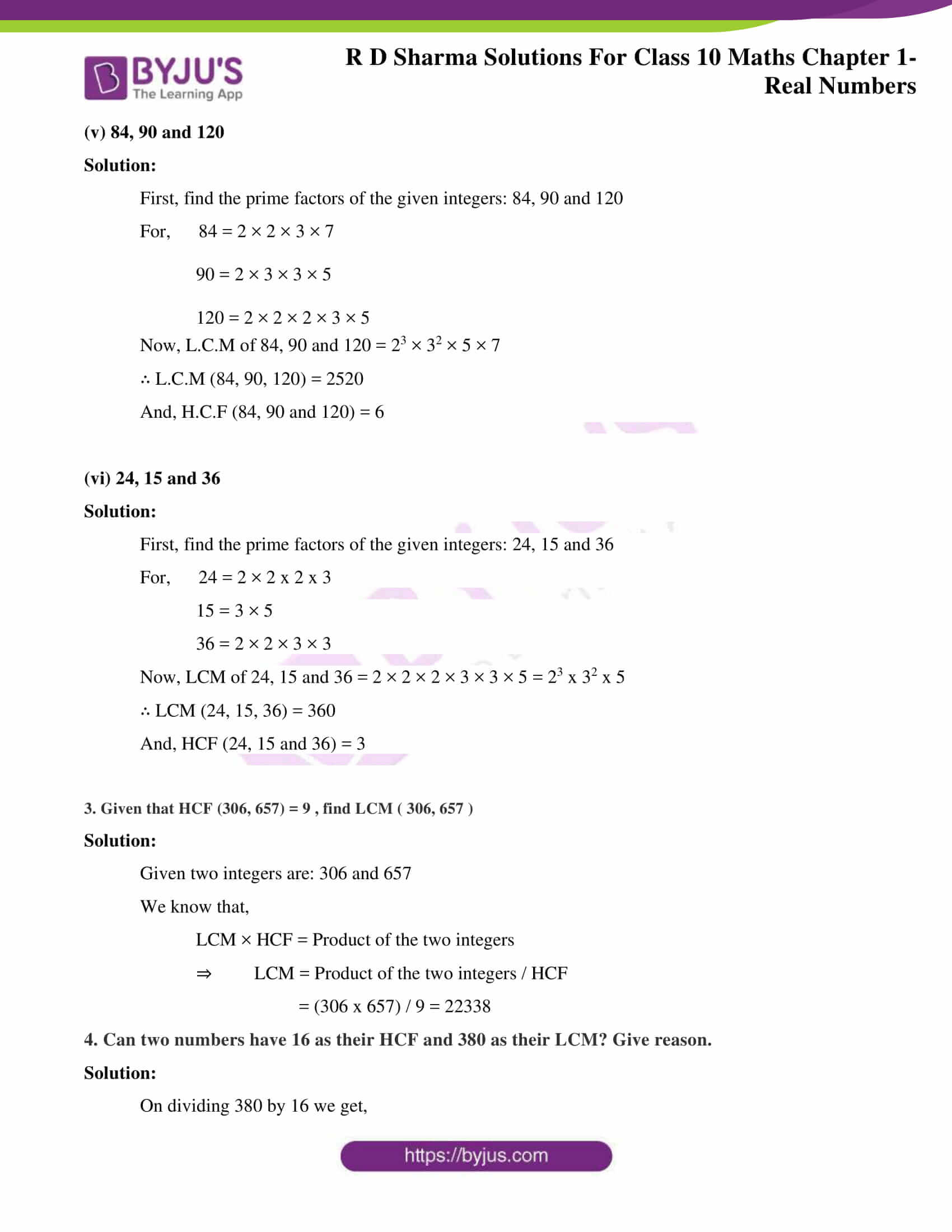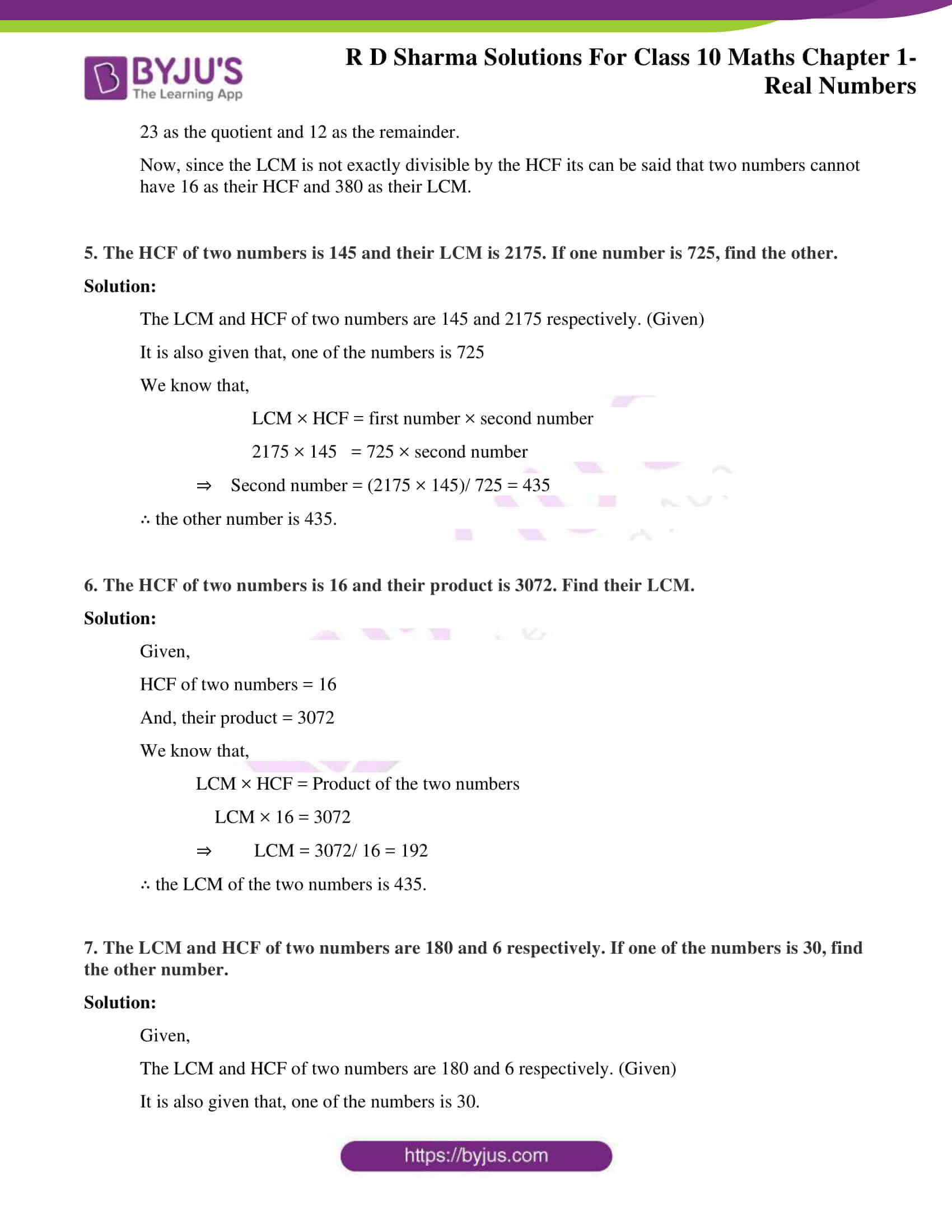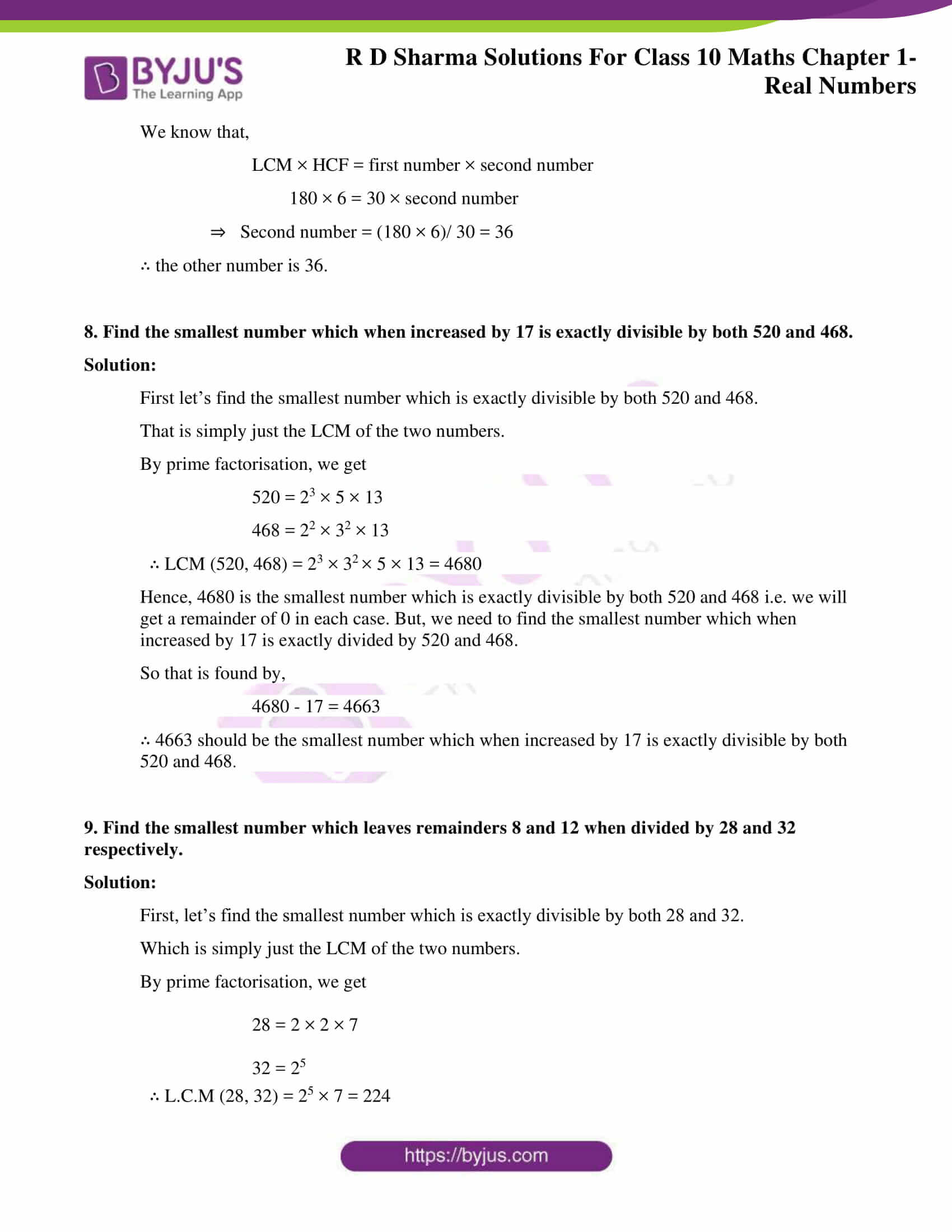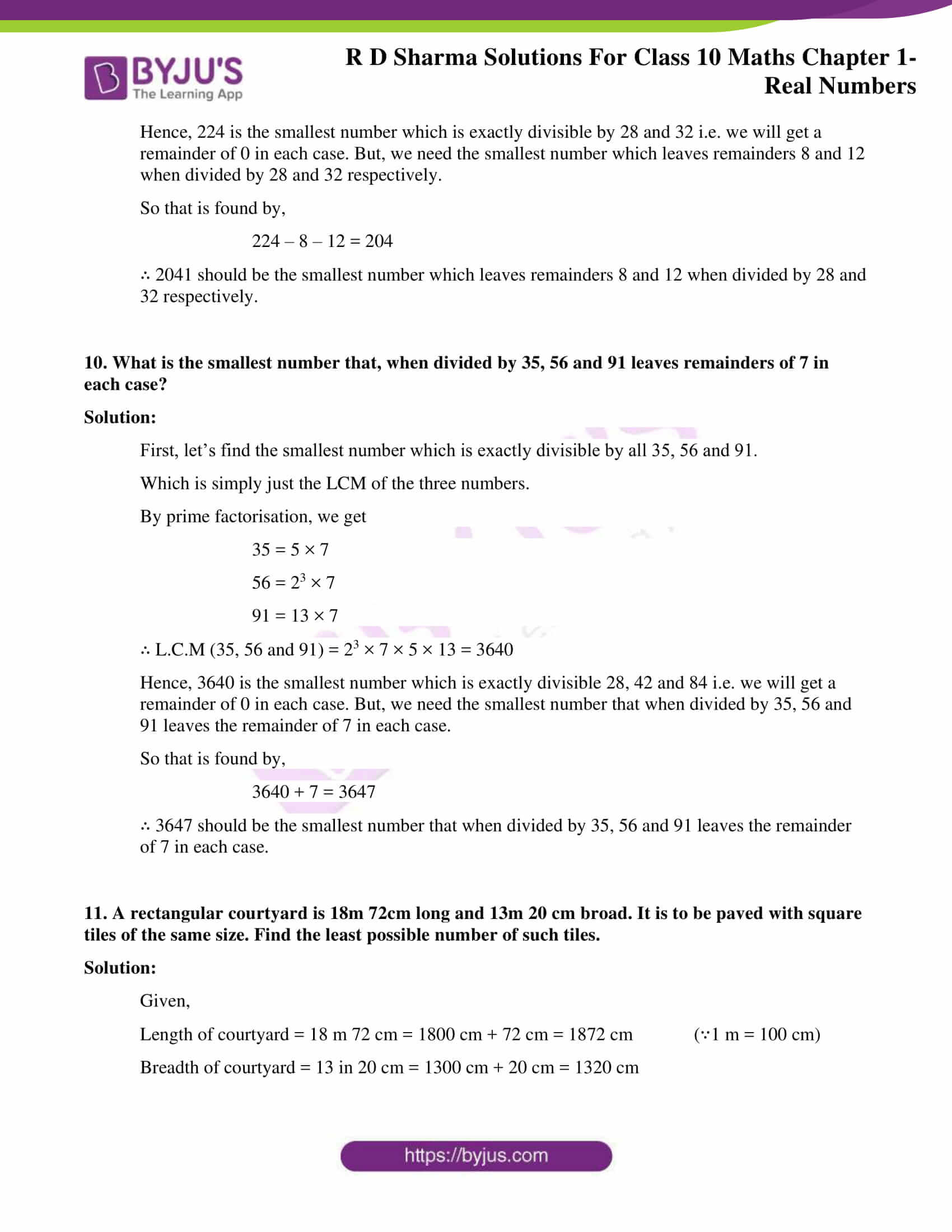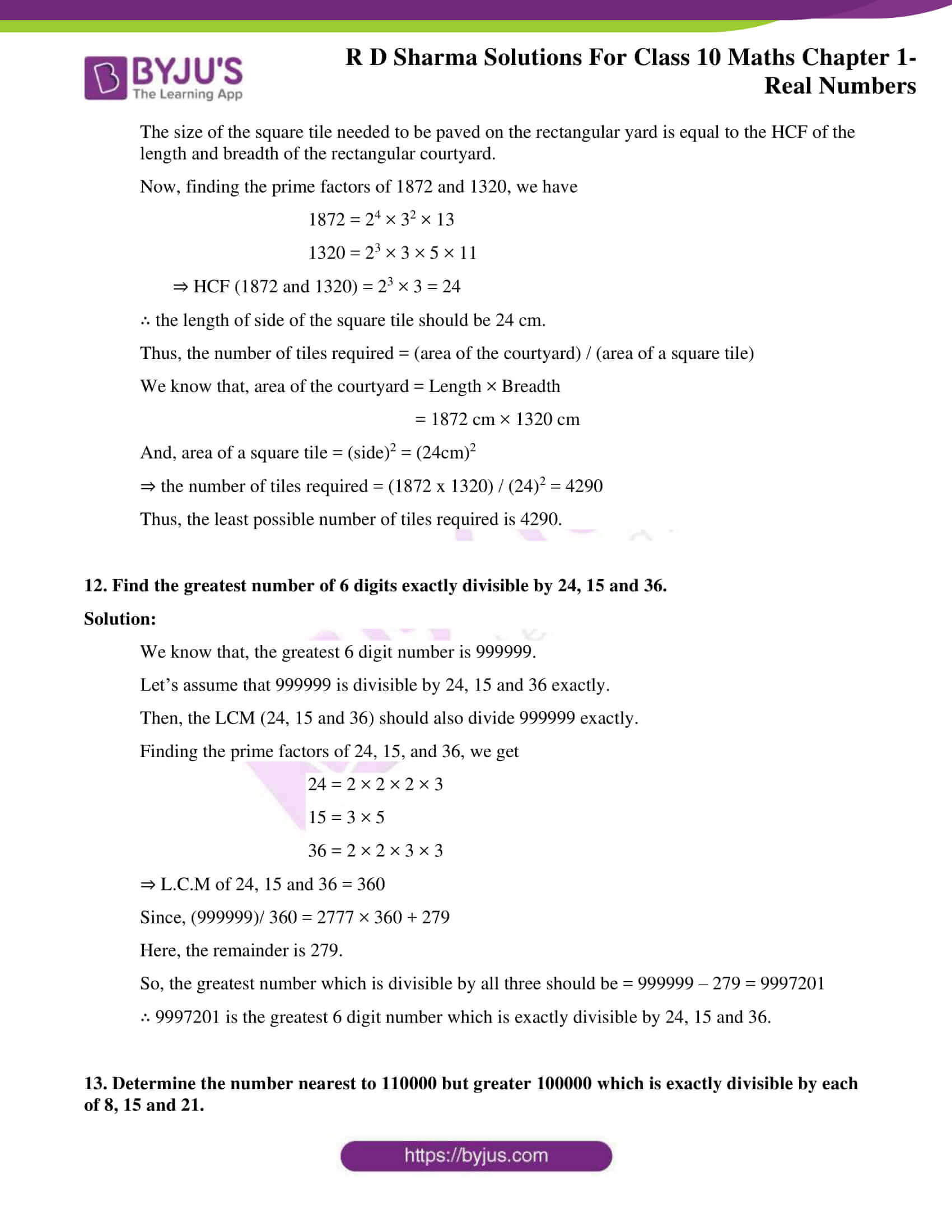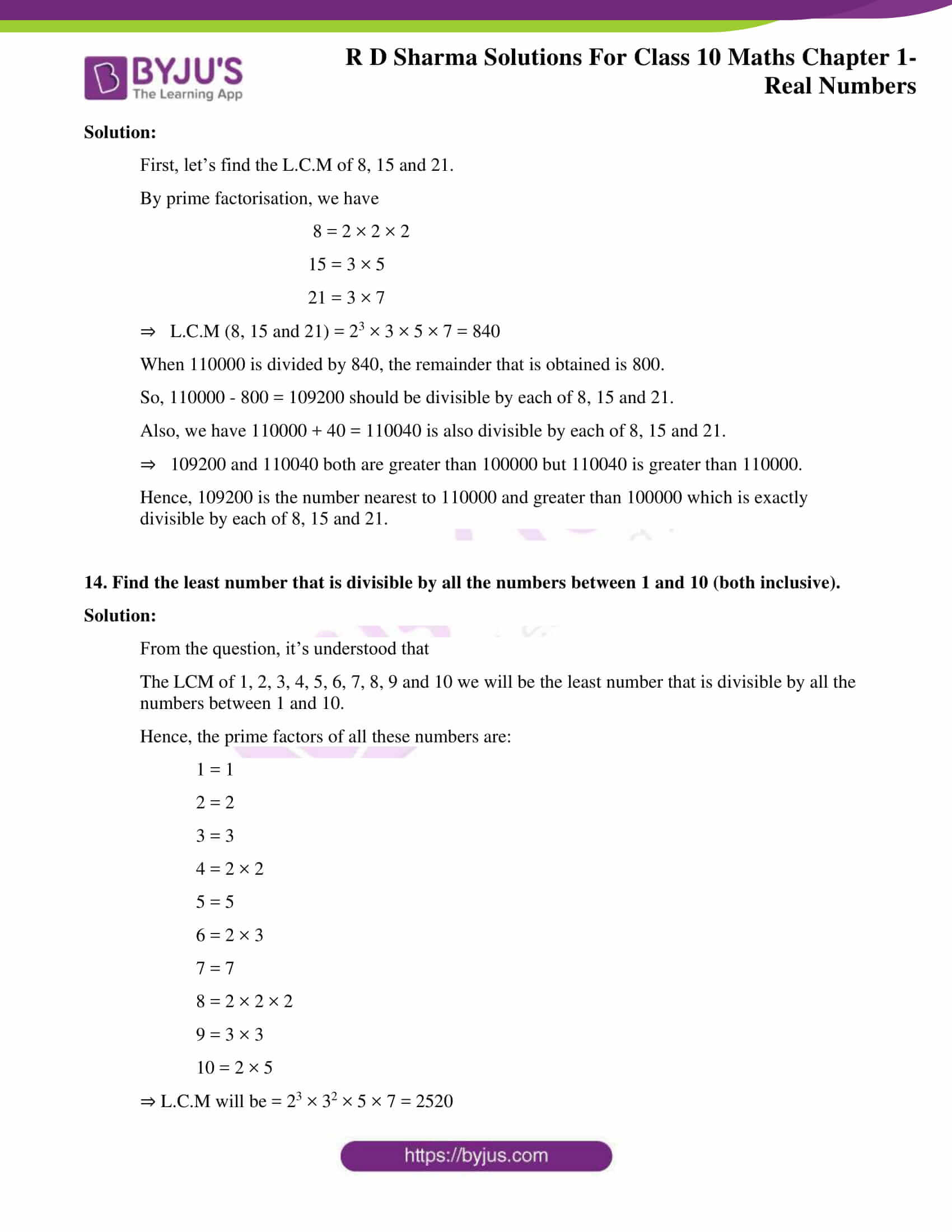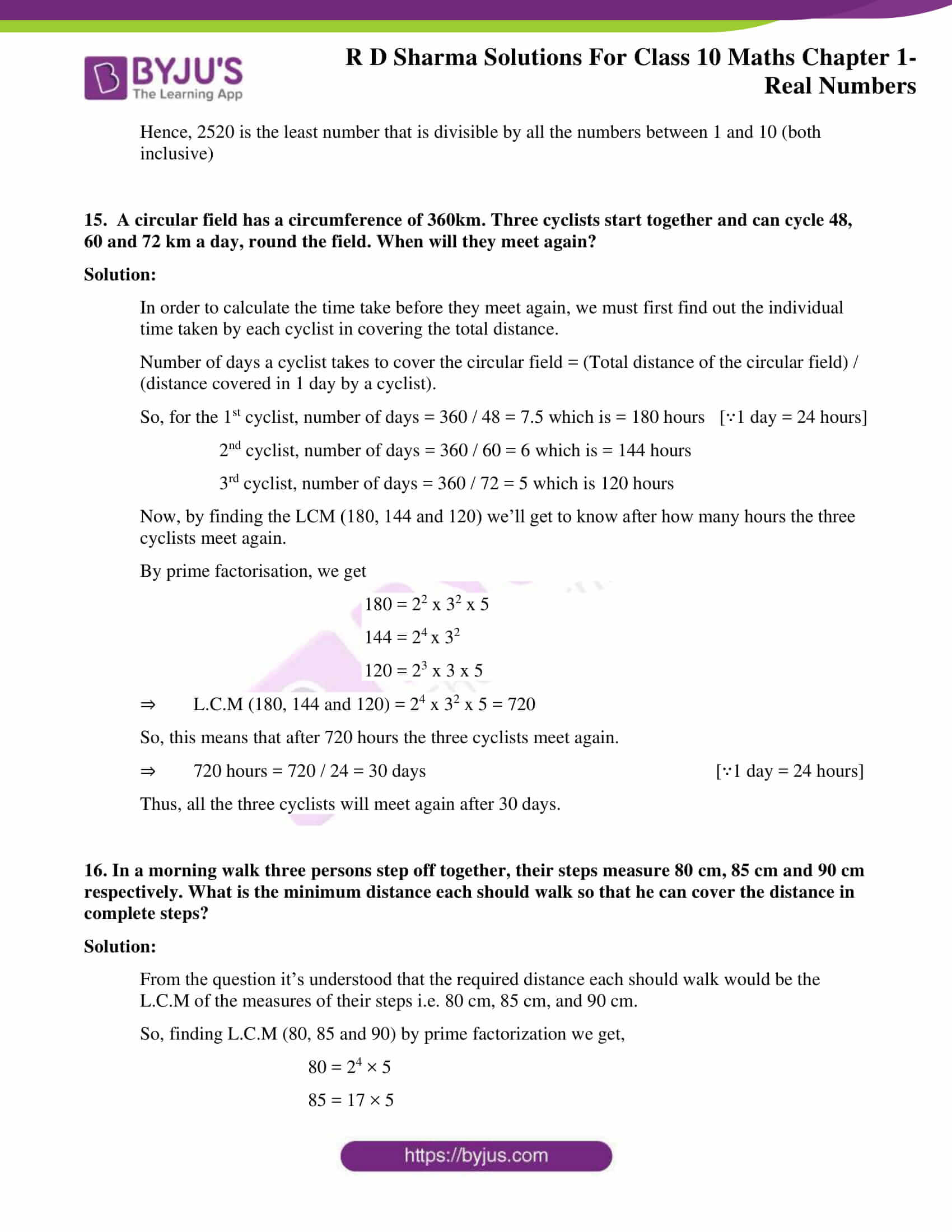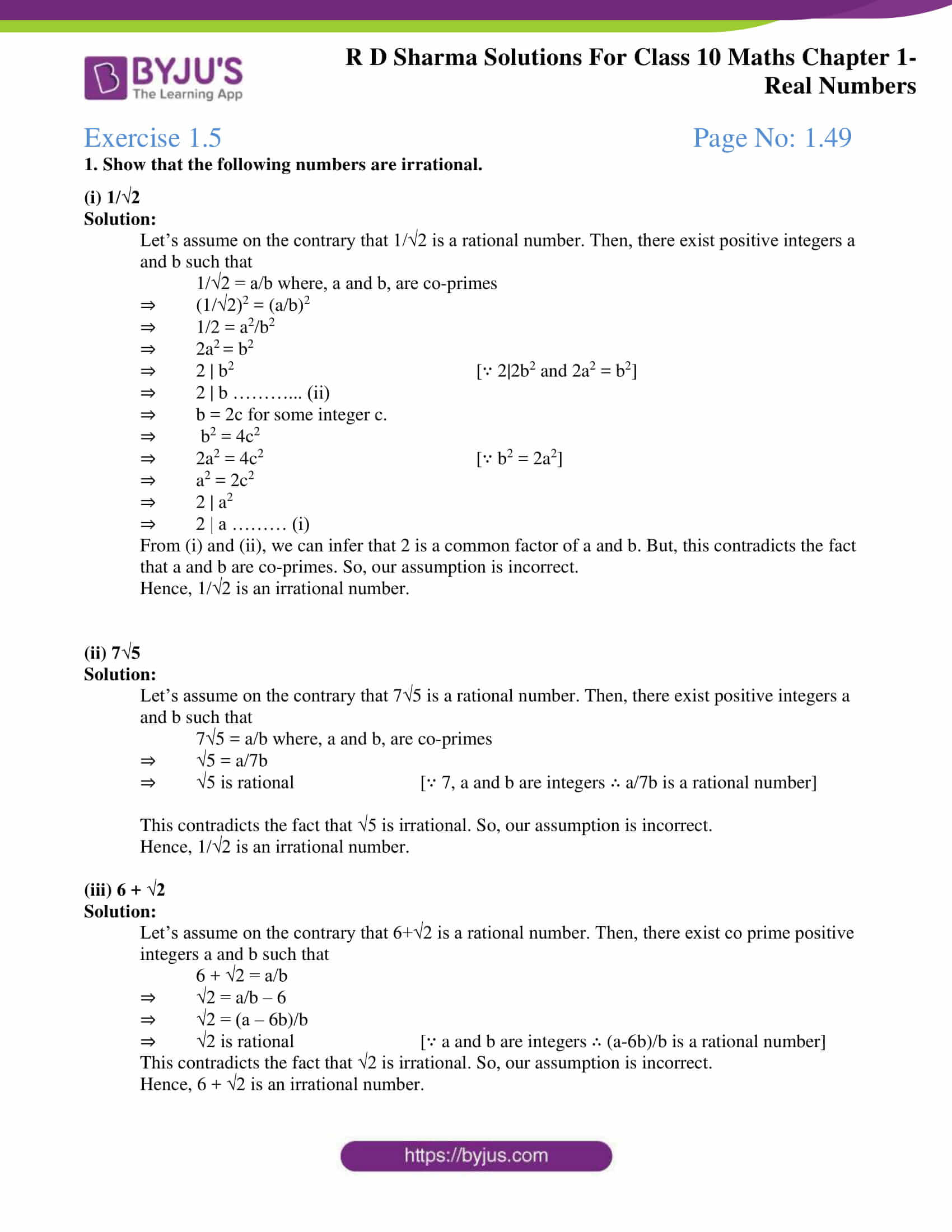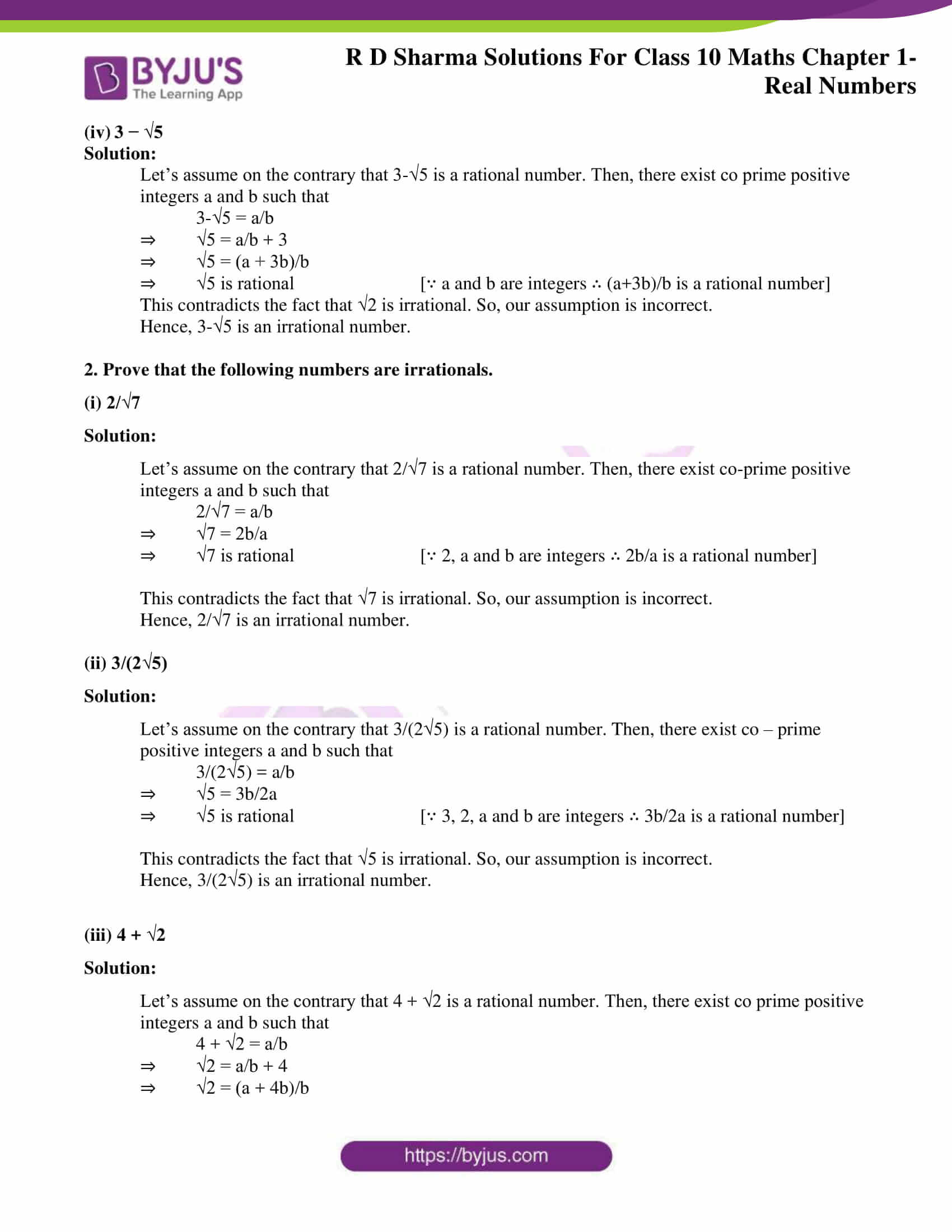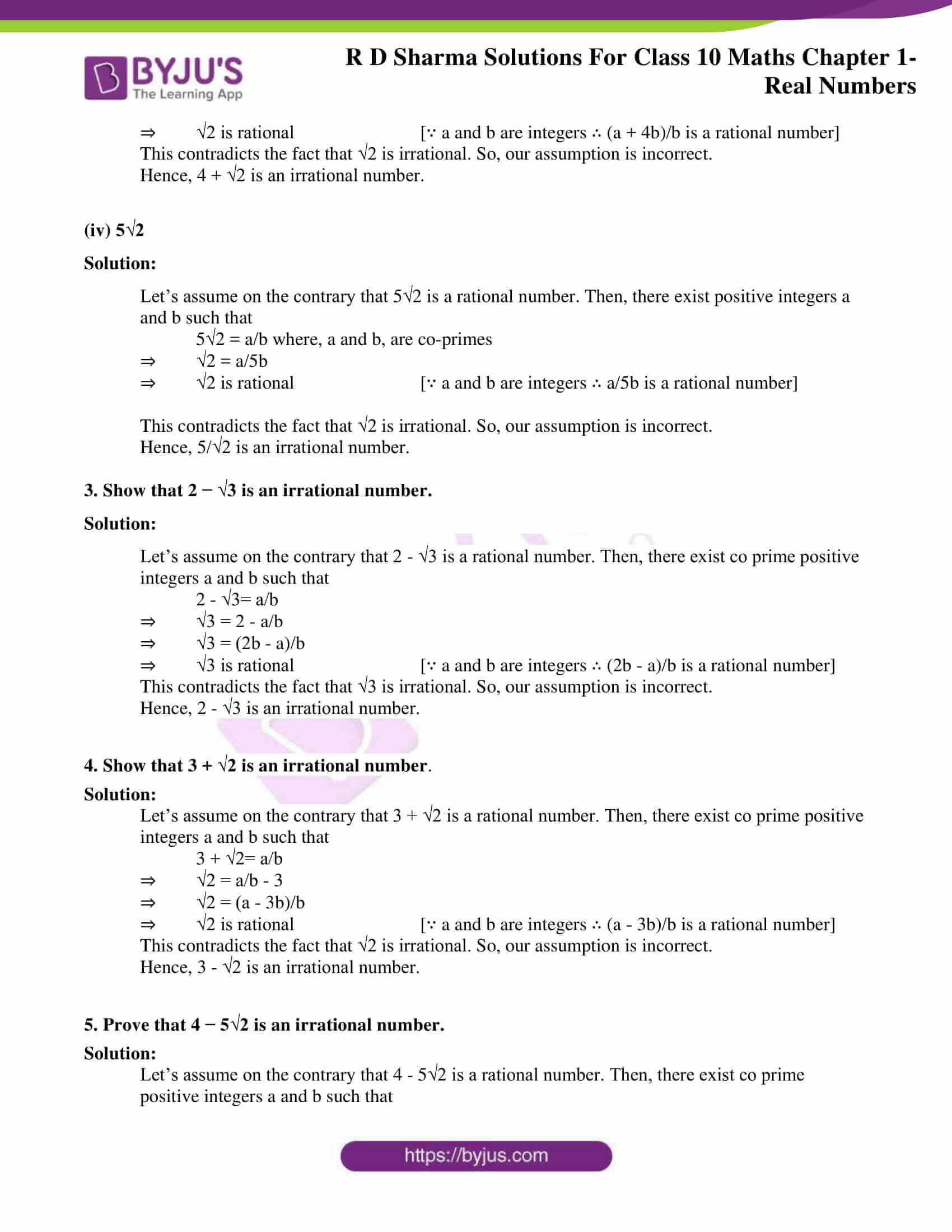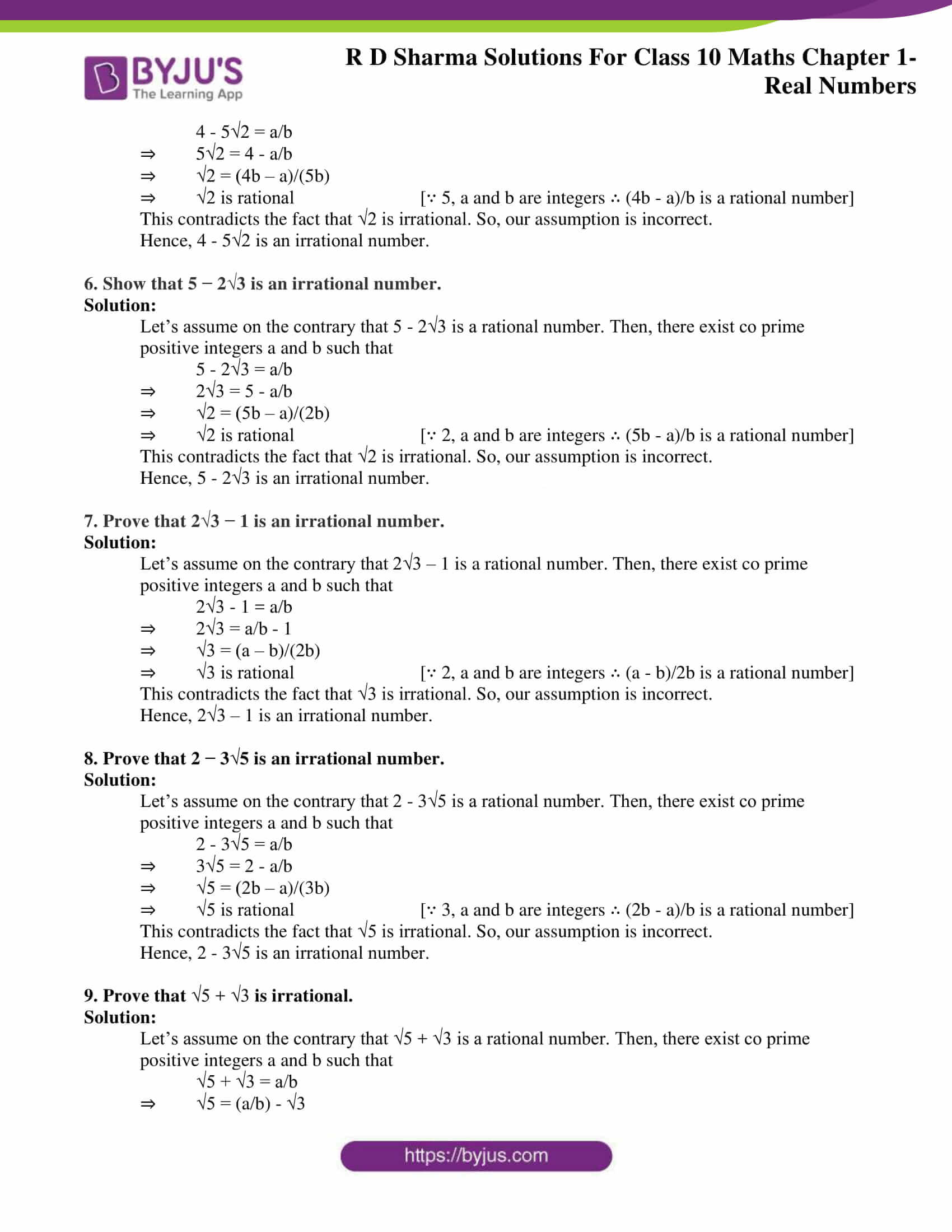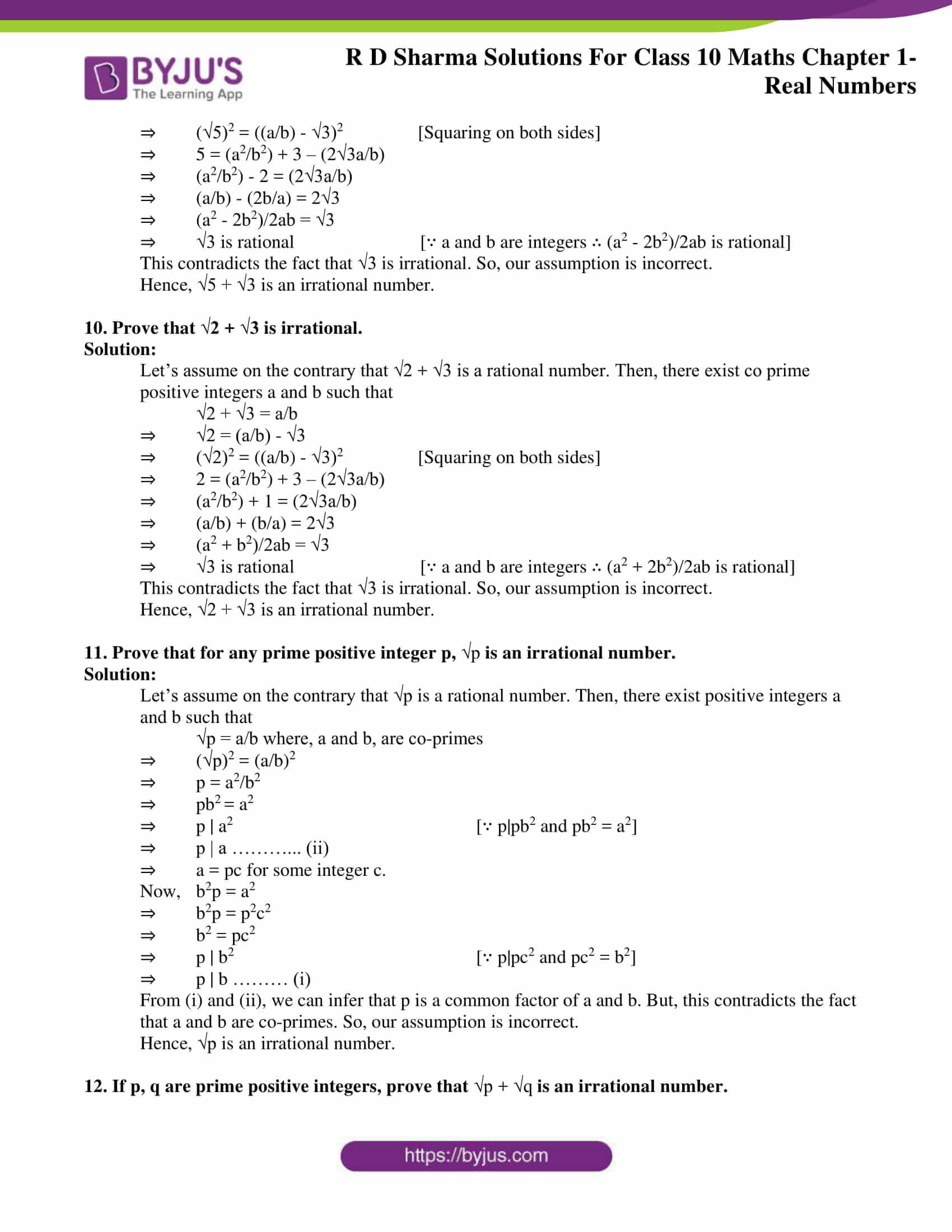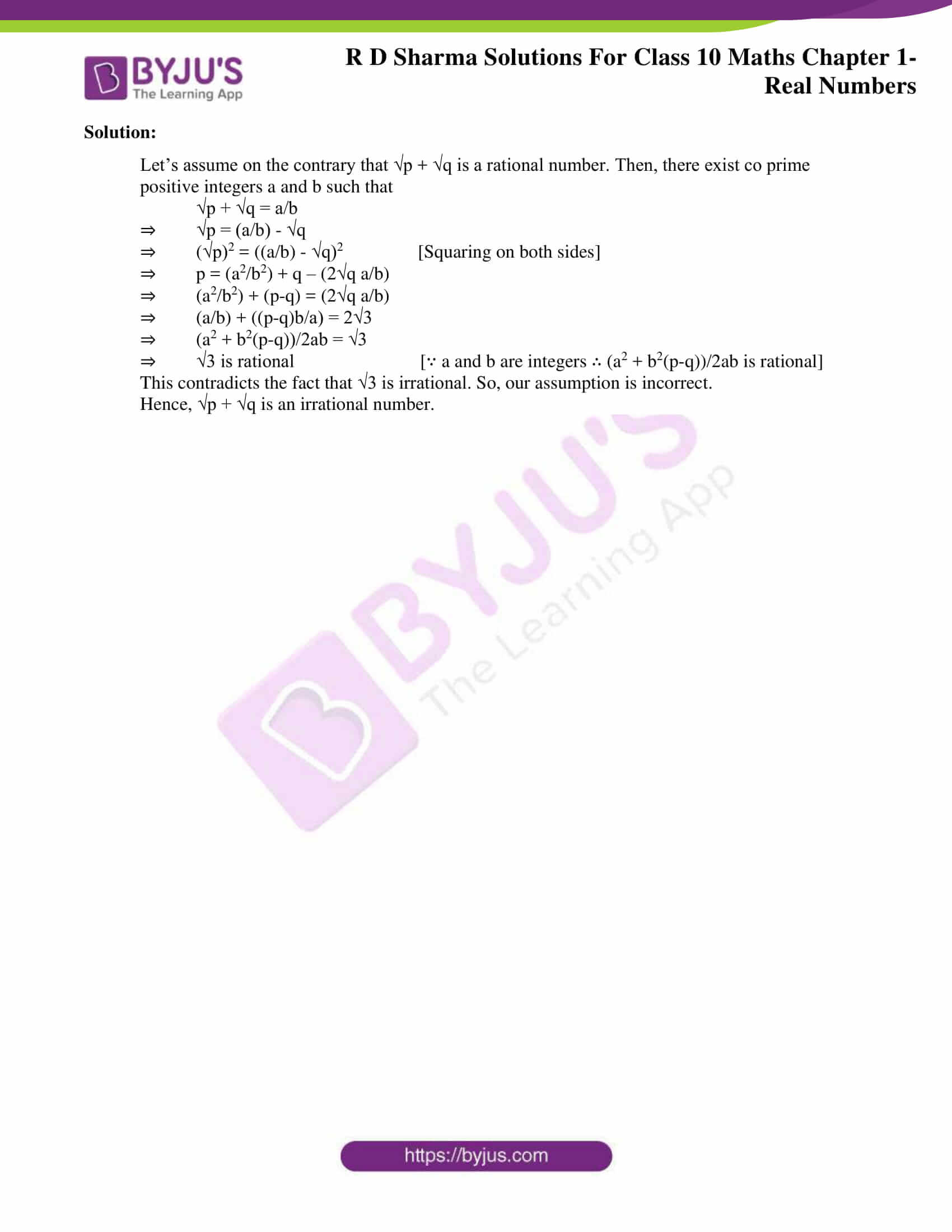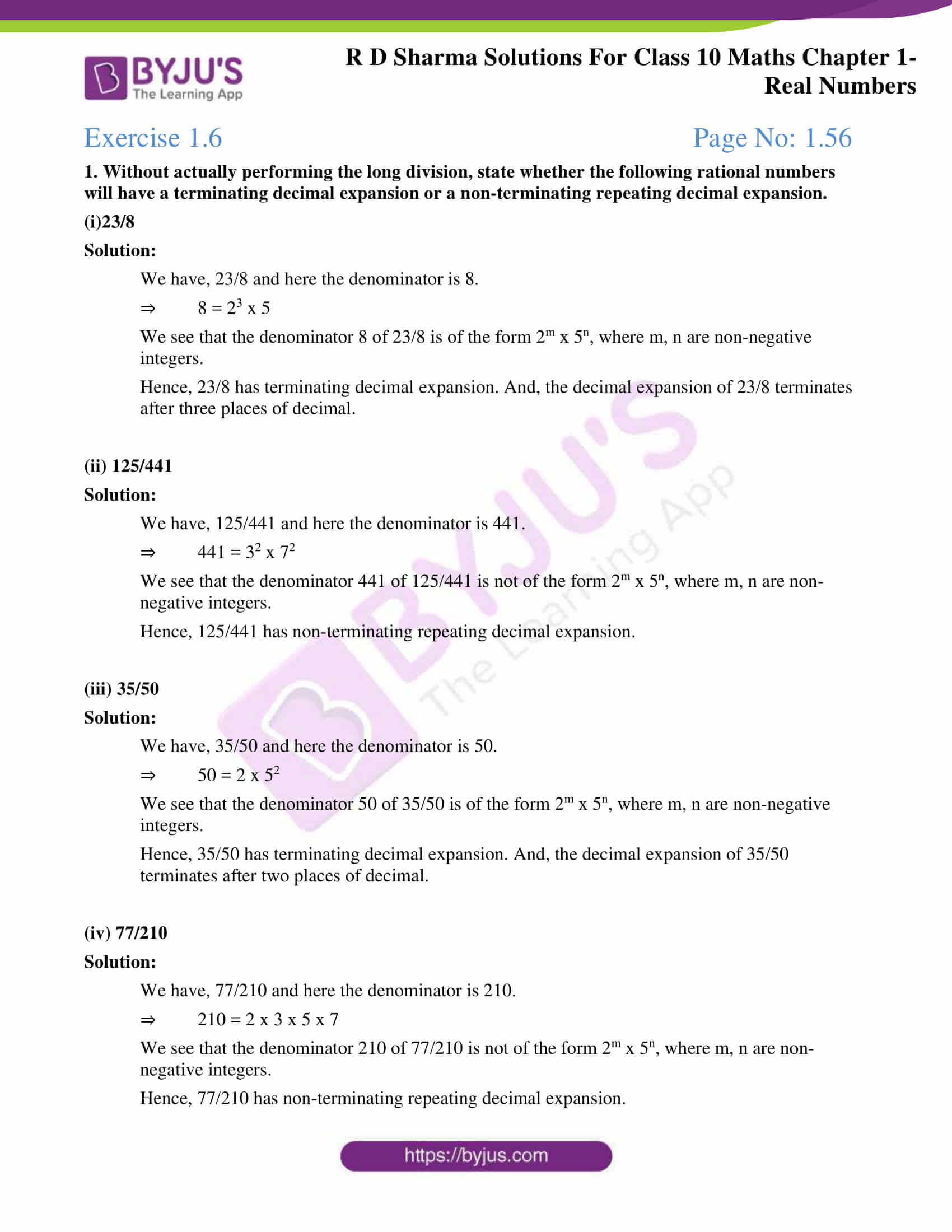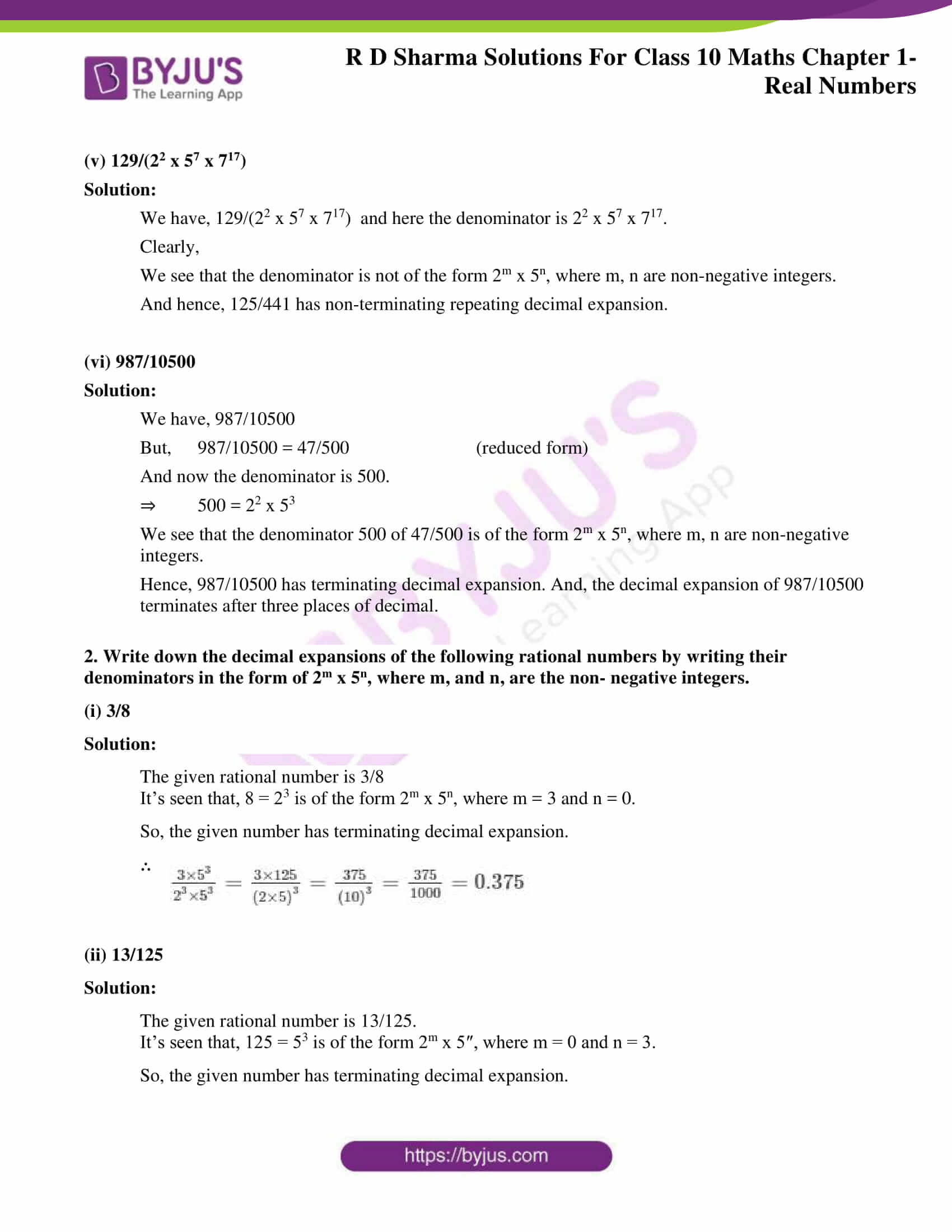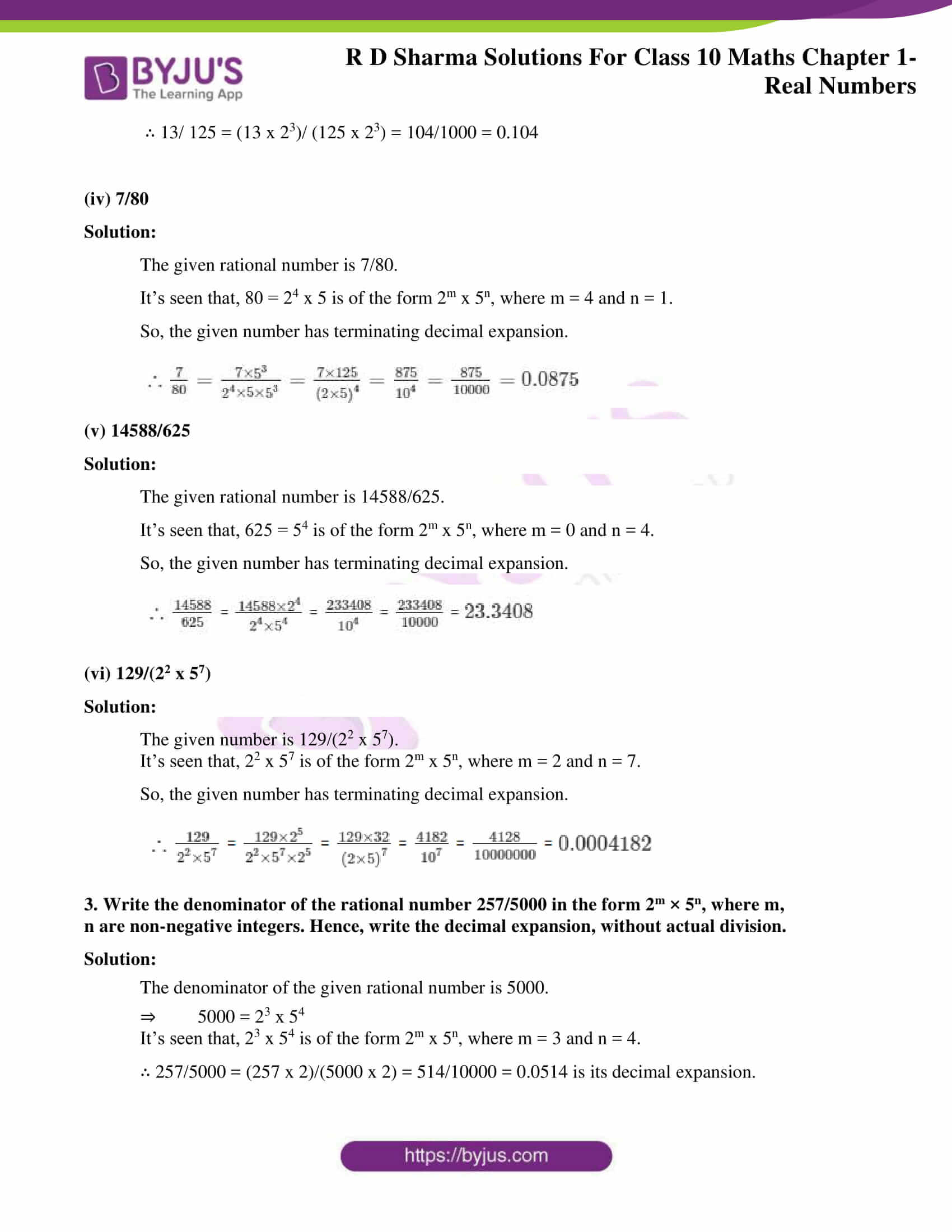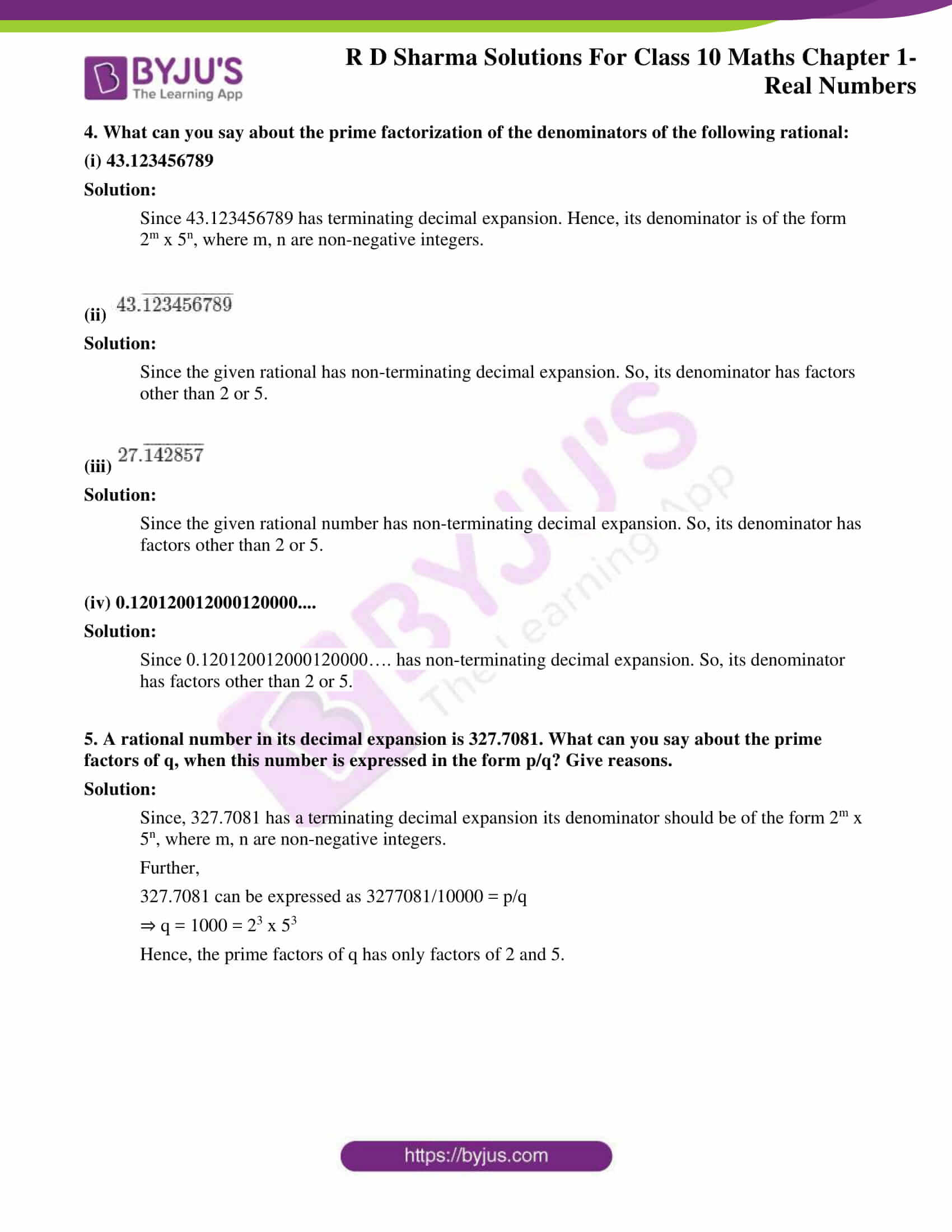Chapter 1- Real Numbers contains six exercises for which RD Sharma Solutions for Class 10 provides easy and precise answers. The objective is to help students get the correct understanding of the problems by introducing the right methods to solve them. In this chapter, the students will comprehend the divisibility of integers and some important properties of integers like:

• Eculid’s division Lemma
• Euclid’s division algorithm
• Fundamental Theorem of Arithmetic

### Access the RD Sharma Solutions For Class 10 Chapter 1 – Real Numbers

Exercise 1.1 Page No: 1.10

1. If a and b are two odd positive integers such that a > b, then prove that one of the two numbers (a+b)/2 and (a-b)/2 is odd and the other is even.

Solution:

We know that any odd positive integer is of the form 4q+1 or, 4q+3 for some whole number q.

Now that it’s given a > b

So, we can choose a= 4q+3 and b= 4q+1.

∴ (a+b)/2 = [(4q+3) + (4q+1)]/2

⇒ (a+b)/2 = (8q+4)/2

⇒ (a+b)/2 = 4q+2 = 2(2q+1) which is clearly an even number.

Now, doing (a-b)/2

⇒ (a-b)/2 = [(4q+3)-(4q+1)]/2

⇒ (a-b)/2 = (4q+3-4q-1)/2

⇒ (a-b)/2 = (2)/2

⇒ (a-b)/2 = 1 which is an odd number.

Hence, one of the two numbers (a+b)/2 and (a-b)/2 is odd and the other is even.

2. Prove that the product of two consecutive positive integers is divisible by 2.

Solution:

Let’s consider two consecutive positive integers as (n-1) and n.

∴ Their product = (n-1) n

= n2 – n

And then we know that any positive integer is of the form 2q or 2q+1. (From Euclid’s division lemma for b= 2)

So, when n= 2q

We have,

⇒ n2 – n = (2q)2 – 2q

⇒ n2 – n = 4q2 -2q

⇒ n2 – n = 2(2q2 -q)

Thus, n2 – n is divisible by 2.

Now, when n= 2q+1

We have,

⇒ n2 – n = (2q+1)2 – (2q-1)

⇒ n2 – n = (4q2+4q+1 – 2q+1)

⇒ n2 – n = (4q2+2q+2)

⇒ n2 – n = 2(2q2+q+1)

Thus, n2 – n is divisible by 2 again.

Hence, the product of two consecutive positive integers is divisible by 2.

3. Prove that the product of three consecutive positive integers is divisible by 6.

Solution:

Let n be any positive integer.

Thus, the three consecutive positive integers are n, n+1 and n+2.

We know that any positive integer can be of the form 6q, or 6q+1, or 6q+2, or 6q+3, or 6q+4, or 6q+5. (From Euclid’s division lemma for b= 6)

So,

For n= 6q,

⇒ n(n+1)(n+2)= 6q(6q+1)(6q+2)

⇒ n(n+1)(n+2)= 6[q(6q+1)(6q+2)]

⇒ n(n+1)(n+2)= 6m, which is divisible by 6. [m= q(6q+1)(6q+2)]

For n= 6q+1,

⇒ n(n+1)(n+2)= (6q+1)(6q+2)(6q+3)

⇒ n(n+1)(n+2)= 6[(6q+1)(3q+1)(2q+1)]

⇒ n(n+1)(n+2)= 6m, which is divisible by 6. [m= (6q+1)(3q+1)(2q+1)]

For n= 6q+2,

⇒ n(n+1)(n+2)= (6q+2)(6q+3)(6q+4)

⇒ n(n+1)(n+2)= 6[(3q+1)(2q+1)(6q+4)]

⇒ n(n+1)(n+2)= 6m, which is divisible by 6. [m= (3q+1)(2q+1)(6q+4)]

For n= 6q+3,

⇒ n(n+1)(n+2)= (6q+3)(6q+4)(6q+5)

⇒ n(n+1)(n+2)= 6[(2q+1)(3q+2)(6q+5)]

⇒ n(n+1)(n+2)= 6m, which is divisible by 6. [m= (2q+1)(3q+2)(6q+5)]

For n= 6q+4,

⇒ n(n+1)(n+2)= (6q+4)(6q+5)(6q+6)

⇒ n(n+1)(n+2)= 6[(3q+2)(3q+1)(2q+2)]

⇒ n(n+1)(n+2)= 6m, which is divisible by 6. [m= (3q+2)(3q+1)(2q+2)]

For n= 6q+5,

⇒ n(n+1)(n+2)= (6q+5)(6q+6)(6q+7)

⇒ n(n+1)(n+2)= 6[(6q+5)(q+1)(6q+7)]

⇒ n(n+1)(n+2)= 6m, which is divisible by 6. [m= (6q+5)(q+1)(6q+7)]

Hence, the product of three consecutive positive integers is divisible by 6.

4. For any positive integer n, prove that n3 – n divisible by 6.

Solution:

Let, n be any positive integer. And since any positive integer can be of the form 6q, or 6q+1, or 6q+2, or 6q+3, or 6q+4, or 6q+5. (From Euclid’s division lemma for b= 6)

We have n3 – n = n(n2-1)= (n-1)n(n+1),

For n= 6q,

⇒ (n-1)n(n+1)= (6q-1)(6q)(6q+1)

⇒ (n-1)n(n+1)= 6[(6q-1)q(6q+1)]

⇒ (n-1)n(n+1)= 6m, which is divisible by 6. [m= (6q-1)q(6q+1)]

For n= 6q+1,

⇒ (n-1)n(n+1)= (6q)(6q+1)(6q+2)

⇒ (n-1)n(n+1)= 6[q(6q+1)(6q+2)]

⇒ (n-1)n(n+1)= 6m, which is divisible by 6. [m= q(6q+1)(6q+2)]

For n= 6q+2,

⇒ (n-1)n(n+1)= (6q+1)(6q+2)(6q+3)

⇒ (n-1)n(n+1)= 6[(6q+1)(3q+1)(2q+1)]

⇒ (n-1)n(n+1)= 6m, which is divisible by 6. [m= (6q+1)(3q+1)(2q+1)]

For n= 6q+3,

⇒ (n-1)n(n+1)= (6q+2)(6q+3)(6q+4)

⇒ (n-1)n(n+1)= 6[(3q+1)(2q+1)(6q+4)]

⇒ (n-1)n(n+1)= 6m, which is divisible by 6. [m= (3q+1)(2q+1)(6q+4)]

For n= 6q+4,

⇒ (n-1)n(n+1)= (6q+3)(6q+4)(6q+5)

⇒ (n-1)n(n+1)= 6[(2q+1)(3q+2)(6q+5)]

⇒ (n-1)n(n+1)= 6m, which is divisible by 6. [m= (2q+1)(3q+2)(6q+5)]

For n= 6q+5,

⇒ (n-1)n(n+1)= (6q+4)(6q+5)(6q+6)

⇒ (n-1)n(n+1)= 6[(6q+4)(6q+5)(q+1)]

⇒ (n-1)n(n+1)= 6m, which is divisible by 6. [m= (6q+4)(6q+5)(q+1)]

Hence, for any positive integer n, n3 – n is divisible by 6.

5.  Prove that if a positive integer is of form 6q + 5, then it is of the form 3q + 2 for some integer q, but not conversely.

Solution:

Let n= 6q+5 be a positive integer for some integer q.

We know that any positive integer can be of the form 3k, or 3k+1, or 3k+2. (From Euclid’s division lemma for b= 3)

∴ q can be 3k or, 3k+1 or, 3k+2. (From Euclid’s division lemma for b= 3)

If q= 3k, then

⇒ n= 6q+5

⇒ n= 6(3k)+5

⇒ n= 18q+5 = (18q+3)+ 2

⇒ n= 3(6q+1)+2

⇒ n= 3m+2, where m is some integer

If q= 3k+1, then

⇒ n= 6q+5

⇒ n= 6(3k+1)+5

⇒ n= 18q+6+5 = (18q+9)+ 2

⇒ n= 3(6q+3)+2

⇒ n= 3m+2, where m is some integer

If q= 3k+2, then

⇒ n= 6q+5

⇒ n= 6(3k+2)+5

⇒ n= 18q+12+5 = (18q+15)+ 2

⇒ n= 3(6q+5)+2

⇒ n= 3m+2, where m is some integer

Hence, if a positive integer is of form 6q + 5, then it is of the form 3q + 2 for some integer q.

Conversely,

Let n= 3q+2

And we know that a positive integer can be of the form 6k, or 6k+1, or 6k+2, or 6k+3, or 6k+4, or 6k+5. (From Euclid’s division lemma for b= 6)

So, now if q=6k then

⇒ n= 3q+2

⇒ n= 3(6k)+2

⇒ n= 6(3k)+2

⇒ n= 6m+2, where m is some integer

Now, this is not of the form 6q + 5.

Therefore, if n is of the form 3q + 2, then is necessary won’t be of the form 6q + 5.

6.  Prove that square of any positive integer of the form 5q + 1 is of the same form.

Solution:

Here, the integer ‘n’ is of the form 5q+1.

⇒ n= 5q+1

On squaring it,

⇒ n2= (5q+1)2

⇒ n2= (25q2+10q+1)

⇒ n2= 5(5q2+2q)+1

⇒ n2= 5m+1, where m is some integer. [For m = 5q2+2q]

Therefore, the square of any positive integer of the form 5q + 1 is of the same form.

7. Prove that the square of any positive integer is of the form 3m or 3m + 1 but not of the form 3m + 2.

Solution:

Let any positive integer ‘n’ be of the form 3q or, 3q+1 or 3q+2. (From Euclid’s division lemma for b= 3)

If n= 3q,

Then, on squaring

⇒ n2= (3q)2 = 9q2

⇒ n2= 3(3q2)

⇒ n2= 3m, where m is some integer [m = 3q2]

If n= 3q+1,

Then, on squaring

⇒ n2= (3q+1)2 = 9q2 + 6q + 1

⇒ n2= 3(3q2 +2q) + 1

⇒ n2= 3m + 1, where m is some integer [m = 3q2 +2q]

If n= 3q+2,

Then, on squaring

⇒ n2= (3q+2)2 = 9q2 + 12q + 4

⇒ n2= 3(3q2 + 4q + 1) + 1

⇒ n2= 3m, where m is some integer [m = 3q2 + 4q + 1]

Thus, it is observed that the square of any positive integer is of the form 3m or 3m + 1 but not of the form 3m + 2.

8.  Prove that the square of any positive integer is of the form 4q or 4q + 1 for some integer q.

Solution:

Let ‘a’ be any positive integer.

Then,

According to Euclid’s division lemma,

a=bq+r

According to the question, when b = 4.

a = 4k + r, 0 < r < 4

When r = 0, we get, a = 4k

a2 = 16k2 = 4(4k2) = 4q, where q = 4k2

When r = 1, we get, a = 4k + 1

a2 = (4k + 1)2 = 16k2 + 1 + 8k = 4(4k + 2) + 1 = 4q + 1, where q = k(4k + 2)

When r = 2, we get, a = 4k + 2

a2 = (4k + 2)2 = 16k2 + 4 + 16k = 4(4k2 + 4k + 1) = 4q, where q = 4k2 + 4k + 1

When r = 3, we get, a = 4k + 3

a2 = (4k + 3)2 = 16k2 + 9 + 24k = 4(4k2 + 6k + 2) + 1

= 4q + 1, where q = 4k2 + 6k + 2

Therefore, the square of any positive integer is either of the form 4q or 4q + 1 for some integer q.

9. Prove that the square of any positive integer is of the form 5q or 5q + 1, 5q + 4 for some integer q.

Solution:

Let ‘a’ be any positive integer.

Then,

According to Euclid’s division lemma,

a = bq+r

According to the question, when b = 5.

a = 5k + r, 0 < r < 5

When r = 0, we get, a = 5k

a2 = 25k2 = 5(5k2) = 5q, where q = 5k2

When r = 1, we get, a = 5k + 1

a2 = (5k + 1)2 = 25k2 + 1 + 10k = 5k(5k + 2) + 1 = 5q + 1, where q = k(5k + 2)

When r = 2, we get, a = 5k + 2

a2 = (5k + 2)2 = 25k2 + 4 + 20k = 5(5k2 + 4k) + 4 = 4q + 4, where q = 5k2 + 4k

When r = 3, we get, a = 5k + 3

a2 = (5k + 3)2 = 25k2 + 9 + 30k = 5(5k2 + 6k + 1) + 4

= 5q + 4, where q = 5k2 + 6k + 1

When r = 4, we get, a = 5k + 4

a2 = (5k + 4)2 = 25k2 + 16 + 40k = 5(5k2 + 8k + 3) + 1

= 5q + 1, where q = 5k2 + 8k + 3

Therefore, the square of any positive integer is of the form 5q or, 5q + 1 or 5q + 4 for some integer q.

10. Show that the square of odd integer is of the form 8q + 1, for some integer q.

Solution:

From Euclid’s division lemma,

a = bq+r ; where 0 < r < b

Putting b=4 for the question,

⇒ a = 4q + r, 0 < r < 4

For r = 0, we get a = 4q, which is an even number.

For r = 1, we get a = 4q + 1, which is an odd number.

On squaring,

⇒ a2 = (4q + 1)2 = 16q2 + 1 + 8q = 8(2q2 + q) + 1 = 8m + 1, where m = 2q2 + q

For r = 2, we get a = 4q + 2 = 2(2q + 1), which is an even number.

For r = 3, we get a = 4q + 3, which is an odd number.

On squaring,

⇒ a2 = (4q + 3)2 = 16q2 + 9 + 24q = 8(2q2 + 3q + 1) + 1

= 8m + 1, where m = 2q2 + 3q + 1

Thus, the square of an odd integer is of the form 8q + 1, for some integer q.

11. Show that any positive odd integer is of the form 6q +1 or 6q + 3 or 6q + 5, where q is some integer.

Solution:

Let ‘a’ be any positive integer.

Then from Euclid’s division lemma,

a = bq+r ; where 0 < r < b

Putting b=6 we get,

⇒ a = 6q + r, 0 < r < 6

For r = 0, we get a = 6q = 2(3q) = 2m, which is an even number. [m = 3q]

For r = 1, we get a = 6q + 1 = 2(3q) + 1 = 2m + 1, which is an odd number. [m = 3q]

For r = 2, we get a = 6q + 2 = 2(3q + 1) = 2m, which is an even number. [m = 3q + 1]

For r = 3, we get a = 6q + 3 = 2(3q + 1) + 1 = 2m + 1, which is an odd number. [m = 3q + 1]

For r = 4, we get a = 6q + 4 = 2(3q + 2) + 1 = 2m + 1, which is an even number. [m = 3q + 2]

For r = 5, we get a = 6q + 5 = 2(3q + 2) + 1 = 2m + 1, which is an odd number. [m = 3q + 2]

Thus, from the above it can be seen that any positive odd integer can be of the form 6q +1 or 6q + 3 or 6q + 5, where q is some integer.

12. Show that the square of any positive integer cannot be of the form 6m + 2 or 6m + 5 for any integer m.

Solution:

Let the positive integer = a

According to Euclid’s division algorithm,

a = 6q + r, where 0 ≤ r < 6

a2 = (6q + r)2 = 36q2 + r+ 12qr [∵(a+b)2 = a2 + 2ab + b2]

a2 = 6(6q2 + 2qr) + r2   …(i), where,0 ≤ r < 6

When r = 0, substituting r = 0 in Eq.(i), we get

a= 6 (6q2) = 6m, where, m = 6q2 is an integer.

When r = 1, substituting r = 1 in Eq.(i), we get

a2 + 6 (6q2 + 2q) + 1 = 6m + 1, where, m = (6q2 + 2q) is an integer.

When r = 2, substituting r = 2 in Eq(i), we get

a2 = 6(6q2 + 4q) + 4 = 6m + 4, where, m = (6q2 + 4q) is an integer.

When r = 3, substituting r = 3 in Eq.(i), we get

a2 = 6(6q2 + 6q) + 9 = 6(6q2 + 6a) + 6 + 3

a2 = 6(6q2 + 6q + 1) + 3 = 6m + 3, where, m = (6q + 6q + 1) is integer.

When r = 4, substituting r = 4 in Eq.(i) we get

a2 = 6(6q2 + 8q) + 16

= 6(6q2 + 8q) + 12 + 4

⇒ a= 6(6q+ 8q + 2) + 4 = 6m + 4, where, m = (6q2 + 8q + 2) is integer.

When r = 5, substituting r = 5 in Eq.(i), we get

a= 6 (6q2 + 10q) + 25 = 6(6q+ 10q) + 24 + 1

a2 = 6(6q2 + 10q + 4) + 1 = 6m + 1, where, m = (6q2 + 10q + 1) is integer.

Hence, the square of any positive integer cannot be of the form 6m + 2 or 6m + 5 for any integer m.

Hence Proved.

13. Show that the cube of a positive integer of the form 6q + r, q is an integer and r = 0, 1, 2, 3, 4, 5 is also of the form 6m + r.

Solution:

Given, 6q + r is a positive integer, where q is an integer and r = 0, 1, 2, 3, 4, 5

Then, the positive integers are of the form 6q, 6q+1, 6q+2, 6q+3, 6q+4 and 6q+5.

Taking cube on L.H.S and R.H.S,

For 6q,

(6q)3 = 216 q3 = 6(36q)3 + 0

= 6m + 0, (where m is an integer = (36q)3)

For 6q+1,

(6q+1)3 = 216q3 + 108q2 + 18q + 1

= 6(36q3 + 18q2 + 3q) + 1

= 6m + 1, (where m is an integer = 36q3 + 18q2 + 3q)

For 6q+2,

(6q+2)3 = 216q3 + 216q2 + 72q + 8

= 6(36q3 + 36q2 + 12q + 1) +2

= 6m + 2, (where m is an integer = 36q3 + 36q2 + 12q + 1)

For 6q+3,

(6q+3)3 = 216q3 + 324q2 + 162q + 27

= 6(36q3 + 54q2 + 27q + 4) + 3

= 6m + 3, (where m is an integer = 36q3 + 54q2 + 27q + 4)

For 6q+4,

(6q+4)3 = 216q3 + 432q2 + 288q + 64

= 6(36q3 + 72q2 + 48q + 10) + 4

= 6m + 4, (where m is an integer = 36q3 + 72q2 + 48q + 10)

For 6q+5,

(6q+5)3 = 216q3 + 540q2 + 450q + 125

= 6(36q3 + 90q2 + 75q + 20) + 5

= 6m + 5, (where m is an integer = 36q3 + 90q2 + 75q + 20)

Hence, the cube of a positive integer of the form 6q + r, q is an integer and r = 0, 1, 2, 3, 4, 5 is also of the form 6m + r.

14. Show that one and only one out of n, n + 4, n + 8, n + 12 and n + 16 is divisible by 5, where n is any positive integer.

Solution:

According to Euclid’s division Lemma,

Let the positive integer = n

And, b=5

n = 5q+r, where q is the quotient and r is the remainder

0 < r < 5 implies remainders may be 0, 1, 2, 3, 4 and 5

Therefore, n may be in the form of 5q, 5q+1, 5q+2, 5q+3, 5q+4

So, this gives us the following cases:

CASE 1:

When, n = 5q

n+4 = 5q+4

n+8 = 5q+8

n+12 = 5q+12

n+16 = 5q+16

Here, n is only divisible by 5

CASE 2:

When, n = 5q+1

n+4 = 5q+5 = 5(q+1)

n+8 = 5q+9

n+12 = 5q+13

n+16 = 5q+17

Here, n + 4 is only divisible by 5

CASE 3:

When, n = 5q+2

n+4 = 5q+6

n+8 = 5q+10 = 5(q+2)

n+12 = 5q+14

n+16 = 5q+18

Here, n + 8 is only divisible by 5

CASE 4:

When, n = 5q+3

n+4 = 5q+7

n+8 = 5q+11

n+12 = 5q+15 = 5(q+3)

n+16 = 5q+19

Here, n + 12 is only divisible by 5

CASE 5:

When, n = 5q+4

n+4 = 5q+8

n+8 = 5q+12

n+12 = 5q+16

n+16 = 5q+20 = 5(q+4)

Here, n + 16 is only divisible by 5

So, we can conclude that one and only one out of n, n + 4, n + 8, n + 12 and n + 16 is divisible by 5.

Hence Proved

15. Show that the square of an odd integer can be of the form 6q + 1 or 6q + 3, for some integer q.

Solution:

Let ‘a’ be an odd integer and b = 6.

According to Euclid’s algorithm,

a = 6m + r for some integer m ≥ 0

And r = 0, 1, 2, 3, 4, 5 because 0 ≤ r < 6.

So, we have that,

a = 6m or, 6m + 1 or, 6m + 2 or, 6m + 3 or, 6m + 4 or 6m + 5

Thus, we are choosing for a = 6m + 1 or, 6m + 3 or 6m + 5 for it to be an odd integer.

For a = 6m + 1,

(6m + 1)2 = 36m2 + 12m + 1

= 6(6m2 + 2m) + 1

= 6q + 1, where q is some integer and q = 6m2 + 2m.

For a = 6m + 3

(6m + 3)2 = 36m2 + 36m + 9

= 6(6m2 + 6m + 1) + 3

= 6q + 3, where q is some integer and q = 6m2 + 6m + 1

For a = 6m + 5,

(6m + 5)2 = 36m2 + 60m + 25

= 6(6m2 + 5m + 4) + 1

= 6q + 1, where q is some integer and q = 6m2 + 5m + 4.

Therefore, the square of an odd integer is of the form 6q + 1 or 6q + 3, for some integer q.

Hence Proved.

16. A positive integer is of the form 3q + 1, q being a natural number. Can you write its square in any form other than 3m + 1, 3m or 3m + 2 for some integer m? Justify your answer.

Solution:

No.

Justification:

By Euclid’s Division Lemma,

a = bq + r, 0 ≤ r < b

Here, a is any positive integer and b = 3,

⇒ a = 3q + r

So, a can be of the form 3q, 3q + 1 or 3q + 2.

Now, for a = 3q

(3q)2 = 9q2 = 3m [where m = 3q2]

for a = 3q + 1

(3q + 1)2 = 9q2 + 6q + 1 = 3(3q2 + 2q) + 1 = 3m + 1 [where m = 3q2 + 2q]

for a = 3q + 2

(3q + 2)2 = 9q2 + 12q + 4 = 9q2 + 12q + 3 + 1 = 3(3q2 + 4q + 1) + 1

= 3m + 1 [where m = 3q2 + 4q + 1]

Thus, square of a positive integer of the form 3q + 1 is always of the form 3m + 1 or 3m for some integer m.

17. Show that the square of any positive integer cannot be of the form 3m + 2, where m is a natural number.

Solution:

Let the positive integer be ‘a’

According to Euclid’s division lemma,

a = bm + r

According to the question, we take b = 3

a = 3m + r

So, r = 0, 1, 2.

When r = 0, a = 3m.

When r = 1, a = 3m + 1.

When r = 2, a = 3m + 2.

Now,

When a = 3m

a2 = (3m)2 = 9m2

a2 = 3(3m2) = 3q, where q = 3m2

When a = 3m + 1

a2 = (3m + 1)2 = 9m2 + 6m + 1

a2 = 3(3m2 + 2m) + 1 = 3q + 1, where q = 3m2 + 2m

When a = 3m + 2

a2 = (3m + 2)2

a2 = 9m2 + 12m + 4

a2 = 3(3m2 + 4m + 1) + 1

a2 = 3q + 1 where q = 3m2 + 4m + 1

Therefore, square of any positive integer cannot be of the form 3q + 2, where q is a natural number.

Hence Proved.

Exercise 1.2 Page No: 1.27

1. Define HCF of two positive integers and find the HCF of the following pairs of numbers:

(i) 32 and 54

Solution:

Now, apply Euclid’s Division Lemma on 54 and 32

54 = 32 x 1 + 22

Since remainder ≠ 0, apply division lemma on 32 and remainder 22

32 = 22 x 1 + 10

Since remainder ≠ 0, apply division lemma on 22 and remainder 10

22 = 10 x 2 + 2

Since remainder ≠ 0, apply division lemma on 10 and 2

10 = 2 x 5 + 0

Therefore, the H.C.F. of 32 and 54 is 2

(ii) 18 and 24

Solution:

Now, apply Euclid’s Division Lemma on 24 and 18

24 = 18 x 1 + 6.

Since remainder ≠ 0, apply division lemma on divisor 18 and remainder 6

18 = 6 x 3 + 0.

Therefore, H.C.F. of 18 and 24 is 6

(iii) 70 and 30

Solution:

Now, apply Euclid’s Division lemma on 70 and 30

70 = 30 x 2 + 10.

Since remainder ≠ 0, apply division lemma on divisor 30 and remainder 10

30 = 10 x 3 + 0.

Therefore, H.C.F. of 70 and 30 is 10

(iv) 56 and 88

Solution:

Now, apply Euclid’s Division lemma on 56 and 88

88 = 56 x 1 + 32.

Since remainder ≠ 0, apply division lemma on 56 and remainder 32

56 = 32 x 1 + 24.

Since remainder ≠ 0, apply division lemma on 32 and remainder 24

32 = 24 x 1+ 8.

Since remainder ≠ 0, apply division lemma on 24 and remainder 8

24 = 8 x 3 + 0.

Therefore, H.C.F. of 56 and 88 is 8

(v) 475 and 495

Solution:

By applying Euclid’s Division lemma on 495 and 475 we get,

495 = 475 x 1 + 20.

Since remainder ≠ 0, apply division lemma on 475 and remainder 20

475 = 20 x 23 + 15.

Since remainder ≠ 0, apply division lemma on 20 and remainder 15

20 = 15 x 1 + 5.

Since remainder ≠ 0, apply division lemma on 15 and remainder 5

15 = 5 x 3+ 0.

Therefore, H.C.F. of 475 and 495 is 5

(vi) 75 and 243

Solution:

By applying Euclid’s Division lemma on 243 and 75

243 = 75 x 3 + 18.

Since remainder ≠ 0, apply division lemma on 75 and remainder 18

75 = 18 x 4 + 3.

Since remainder ≠ 0, apply division lemma on divisor 18 and remainder 3

18 = 3 x 6+ 0.

Therefore, H.C.F. of 75 and 243 is 3

(vii) 240 and 6552

Solution:

By applying Euclid’s Division lemma on 6552 and 240 we get,

6552 = 240 x 27 + 72.

Since remainder ≠ 0, apply division lemma on divisor 240 and remainder 72

240 = 72 x 3+ 24.

Since remainder ≠ 0, apply division lemma on divisor 72 and remainder 24

72 = 24 x 3 + 0.

Therefore, H.C.F. of 240 and 6552 is 24

(viii) 155 and 1385

Solution:

By applying Euclid’s Division lemma on 1385 and 155 we get,

1385 = 155 x 8 + 145.

Since remainder ≠ 0, apply division lemma on divisor 155 and remainder 145.

155 = 145 x 1 + 10.

Since remainder ≠ 0 apply division lemma on divisor 145 and remainder 10

145 = 10 x 14 + 5.

Since remainder ≠ 0, apply division lemma on divisor 10 and remainder 5

10 = 5 x 2 + 0.

Therefore, H.C.F. of 155 and 1385 is 5

(ix) 100 and 190

Solution:

By applying Euclid’s division lemma on 190 and 100 we get,

190 = 100 x 1 + 90.

Since remainder ≠ 0, apply division lemma on divisor 100 and remainder 90

100 = 90 x 1 + 10.

Since remainder ≠ 0, apply division lemma on divisor 90 and remainder 10

90 = 10 x 9 + 0.

Therefore, H.C.F. of 100 and 190 is 10

(x) 105 and 120

Solution:

By applying Euclid’s division lemma on 120 and 105 we get,

120 = 105 x 1 + 15.

Since remainder ≠ 0, apply division lemma on divisor 105 and remainder 15

105 = 15 x 7 + 0.

Therefore, H.C.F. of 105 and 120 is 15

2. Use Euclid’s division algorithm to find the HCF of

(i) 135 and 225

Solution:

Given integers here are 225 and 135. On comparing, we find 225 > 135.

So, by applying Euclid’s division lemma to 225 and 135, we get

867 = 225 x 3 + 192

Since the remainder ≠ 0. So we apply the division lemma to the divisor 135 and remainder 90.

⇒ 135 = 90 x 1 + 45

Now we apply the division lemma to the new divisor 90 and remainder 45.

⇒ 90 = 45 x 2 + 0

Since the remainder at this stage is 0, the divisor will be the HCF.

Hence, the H.C.F of 225 and 135 is 45.

(ii) 196 and 38220

Solution:

Given integers here are 196 and 38220. On comparing, we find 38220 > 196.

So, by applying Euclid’s division lemma to 38220 and 196. We get,

38220 = 196 x 195 + 0

Since the remainder at this stage is 0, the divisor will be the HCF.

Hence, the HCF of 38220 and 196 is 196

(iii) 867 and 255

Solution:

Given integers here are 867 and 255. On comparing, we find 867 > 255.

So, by applying Euclid’s division lemma to 867 and 225, we get,

867 = 225 x 3 + 192

Since the remainder 192 ≠ 0. So we apply the division lemma to the divisor 225 and remainder 192. We get,

225 = 192 x 1 + 33

Now we apply the division lemma to the new divisor 192 and remainder 33. We get,

192 = 33 x 5 + 27

Now we apply the division lemma to the new divisor 33 and remainder 27. We get,

33 = 27 x 1 + 6

Now we apply the division lemma to the new divisor 27 and remainder 6. We get,

27 = 6 x 4 + 3

Now we apply the division lemma to the new divisor 27 and remainder 6. We get,

6 = 3 x 2 + 0

Since the remainder at this stage is 0, the divisor will be the HCF.

Hence, the HCF of 867 and 255 is 3.

(iv) 184, 230 and 276

Solution:

Let’s first choose 184 and 230 to find the HCF by using Euclid’s division lemma.

Thus, we obtain

230 = 184 x 1 + 46

Since the remainder 46 ≠ 0. So we apply the division lemma to the divisor 184 and remainder 46. We get,

184 = 46 x 4 + 0

The remainder at this stage is 0, the divisor will be the HCF i.e., 46 for 184 and 230.

Now, we again use Euclid’s division lemma to find the HCF of 46 and 276. And we get,

276 = 46 x 6 + 0

So, this stage has remainder 0. Thus, the HCF of the third number 276 and 46 is 46.

Hence, the HCF of 184, 230 and 276 is 46.

(v) 136, 170 and 255

Solution:

Let’s first choose 136 and 170 to find the HCF by using Euclid’s division lemma.

Thus, we obtain

170 = 136 x 1 + 34

Since the remainder 34 ≠ 0. So we apply the division lemma to the divisor 136 and remainder 34. We get,

136 = 34 x 4 + 0

The remainder at this stage is 0, the divisor will be the HCF i.e., 34 for 136 and 170.

Now, we again use Euclid’s division lemma to find the HCF of 34 and 255. And we get,

255 = 34 x 7 + 17

Since the remainder 17 ≠ 0. So we apply the division lemma to the divisor 34 and remainder 17. We get,

34 = 17 x 2 + 0

So, this stage has remainder 0. Thus, the HCF of the third number 255 and 34 is 17.

Hence, the HCF of 136, 170 and 255 is 17.

3. Find the HCF of the following pair of integers and express it as a linear combination of them,

(i) 963 and 657

Solution:

By applying Euclid’s division lemma on 963 and 657, we get

963 = 657 x 1 + 306………. (1)

As the remainder ≠ 0, apply division lemma on divisor 657 and remainder 306

657 = 306 x 2 + 45………… (2)

As the remainder ≠ 0, apply division lemma on divisor 306 and remainder 45

306 = 45 x 6 + 36…………. (3)

As the remainder ≠ 0, apply division lemma on divisor 45 and remainder 36

45 = 36 x 1 + 9…………… (4)

As the remainder ≠ 0, apply division lemma on divisor 36 and remainder 9

36 = 9 x 4 + 0……………. (5)

Thus, we can conclude the H.C.F. = 9.

Now, in order to express the found HCF as a linear combination of 963 and 657, we perform

9 = 45 – 36 x 1 [from (5)]

= 45 – [306 – 45 x 6] x 1 = 45 – 306 x 1 + 45 x 6 [from (3)]

= 45 x 7 – 306 x 1 = [657 -306 x 2] x 7 – 306 x 1 [from (2)]

= 657 x 7 – 306 x 14 – 306 x 1

= 657 x 7 – 306 x 15

= 657 x 7 – [963 – 657 x 1] x 15 [from (1)]

= 657 x 7 – 963 x 15 + 657 x 15

657 x 22 – 963 x 15.

(ii) 592 and 252

Solution:

By applying Euclid’s division lemma on 592 and 252, we get

592 = 252 x 2 + 88……… (1)

As the remainder ≠ 0, apply division lemma on divisor 252 and remainder 88

252 = 88 x 2 + 76………. (2)

As the remainder ≠ 0, apply division lemma on divisor 88 and remainder 76

88 = 76 x 1 + 12………… (3)

As the remainder ≠ 0, apply division lemma on divisor 76 and remainder 12

76 = 12 x 6 + 4………….. (4)

Since the remainder ≠ 0, apply division lemma on divisor 12 and remainder 4

12 = 4 x 3 + 0……………. (5)

Thus, we can conclude the H.C.F. = 4.

Now, in order to express the found HCF as a linear combination of 592 and 252, we perform

4 = 76 – 12 x 6 [from (4)]

= 76 – [88 – 76 x 1] x 6 [from (3)]

= 76 – 88 x 6 + 76 x 6

= 76 x 7 – 88 x 6

= [252 – 88 x 2] x 7 – 88 x 6 [from (2)]

= 252 x 7- 88 x 14- 88 x 6

= 252 x 7- 88 x 20

= 252 x 7 – [592 – 252 x 2] x 20 [from (1)]

= 252 x 7 – 592 x 20 + 252 x 40

= 252 x 47 – 592 x 20

252 x 47 + 592 x (-20)

(iii) 506 and 1155

Solution:

By applying Euclid’s division lemma on 506 and 1155, we get

1155 = 506 x 2 + 143…………. (1)

As the remainder ≠ 0, apply division lemma on divisor 506 and remainder 143

506 = 143 x 3 + 77…………….. (2)

As the remainder ≠ 0, apply division lemma on divisor 143 and remainder 77

143 = 77 x 1 + 66……………… (3)

Since the remainder ≠ 0, apply division lemma on divisor 77 and remainder 66

77 = 66 x 1 + 11……………….. (4)

As the remainder ≠ 0, apply division lemma on divisor 66 and remainder 11

66 = 11 x 6 + 0………………… (5)

Thus, we can conclude the H.C.F. = 11.

Now, in order to express the found HCF as a linear combination of 506 and 1155, we perform

11 = 77 – 66 x 1 [from (4)]

= 77 – [143 – 77 x 1] x 1 [from (3)]

= 77 – 143 x 1 + 77 x 1

= 77 x 2 – 143 x 1

= [506 – 143 x 3] x 2 – 143 x 1 [from (2)]

= 506 x 2 – 143 x 6 – 143 x 1

= 506 x 2 – 143 x 7

= 506 x 2 – [1155 – 506 x 2] x 7 [from (1)]

= 506 x 2 – 1155 x 7+ 506 x 14

506 x 16 – 1155 x 7

(iv) 1288 and 575

Solution:

By applying Euclid’s division lemma on 1288 and 575, we get

1288 = 575 x 2+ 138………… (1)

As the remainder ≠ 0, apply division lemma on divisor 506 and remainder 143

575 = 138 x 4 + 23……………. (2)

As the remainder ≠ 0, apply division lemma on divisor 143 and remainder 77

138 = 23 x 6 + 0……………….. (3)

Thus, we can conclude the H.C.F. = 23.

Now, in order to express the found HCF as a linear combination of 1288 and 575, we perform

23 = 575 – 138 x 4 [from (2)]

= 575 – [1288 – 575 x 2] x 4 [from (1)]

= 575 – 1288 x 4 + 575 x 8

= 575 x 9 – 1288 x 4

4. Find the largest number which divides 615 and 963 leaving remainder 6 in each case.

Solution:

Firstly, the required numbers which on dividing doesn’t leave any remainder are to be found.

This is done by subtracting 6 from both the given numbers.

So, the numbers are 615 – 6 = 609 and 963 – 6 = 957.

Now, if the HCF of 609 and 957 is found, that will be the required number.

By applying Euclid’s division lemma

957 = 609 x 1+ 348

609 = 348 x 1 + 261

348 = 216 x 1 + 87

261 = 87 x 3 + 0.

⇒ H.C.F. = 87.

Therefore, the required number is 87

5. If the HCF of 408 and 1032 is expressible in the form 1032m – 408 x 5, find m.

Solution:

Firstly, the HCF of 408 and 1032 is to be found.

By applying Euclid’s division lemma, we get

1032 = 408x 2 + 216.

Here, the remainder ≠ 0. So apply Euclid’s division lemma on divisor 408 and remainder 216

408 = 216 x 1 + 192.

As the remainder ≠ 0, again apply division lemma on divisor 216 and remainder 192

216 = 192 x 1 + 24.

Again the remainder ≠ 0. So, apply division lemma again on divisor 192 and remainder 24

192 = 24 x 8 + 0.

Now, it is seen that the remainder is 0.

Hence, the last divisor is the H.C.F of 408 and 1032 i.e., 24

So, this HCF is expressed as a linear combination that is,

24 = 1032m – 408 x 5

1032m = 24 + 408 x 5

1032m = 24 + 2040

1032m = 2064

m = 2064/1032

m = 2

6.  If the HCF of 657 and 963 is expressible in the form 657x + 963 x – 15, find x.

Solution:

Firstly, the HCF of 657 and 963 is to be found.

By applying Euclid’s division lemma, we get

963 = 657 x 1+ 306.

Here, the remainder ≠ 0 and so we apply Euclid’s division lemma on divisor 657 and remainder 306

657 = 306 x 2 + 45.

Now, continue applying division lemma till the remainder becomes 0.

306 = 45 x 6 + 36.

Again, the remainder ≠ 0

45 = 36 x 1 + 9.

Again, the remainder ≠ 0

36 = 9 x 4 + 0.

Now, the remainder = 0.

Hence, the last divisor is the H.C.F of 657 and 963 i.e., 9

So, this HCF is expressed as a linear combination which given as,

9 = 657x + 936 (-15).

Solving for x, we get

9 = 657x —14445

9 + 14445 = 657x

14454 = 657x

⇒ x = 14454 / 657

∴ x = 22

7. An army contingent of 616 members is to march behind an army band of 32 members in a parade. The two groups are to march in the same number of columns. What is the maximum number of columns in which they can march?

Solution:

It is given that an army contingent of 616 members is to march behind an army band of 32 members in a parade. Also, the two groups are to march in the same number of columns.

Thus, we need to find the maximum number of columns in which they can march.

This is done by simply finding the HCF of the given two numbers.

Therefore, the maximum number of columns = H.C.F of 616 and 32.

By applying Euclid’s division lemma

616 = 32 x 19 + 8

32 = 8 x 4 + 0.

So, H.C.F. = 8

∴ The maximum number of columns in which they can march is 8.

8. A merchant has 120 litres of oil of one kind, 180 litres of another and 240 litres of the third kind. He wants to sell the oil by filling the three kinds of oil in tins of equal capacity. What should be the greatest capacity of such a tin?

Solution:

From the question, it’s given that the merchant has 3 different oils of 120 litres, 180 litres and 240 litres respectively.

So, the greatest capacity of the tin for filling three different types of oil can be found out by simply finding the H.C.F. of the three quantities 120,180 and 240.

Firstly, apply Euclid’s division lemma on 180 and 120.

180 = 120 x 1 + 60

120 = 60 x 2 + 0 (here the remainder becomes zero in this step)

Since the divisor at the last step is 60, the HCF (120, 180) = 60.

Now, let’s find the H.C.F of 60 and the third quantity 240.

Applying Euclid’s division lemma, we get

240 = 60 x 4 + 0

And here, since the remainder is 0, the HCF (240, 60) is 60.

Therefore, the tin should be of 160 litres.

9. During a sale, colour pencils were being sold in packs of 24 each and crayons in packs of 32 each. If you want full packs of both and the same number of pencils and crayons, how many of each would you need to buy?

Solution:

So to get full packs of both colour pencils and crayons and also of same numbers in quantity, we need to find the number of packets each need to be bought.

It’s given that,

Number of colour pencils in a pack = 24

Number of crayons in a pack = 32.

So, the least number of both colour pencils and crayons need to be purchased is given by their LCM.

L.C.M of 24 and 32 = 2 x 2 x 2 x 2 x 2 x 3 = 96

∴ the number of packs of pencils to be bought = 96 / 24 = 4 packs

And, the number of packs of crayon to be bought = 96 / 32 = 3 packs

10. 144 cartons of Coke Cans and 90 cartons of Pepsi Cans are to be stacked in a Canteen. If each stack is of the same height and is to contain cartons of the same drink, what would be the greatest number of cartons each stack would have?

Solution:

It’s given that,

Number of cartons of coke cans = 144

Number of cartons of Pepsi cans = 90.

So, the greatest number of cartons in a stack can be found by finding the H.C.F. (144, 90).

Thus, by applying Euclid’s division lemma on 144 and 90, we get

144 = 90 x 1 + 54

90 = 54 x 1+ 36

54 = 36 x 1 + 18

36 = 18 x 2 + 0 (only in this stage the remainder becomes 0)

∴ the H.C.F. should be the last divisor i.e., 18.

Hence, the greatest number of cartons together in one stack is 18

11. Find the greatest number which divides 285 and 1249 leaving remainders 9 and 7 respectively.

Solution:

From the question it can be understood that the required number when divides 285 and 1249, leaves remainder 9 and 7 respectively should be the number which

285 – 9 = 276 and 1249 -7 = 1242 can divide them exactly.

So, if the H.C.F. of 276 and 1242 is found then that will be the required number.

Now, by applying Euclid’s division lemma, we get

1242 = 276 x 4 + 138

276 = 138 x 2 + 0. (The remainder becomes 0 here)

So, the H.C.F. = 138

∴ the required number is 138

12. Find the largest number which exactly divides 280 and 1245 leaving remainders 4 and 3, respectively.

Solution:

From the question it’s understood that,

280 – 4 = 276 and 1245 – 3 = 1242 has to be exactly divisible by the number.

Thus, the required number should be the H.C.F. of 276 and 1242.

Now, applying Euclid’s division lemma

1242 = 276 x 4 + 138

276 = 138 x 2 + 0 (the remainder becomes 0 here)

And hence the H.C.F (280, 1245) = 138

∴ the required number is 138.

13. What is the largest number which that divides 626, 3127 and 15628 and leaves remainders of 1, 2 and 3 respectively?

Solution:

From the question it’s understood that,

626 – 1 = 625, 3127 – 2 = 3125 and 15628 – 3 = 15625 has to be exactly divisible by the number.

Thus, the required number should be the H.C.F of 625, 3125 and 15625.

First, consider 625 and 3125 and apply Euclid’s division lemma

3125 = 625 x 5 + 0

∴ H.C.F (625, 3125) = 625

Next, consider 625 and the third number 15625 to apply Euclid’s division lemma

15625 = 625 x 25 + 0

We get, the HCF of 625 and 12625 to be 625.

∴ H.C.F. (625, 3125, 15625) = 625

So, the required number is 625.

14. Find the greatest number that will divide 445,572 and 699 leaving remainders 4, 5 and 6 respectively.

Solution:

From the question it’s understood that,

445 – 4 = 441, 572 – 5 = 567 and 699 – 6 = 693 has to be exactly divisible by the number.

Thus, the required number should be the H.C.F of 441, 567 and 693.

First, consider 441 and 567 and apply Euclid’s division lemma till the remainder becomes 0

567 = 441 x 1 + 126

441 = 126 x 3 + 63

126 = 63 x 2 + 0.

∴ H.C.F (441 and 567) = 63

Next, consider 63 and the third number 693 to apply Euclid’s division lemma

693 = 63 x 11 + 0.

We get, the HCF of 63 and 693 to be 63.

∴ H.C.F. (441, 567 and 693) = 63

So, the required number is 63.

15. Find the greatest number which divides 2011 and 2623 leaving remainders 9 and 5 respectively.

Solution:

For the question it’s understood that,

2011 – 9 = 2002 and 2623 – 5 = 2618 has to be exactly divisible by the number.

Thus, the required number should be the H.C.F. of 2002 and 2618

Applying Euclid’s division lemma, we get

2618 = 2002 x 1 + 616

2002 = 616 x 3 + 154

616 = 154 x 4 + 0. (Here the remainder becomes 0)

And hence the H.C.F. (2002, 2618) = 154

∴ the required number is 154

16. Using Euclid’s division algorithm, find the largest number that divides 1251, 9377 and 15628 leaving remainders 1, 2 and 3 respectively.

Solution:

From the question it’s understood that,

1251 – 1 = 1250, 9377 – 2 = 9375 and 15628 – 3 = 15625 has to be exactly divisible by the number.

Thus, the required number should be the H.C.F of 1250, 9375 and 15625.

First, consider 1250 and 9375 and apply Euclid’s division lemma

9375 = 1250 x 7 + 625

1250 = 625 x 2 + 0

∴ H.C.F (1250, 9375) = 625

Next, consider 625 and the third number 15625 to apply Euclid’s division lemma

15625 = 625 x 25 + 0

We get, the HCF of 625 and 12625 to be 625.

∴ H.C.F. (1250, 9375, 15625) = 625

So, the required number is 625.

17. Two brands of chocolates are available in packs of 24 and 15 respectively. If I need to buy an equal number of chocolates of both kinds, what is the least number of boxes of each kind I would need to buy?

Solution:

From the question, it’s given that

Number of chocolates of 1st brand in a pack = 24

Number of chocolates of 2nd brand in a pack = 15.

So, the least number of both brands of chocolates need to be purchased is given by their LCM.

L.C.M. of 24 and 15 = 2 x 2 x 2 x 3 x 5 = 120

Hence, the number of packets of 1st brand to be bought = 120 / 24 = 5

And, the number of packets of 2nd brand to be bought = 120 / 15 = 8

18. A mason has to fit a bathroom with square marble tiles of the largest possible size. The size of the bathroom is 10ft. by 8ft. What would be the size in inches of the tile required that has to be cut and how many such tiles are required?

Solution:

From the question it’s given that,

Size of bathroom = 10 ft. by 8 ft.

= (10 x 12) inch by (8 x 12) inch [conversion from ft. to inch.]

= 120 inch by 96 inch

Now, the largest size of tile required will be the HCF of 120 and 96.

So, Applying Euclid’s division lemma

We get,

120 = 96 x 1 + 24

96 = 24 x 4 + 0

⇒ HCF = 24

Thus, we can conclude that the largest size of tile which required is 24 inches

And,

Number of tiles required = (area of bathroom) / (area of a tile)

= (120 x 96) / (24×24)

= 5 x 4

= 20 tiles

Therefore, 20 tiles each of size 24inch by 24inch are required to be cut.

19. 15 pastries and 12 biscuit packets have been donated for a school fete. These are to be packed in several smaller identical boxes with the same number of pastries and biscuit packets in each. How many biscuit packets and how many pastries will each box contain?

Solution:

From the question it’s given that,

Number of pastries = 15

Number of biscuit packets = 12

So, the required number of boxes which will contain equal number of both pastries and biscuits will be the HCF of 15 and 12.

So, applying Euclid’s division lemma, we have

15 = 12 x 1 + 3

12 = 3 x 4 = 0

So, the number of boxes required = 3

∴ Each box will contain 15/3 = 5 pastries and 12/3 = 4 biscuit packs.

20. 105 goats, 140 donkeys and 175 cows have to be taken across a river. There is only one boat which will have to make many trips in order to do so. The lazy boatman has his own conditions for transporting them. He insists that he will take the same number of animals in every trip and they have to be of the same kind. He will naturally like to take the largest possible number each time. Can you tell how many animals went on each trip?

Solution:

From the question it’s given that,

Number of goats = 205

Number of donkeys = 140

Number of cows = 175

So, to find the largest number of animals in one trip the HCF (105, 140 and 175) has to be calculated.

Now, first consider the numbers 105 and 140 and apply division lemma

140 = 105 x 1 + 35

105 = 35 x 3 + 0

Thus, the HCF (105 and 140) = 35

Now, consider 35 and the third number 175 and apply Euclid’s division lemma

175 = 35 x 5 +0

Hence, the HCF (105, 140, 175) = 35.

Therefore, it can be said that 35 animals went on in each trip.

21. The length, breadth and height of a room are 8 m 25 cm, 6 m 75 cm and 4 m 50 cm, respectively. Determine the longest rod which can measure the three dimensions of the room exactly.

Solution:

From the question, it’s given that

Length of the room = 8m 25 cm = 825 cm (converting into cm)

Breadth of the room = 6m 75m = 675 cm

Height of the room = 4m 50m = 450 cm

The required longest rod which can measure the room exactly is the HCF of 825, 675 and 450.

First, consider 675 and 450 and apply Euclid’s division lemma,

675 = 450 x 1 + 225

450 = 225 x 2 + 0

Therefore, the HCF (675, 450) = 225

Now, consider 225 and the third dimension i.e., 825

By applying Euclid’s division lemma to the above,

825 = 225 x 3 + 150

225 = 150 x 1+75

150 = 75 x 2 + 0 (here the remainder becomes 0)

Thus, HCF (225, 825) = 75.

Therefore, HCF of 825, 675 and 450 is 75.

And, the length of the longest rod is 75 cm or 0.75m

22. Express the HCF of 468 and 222 as 468x + 222y where x, y are integers in two different ways.

Solution:

Given, we need to express the H.C.F. of 468 and 222 as 468x + 222y where x, y are integers in two different ways.

So, here the integers are: 468 and 222, and 468 > 222

Then, by applying Euclid’s division lemma, we get

468 = 222 x 2 + 24……… (1)

Since the remainder ≠ 0, so apply division lemma on divisor 222 and remainder 24

222 = 24 x 9 + 6………… (2)

Since the remainder ≠ 0, so apply division lemma on divisor 24 and remainder 6

24 = 6 x 4 + 0……………. (3)

We observe that remainder is 0.

So, the last divisor 6 is the H.C.F. of 468 and 222

Now, in order to express the HCF as a linear combination of 468 and 222, we perform

6 = 222 – 24 x 9 [from (2)]

= 222 – (468 – 222 x 2) x 9 [from (1)]

= 222 – 468 x 9 + 222 x 18

6 = 222 x 19 – 468 x 9 = 468(-9) + 222(19)

∴ 6 = 468x + 222y, where x = -9 and y = 19.

Exercise 1.3 Page No: 1.35

1. Express each of the following integers as a product of its prime.

(i) 420

Solution: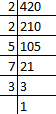420 = 2 x 2 x 3 x 5 x 7

∴ 420 = 22x 3 x 5 x 7

(ii) 468

Solution: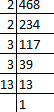468 = 2 x 2 x 3 x 3 x 13

∴ 468 = 22x 33 x 13

(iii) 945

Solution: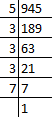945 = 3 x 3 x 3 x 5 x 7

∴ 945 = 33x 5 x 7

(iv) 7325

Solution: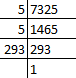7325 = 5 x 5 x 293

∴ 7325 = 55 x 293

2. Determine the prime factorization of each of the following positive integer :

(i) 20570

Solution: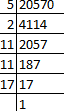20570 = 2 x 5 x 11 x 11 x 17

∴ 20570 = 2 x 5 x 112x 17

(ii) 58500

Solution: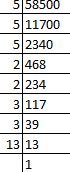58500 = 2 x 2 x 3 x 3 x 5 x 5 x 5 x 13

∴ 58500 = 22 x 32x 53x 13

(iii) 45470971

Solution: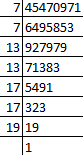45470971 = 7x7x13x13x17x17x19

∴ 45470971 = 72x132x172x19

3. Explain why 7 x 11 x 13 + 13 and 7 x 6 x 5 x 4 x 3 x 2 x 1 + 5 are composite numbers.

Solution:

So, basically there are two types of numbers i.e., prime numbers and composite numbers.

Understanding that,

Prime numbers are those numbers having 1 and the number itself as factors. And,

Composite numbers are those numbers having factors other than 1 and itself.

It’s seen that,

7 x 11 x 13 + 13 = 13 x (7 x 11 + 1) [taking 13 out as common]

= 13 x (77 + 1)

= 13 x 78

= 13 x 13 x 6

So, the given expression has 6 and 13 as its factors. Therefore, we can conclude that it is a composite number.

Similarly,

7 x 6 x 5 x 4 x 3 x 2 x 1 + 5 = 5 x (7 x 6 x 4 x 3 x 2 x 1 + 1) [taking 5 out- common]

= 5 x (1008 + 1)

= 5 x 1009

Since, 1009 is a prime number the given expression has 5 and 1009 as its factors other than 1 and the number itself. Hence, it is also a composite number.

4. Check whether 6n can end with the digit 0 for any natural number n.

Solution:

In order to check whether 6n can end with the digit 0 for any natural number n, let us find the factors of 6.

It’s seen that the factors of 6 are 2 and 3.

So, 6n = (2 x 3)n

6n =2x 3n

Since, the prime factorization of 6 does not contain 5 and 2 as its factor, together. We can thus conclude that 6n can never end with the digit 0 for any natural number n.

5. Explain why 3 × 5 × 7 + 7 is a composite number.

Solution:

So, basically there are two types of numbers i.e., prime numbers and composite numbers.

Understanding that,

Prime numbers are those numbers having 1 and the number itself as factors. And,

Composite numbers are those numbers having factors other than 1 and itself.

It’s seen that,

3 × 5 × 7+ 7 = 7 × (3 × 5 + 1) = 7 × (15 + 1) = 7 × 16

Since, the given expression has 7 and 16 as its factors we can conclude that it is a composite number.

Exercise 1.4 Page No: 1.39

1. Find the LCM and HCF of the following pairs of integers and verify that LCM × HCF = Product of the integers:

(i) 26 and 91

Solution:

Given integers are: 26 and 91

First, find the prime factors of 26 and 91.

26 = 2 × 13

91 = 7 × 13

∴ L.C.M (26, 91) = 2 × 7 × 13 = 182

And,

H.C.F (26, 91) = 13

Verification:

L.C.M × H.C.F = 182 x 13= 2366

And, product of the integers = 26 x 91 = 2366

∴ L.C.M × H.C.F = product of the integers

Hence verified.

(ii) 510 and 92

Solution:

Given integers are: 510 and 92

First, find the prime factors of 510 and 92.

510 = 2 × 3 × 5 × 17

92 = 2 × 2 × 23

∴ L.C.M (510, 92) = 2 × 2 × 3 × 5 × 23 × 17 = 23460

And,

H.C.F (510, 92) = 2

Verification:

L.C.M × H.C.F = 23460 x 2 = 46920

And, product of the integers = 510 x 92 = 46920

∴ L.C.M × H.C.F = product of the integers

Hence verified.

(iii) 336 and 54

Solution:

Given integers are: 336 and 54

First, find the prime factors of 336 and 54.

336 = 2 × 2 × 2 × 2 × 3 × 7

54 = 2 × 3 × 3 x 3

∴ L.C.M (336, 54) = 2 × 2 × 2 × 2 × 3 × 3 × 3 × 7 = 3024

And,

H.C.F (336, 54) = 2 x 3 = 6

Verification:

L.C.M × H.C.F = 3024 x 6 = 18144

And, product of the integers = 336 x 54 = 18144

∴ L.C.M × H.C.F = product of the integers

Hence verified.

2. Find the LCM and HCF of the following integers by applying the prime factorization method:

(i) 12, 15 and 21

Solution:

First, find the prime factors of the given integers: 15, 12 and 21

For, 12 = 2 × 2 × 3

15 = 3 × 5

21 = 3 × 7

Now, L.C.M of 12, 15 and 21 = 2 × 2 × 3 × 5 × 7

∴ L.C.M (12, 15, 21) = 420

And, H.C.F (12, 15 and 21) = 3

(ii) 17, 23 and 29

Solution:

First, find the prime factors of the given integers: 17, 23 and 29

For, 17 = 1 × 17

23 = 1 × 23

29 = 1 × 29

Now, L.C.M of 17, 23 and 29 = 1 × 17 × 23 × 29

∴ L.C.M (17, 23, 29) = 11339

And, H.C.F (17, 23 and 29) = 1

(iii) 8, 9 and 25

Solution:

First, find the prime factors of the given integers: 8, 9 and 25

For, 8 = 2 × 2 x 2

9 = 3 × 3

25 = 5 × 5

Now, L.C.M of 8, 9 and 25 = 23 × 32 × 52

∴ L.C.M (8, 9, 25) = 1800

And, H.C.F (8, 9 and 25) = 1

(iv) 40, 36 and 126

Solution:

First, find the prime factors of the given integers: 40, 36 and 126

For, 40 = 2 x 2 x 2 × 5

36 = 2 x 2 x 3 x 3

126 = 2 × 3 × 3 × 7

Now, L.C.M of 40, 36 and 126 = 23 × 32 × 5 × 7

∴ L.C.M (40, 36, 126) = 2520

And, H.C.F (40, 36 and 126) = 2

(v) 84, 90 and 120

Solution:

First, find the prime factors of the given integers: 84, 90 and 120

For, 84 = 2 × 2 × 3 × 7

90 = 2 × 3 × 3 × 5

120 = 2 × 2 × 2 × 3 × 5

Now, L.C.M of 84, 90 and 120 = 23 × 32 × 5 × 7

∴ L.C.M (84, 90, 120) = 2520

And, H.C.F (84, 90 and 120) = 6

(vi) 24, 15 and 36

Solution:

First, find the prime factors of the given integers: 24, 15 and 36

For, 24 = 2 × 2 x 2 x 3

15 = 3 × 5

36 = 2 × 2 × 3 × 3

Now, LCM of 24, 15 and 36 = 2 × 2 × 2 × 3 × 3 × 5 = 23 x 32 x 5

∴ LCM (24, 15, 36) = 360

And, HCF (24, 15 and 36) = 3

3. Given that HCF (306, 657) = 9 , find LCM ( 306, 657 )

Solution:

Given two integers are: 306 and 657

We know that,

LCM × HCF = Product of the two integers

⇒ LCM = Product of the two integers / HCF

= (306 x 657) / 9 = 22338

4. Can two numbers have 16 as their HCF and 380 as their LCM? Give reason.

Solution:

On dividing 380 by 16 we get,

23 as the quotient and 12 as the remainder.

Now, since the LCM is not exactly divisible by the HCF its can be said that two numbers cannot have 16 as their HCF and 380 as their LCM.

5. The HCF of two numbers is 145 and their LCM is 2175. If one number is 725, find the other.

Solution:

The LCM and HCF of two numbers are 145 and 2175 respectively. (Given)

It is also given that, one of the numbers is 725

We know that,

LCM × HCF = first number × second number

2175 × 145  = 725 × second number

⇒ Second number = (2175 × 145)/ 725 = 435

∴ the other number is 435.

6. The HCF of two numbers is 16 and their product is 3072. Find their LCM.

Solution:

Given,

HCF of two numbers = 16

And, their product = 3072

We know that,

LCM × HCF = Product of the two numbers

LCM × 16 = 3072

⇒ LCM = 3072/ 16 = 192

∴ the LCM of the two numbers is 435.

7. The LCM and HCF of two numbers are 180 and 6 respectively. If one of the numbers is 30, find the other number.

Solution:

Given,

The LCM and HCF of two numbers are 180 and 6 respectively. (Given)

It is also given that, one of the numbers is 30.

We know that,

LCM × HCF = first number × second number

180 × 6 = 30 × second number

⇒ Second number = (180 × 6)/ 30 = 36

∴ the other number is 36.

8. Find the smallest number which when increased by 17 is exactly divisible by both 520 and 468.

Solution:

First let’s find the smallest number which is exactly divisible by both 520 and 468.

That is simply just the LCM of the two numbers.

By prime factorisation, we get

520 = 23 × 5 × 13

468 = 22 × 32 × 13

∴ LCM (520, 468) = 23 × 32 × 5 × 13 = 4680

Hence, 4680 is the smallest number which is exactly divisible by both 520 and 468 i.e. we will get a remainder of 0 in each case. But, we need to find the smallest number which when increased by 17 is exactly divided by 520 and 468.

So that is found by,

4680 – 17 = 4663

∴ 4663 should be the smallest number which when increased by 17 is exactly divisible by both 520 and 468.

9. Find the smallest number which leaves remainders 8 and 12 when divided by 28 and 32 respectively.

Solution:

First, let’s find the smallest number which is exactly divisible by both 28 and 32.

Which is simply just the LCM of the two numbers.

By prime factorisation, we get

28 = 2 × 2 × 7

32 = 25

∴ L.C.M (28, 32) = 25 × 7 = 224

Hence, 224 is the smallest number which is exactly divisible by 28 and 32 i.e. we will get a remainder of 0 in each case. But, we need the smallest number which leaves remainders 8 and 12 when divided by 28 and 32 respectively.

So that is found by,

224 – 8 – 12 = 204

∴ 2041 should be the smallest number which leaves remainders 8 and 12 when divided by 28 and 32 respectively.

10. What is the smallest number that, when divided by 35, 56 and 91 leaves remainders of 7 in each case?

Solution:

First, let’s find the smallest number which is exactly divisible by all 35, 56 and 91.

Which is simply just the LCM of the three numbers.

By prime factorisation, we get

35 = 5 × 7

56 = 23 × 7

91 = 13 × 7

∴ L.C.M (35, 56 and 91) = 23 × 7 × 5 × 13 = 3640

Hence, 3640 is the smallest number which is exactly divisible 28, 42 and 84 i.e. we will get a remainder of 0 in each case. But, we need the smallest number that when divided by 35, 56 and 91 leaves the remainder of 7 in each case.

So that is found by,

3640 + 7 = 3647

∴ 3647 should be the smallest number that when divided by 35, 56 and 91 leaves the remainder of 7 in each case.

11. A rectangular courtyard is 18m 72cm long and 13m 20 cm broad. It is to be paved with square tiles of the same size. Find the least possible number of such tiles.

Solution:

Given,

Length of courtyard = 18 m 72 cm = 1800 cm + 72 cm = 1872 cm (∵1 m = 100 cm)

Breadth of courtyard = 13 in 20 cm = 1300 cm + 20 cm = 1320 cm

The size of the square tile needed to be paved on the rectangular yard is equal to the HCF of the length and breadth of the rectangular courtyard.

Now, finding the prime factors of 1872 and 1320, we have

1872 = 24 × 32 × 13

1320 = 23 × 3 × 5 × 11

⇒ HCF (1872 and 1320) = 23 × 3 = 24

∴ the length of side of the square tile should be 24 cm.

Thus, the number of tiles required = (area of the courtyard) / (area of a square tile)

We know that, area of the courtyard = Length × Breadth

= 1872 cm × 1320 cm

And, area of a square tile = (side)2 = (24cm)2

⇒ the number of tiles required = (1872 x 1320) / (24)2 = 4290

Thus, the least possible number of tiles required is 4290.

12. Find the greatest number of 6 digits exactly divisible by 24, 15 and 36.

Solution:

We know that, the greatest 6 digit number is 999999.

Let’s assume that 999999 is divisible by 24, 15 and 36 exactly.

Then, the LCM (24, 15 and 36) should also divide 999999 exactly.

Finding the prime factors of 24, 15, and 36, we get

24 = 2 × 2 × 2 × 3

15 = 3 × 5

36 = 2 × 2 × 3 × 3

⇒ L.C.M of 24, 15 and 36 = 360

Since, (999999)/ 360 = 2777 × 360 + 279

Here, the remainder is 279.

So, the greatest number which is divisible by all three should be = 999999 – 279 = 9997201

∴ 9997201 is the greatest 6 digit number which is exactly divisible by 24, 15 and 36.

13. Determine the number nearest to 110000 but greater 100000 which is exactly divisible by each of 8, 15 and 21.

Solution:

First, let’s find the L.C.M of 8, 15 and 21.

By prime factorisation, we have

8 = 2 × 2 × 2

15 = 3 × 5

21 = 3 × 7

⇒ L.C.M (8, 15 and 21) = 23 × 3 × 5 × 7 = 840

When 110000 is divided by 840, the remainder that is obtained is 800.

So, 110000 – 800 = 109200 should be divisible by each of 8, 15 and 21.

Also, we have 110000 + 40 = 110040 is also divisible by each of 8, 15 and 21.

⇒ 109200 and 110040 both are greater than 100000 but 110040 is greater than 110000.

Hence, 109200 is the number nearest to 110000 and greater than 100000 which is exactly divisible by each of 8, 15 and 21.

14. Find the least number that is divisible by all the numbers between 1 and 10 (both inclusive).

Solution:

From the question, it’s understood that

The LCM of 1, 2, 3, 4, 5, 6, 7, 8, 9 and 10 we will be the least number that is divisible by all the numbers between 1 and 10.

Hence, the prime factors of all these numbers are:

1 = 1

2 = 2

3 = 3

4 = 2 × 2

5 = 5

6 = 2 × 3

7 = 7

8 = 2 × 2 × 2

9 = 3 × 3

10 = 2 × 5

⇒ L.C.M will be = 23 × 32 × 5 × 7 = 2520

Hence, 2520 is the least number that is divisible by all the numbers between 1 and 10 (both inclusive)

15.  A circular field has a circumference of 360km. Three cyclists start together and can cycle 48, 60 and 72 km a day, round the field. When will they meet again?

Solution:

In order to calculate the time take before they meet again, we must first find out the individual time taken by each cyclist in covering the total distance.

Number of days a cyclist takes to cover the circular field = (Total distance of the circular field) / (distance covered in 1 day by a cyclist).

So, for the 1st cyclist, number of days = 360 / 48 = 7.5 which is = 180 hours [∵1 day = 24 hours]

2nd cyclist, number of days = 360 / 60 = 6 which is = 144 hours

3rd cyclist, number of days = 360 / 72 = 5 which is 120 hours

Now, by finding the LCM (180, 144 and 120) we’ll get to know after how many hours the three cyclists meet again.

By prime factorisation, we get

180 = 22 x 32 x 5

144 = 24 x 32

120 = 23 x 3 x 5

⇒ L.C.M (180, 144 and 120) = 24 x 32 x 5 = 720

So, this means that after 720 hours the three cyclists meet again.

⇒ 720 hours = 720 / 24 = 30 days [∵1 day = 24 hours]

Thus, all the three cyclists will meet again after 30 days.

16. In a morning walk three persons step off together, their steps measure 80 cm, 85 cm and 90 cm respectively. What is the minimum distance each should walk so that he can cover the distance in complete steps?

Solution:

From the question it’s understood that the required distance each should walk would be the L.C.M of the measures of their steps i.e. 80 cm, 85 cm, and 90 cm.

So, finding L.C.M (80, 85 and 90) by prime factorization we get,

80 = 24 × 5

85 = 17 × 5

90 = 2 × 3 × 3 × 5

⇒ L.C.M (80, 85 and 90) = 24 × 32 × 5 × 17 = 12240 cm = 122m 40cm [∵1 m = 100cm]

Hence, 122 m 40 cm is the minimum distance that each should walk so that all can cover the same distance in complete steps.

Exercise 1.5 Page No: 1.49

1. Show that the following numbers are irrational.

(i) 1/√2

Solution:

Let’s assume on the contrary that 1/√2 is a rational number. Then, there exist positive integers a and b such that

1/√2 = a/b where, a and b, are co-primes

⇒ (1/√2)2 = (a/b)2

⇒ 1/2 = a2/b2

⇒ 2a2 = b2

⇒ 2 | b2 [∵ 2|2b2 and 2a2 = b2]

⇒ 2 | b ………… (ii)

⇒ b = 2c for some integer c.

⇒ b2 = 4c2

⇒ 2a2 = 4c2 [∵ b2 = 2a2]

⇒ a2 = 2c2

⇒ 2 | a2

⇒ 2 | a ……… (i)

From (i) and (ii), we can infer that 2 is a common factor of a and b. But, this contradicts the fact that a and b are co-primes. So, our assumption is incorrect.

Hence, 1/√2 is an irrational number.

(ii) 7√5

Solution:

Let’s assume on the contrary that 7√5 is a rational number. Then, there exist positive integers a and b such that

7√5 = a/b where, a and b, are co-primes

⇒ √5 = a/7b

⇒ √5 is rational [∵ 7, a and b are integers ∴ a/7b is a rational number]

This contradicts the fact that √5 is irrational. So, our assumption is incorrect.

Hence, 1/√2 is an irrational number.

(iii) 6 + √2

Solution:

Let’s assume on the contrary that 6+√2 is a rational number. Then, there exist co prime positive integers a and b such that

6 + √2 = a/b

⇒ √2 = a/b – 6

⇒ √2 = (a – 6b)/b

⇒ √2 is rational [∵ a and b are integers ∴ (a-6b)/b is a rational number]

This contradicts the fact that √2 is irrational. So, our assumption is incorrect.

Hence, 6 + √2 is an irrational number.

(iv) 3 − √5

Solution:

Let’s assume on the contrary that 3-√5 is a rational number. Then, there exist co prime positive integers a and b such that

3-√5 = a/b

⇒ √5 = a/b + 3

⇒ √5 = (a + 3b)/b

⇒ √5 is rational [∵ a and b are integers ∴ (a+3b)/b is a rational number]

This contradicts the fact that √2 is irrational. So, our assumption is incorrect.

Hence, 3-√5 is an irrational number.

2. Prove that the following numbers are irrationals.

(i) 2/√7

Solution:

Let’s assume on the contrary that 2/√7 is a rational number. Then, there exist co-prime positive integers a and b such that

2/√7 = a/b

⇒ √7 = 2b/a

⇒ √7 is rational [∵ 2, a and b are integers ∴ 2b/a is a rational number]

This contradicts the fact that √7 is irrational. So, our assumption is incorrect.

Hence, 2/√7 is an irrational number.

(ii) 3/(2√5)

Solution:

Let’s assume on the contrary that 3/(2√5) is a rational number. Then, there exist co – prime positive integers a and b such that

3/(2√5) = a/b

⇒ √5 = 3b/2a

⇒ √5 is rational [∵ 3, 2, a and b are integers ∴ 3b/2a is a rational number]

This contradicts the fact that √5 is irrational. So, our assumption is incorrect.

Hence, 3/(2√5) is an irrational number.

(iii) 4 + √2

Solution:

Let’s assume on the contrary that 4 + √2 is a rational number. Then, there exist co prime positive integers a and b such that

4 + √2 = a/b

⇒ √2 = a/b + 4

⇒ √2 = (a + 4b)/b

⇒ √2 is rational [∵ a and b are integers ∴ (a + 4b)/b is a rational number]

This contradicts the fact that √2 is irrational. So, our assumption is incorrect.

Hence, 4 + √2 is an irrational number.

(iv) 5√2

Solution:

Let’s assume on the contrary that 5√2 is a rational number. Then, there exist positive integers a and b such that

5√2 = a/b where, a and b, are co-primes

⇒ √2 = a/5b

⇒ √2 is rational [∵ a and b are integers ∴ a/5b is a rational number]

This contradicts the fact that √2 is irrational. So, our assumption is incorrect.

Hence, 5/√2 is an irrational number.

3. Show that 2 − √3 is an irrational number.

Solution:

Let’s assume on the contrary that 2 – √3 is a rational number. Then, there exist co prime positive integers a and b such that

2 – √3= a/b

⇒ √3 = 2 – a/b

⇒ √3 = (2b – a)/b

⇒ √3 is rational [∵ a and b are integers ∴ (2b – a)/b is a rational number]

This contradicts the fact that √3 is irrational. So, our assumption is incorrect.

Hence, 2 – √3 is an irrational number.

4. Show that 3 + √2 is an irrational number.

Solution:

Let’s assume on the contrary that 3 + √2 is a rational number. Then, there exist co prime positive integers a and b such that

3 + √2= a/b

⇒ √2 = a/b – 3

⇒ √2 = (a – 3b)/b

⇒ √2 is rational [∵ a and b are integers ∴ (a – 3b)/b is a rational number]

This contradicts the fact that √2 is irrational. So, our assumption is incorrect.

Hence, 3 – √2 is an irrational number.

5. Prove that 4 − 5√2 is an irrational number.

Solution:

Let’s assume on the contrary that 4 – 5√2 is a rational number. Then, there exist co prime positive integers a and b such that

4 – 5√2 = a/b

⇒ 5√2 = 4 – a/b

⇒ √2 = (4b – a)/(5b)

⇒ √2 is rational [∵ 5, a and b are integers ∴ (4b – a)/b is a rational number]

This contradicts the fact that √2 is irrational. So, our assumption is incorrect.

Hence, 4 – 5√2 is an irrational number.

6. Show that 5 − 2√3 is an irrational number.

Solution:

Let’s assume on the contrary that 5 – 2√3 is a rational number. Then, there exist co prime positive integers a and b such that

5 – 2√3 = a/b

⇒ 2√3 = 5 – a/b

⇒ √2 = (5b – a)/(2b)

⇒ √2 is rational [∵ 2, a and b are integers ∴ (5b – a)/b is a rational number]

This contradicts the fact that √2 is irrational. So, our assumption is incorrect.

Hence, 5 – 2√3 is an irrational number.

7. Prove that 2√3 − 1 is an irrational number.

Solution:

Let’s assume on the contrary that 2√3 – 1 is a rational number. Then, there exist co prime positive integers a and b such that

2√3 – 1 = a/b

⇒ 2√3 = a/b – 1

⇒ √3 = (a – b)/(2b)

⇒ √3 is rational [∵ 2, a and b are integers ∴ (a – b)/2b is a rational number]

This contradicts the fact that √3 is irrational. So, our assumption is incorrect.

Hence, 2√3 – 1 is an irrational number.

8. Prove that 2 − 3√5 is an irrational number.

Solution:

Let’s assume on the contrary that 2 – 3√5 is a rational number. Then, there exist co prime positive integers a and b such that

2 – 3√5 = a/b

⇒ 3√5 = 2 – a/b

⇒ √5 = (2b – a)/(3b)

⇒ √5 is rational [∵ 3, a and b are integers ∴ (2b – a)/b is a rational number]

This contradicts the fact that √5 is irrational. So, our assumption is incorrect.

Hence, 2 – 3√5 is an irrational number.

9. Prove that √5 + √3 is irrational.

Solution:

Let’s assume on the contrary that √5 + √3 is a rational number. Then, there exist co prime positive integers a and b such that

√5 + √3 = a/b

⇒ √5 = (a/b) – √3

⇒ (√5)2 = ((a/b) – √3)2 [Squaring on both sides]

⇒ 5 = (a2/b2) + 3 – (2√3a/b)

⇒ (a2/b2) – 2 = (2√3a/b)

⇒ (a/b) – (2b/a) = 2√3

⇒ (a2 – 2b2)/2ab = √3

⇒ √3 is rational [∵ a and b are integers ∴ (a2 – 2b2)/2ab is rational]

This contradicts the fact that √3 is irrational. So, our assumption is incorrect.

Hence, √5 + √3 is an irrational number.

10. Prove that √2 + √3 is irrational.

Solution:

Let’s assume on the contrary that √2 + √3 is a rational number. Then, there exist co prime positive integers a and b such that

√2 + √3 = a/b

⇒ √2 = (a/b) – √3

⇒ (√2)2 = ((a/b) – √3)2 [Squaring on both sides]

⇒ 2 = (a2/b2) + 3 – (2√3a/b)

⇒ (a2/b2) + 1 = (2√3a/b)

⇒ (a/b) + (b/a) = 2√3

⇒ (a2 + b2)/2ab = √3

⇒ √3 is rational [∵ a and b are integers ∴ (a2 + 2b2)/2ab is rational]

This contradicts the fact that √3 is irrational. So, our assumption is incorrect.

Hence, √2 + √3 is an irrational number.

11. Prove that for any prime positive integer p, √p is an irrational number.

Solution:

Let’s assume on the contrary that √p is a rational number. Then, there exist positive integers a and b such that

√p = a/b where, a and b, are co-primes

⇒ (√p)2 = (a/b)2

⇒ p = a2/b2

⇒ pb2 = a2

⇒ p | a2 [∵ p|pb2 and pb2 = a2]

⇒ p | a ………… (ii)

⇒ a = pc for some integer c.

Now, b2p = a2

⇒ b2p = p2c2

⇒ b2 = pc2

⇒ p | b2 [∵ p|pc2 and pc2 = b2]

⇒ p | b ……… (i)

From (i) and (ii), we can infer that p is a common factor of a and b. But, this contradicts the fact that a and b are co-primes. So, our assumption is incorrect.

Hence, √p is an irrational number.

12. If p, q are prime positive integers, prove that √p + √q is an irrational number.

Solution:

Let’s assume on the contrary that √p + √q is a rational number. Then, there exist co prime positive integers a and b such that

√p + √q = a/b

⇒ √p = (a/b) – √q

⇒ (√p)2 = ((a/b) – √q)2 [Squaring on both sides]

⇒ p = (a2/b2) + q – (2√q a/b)

⇒ (a2/b2) + (p-q) = (2√q a/b)

⇒ (a/b) + ((p-q)b/a) = 2√3

⇒ (a2 + b2(p-q))/2ab = √3

⇒ √3 is rational [∵ a and b are integers ∴ (a2 + b2(p-q))/2ab is rational]

This contradicts the fact that √3 is irrational. So, our assumption is incorrect.

Hence, √p + √q is an irrational number.

Exercise 1.6 Page No: 1.56

1. Without actually performing the long division, state whether the following rational numbers will have a terminating decimal expansion or a non-terminating repeating decimal expansion.

(i)23/8

Solution:

We have, 23/8 and here the denominator is 8.

⇒ 8 = 23 x 5

We see that the denominator 8 of 23/8 is of the form 2m x 5n, where m, n are non-negative integers.

Hence, 23/8 has terminating decimal expansion. And, the decimal expansion of 23/8 terminates after three places of decimal.

(ii) 125/441

Solution:

We have, 125/441 and here the denominator is 441.

⇒ 441 = 32 x 72

We see that the denominator 441 of 125/441 is not of the form 2m x 5n, where m, n are non-negative integers.

Hence, 125/441 has non-terminating repeating decimal expansion.

(iii) 35/50

Solution:

We have, 35/50 and here the denominator is 50.

⇒ 50 = 2 x 52

We see that the denominator 50 of 35/50 is of the form 2m x 5n, where m, n are non-negative integers.

Hence, 35/50 has terminating decimal expansion. And, the decimal expansion of 35/50 terminates after two places of decimal.

(iv) 77/210

Solution:

We have, 77/210 and here the denominator is 210.

⇒ 210 = 2 x 3 x 5 x 7

We see that the denominator 210 of 77/210 is not of the form 2m x 5n, where m, n are non-negative integers.

Hence, 77/210 has non-terminating repeating decimal expansion.

(v) 129/(22 x 57 x 717)

Solution:

We have, 129/(22 x 57 x 717) and here the denominator is 22 x 57 x 717.

Clearly,

We see that the denominator is not of the form 2m x 5n, where m, n are non-negative integers.

And hence, 125/441 has non-terminating repeating decimal expansion.

(vi) 987/10500

Solution:

We have, 987/10500

But, 987/10500 = 47/500 (reduced form)

And now the denominator is 500.

⇒ 500 = 22 x 53

We see that the denominator 500 of 47/500 is of the form 2m x 5n, where m, n are non-negative integers.

Hence, 987/10500 has terminating decimal expansion. And, the decimal expansion of 987/10500 terminates after three places of decimal.

2. Write down the decimal expansions of the following rational numbers by writing their denominators in the form of 2m x 5n, where m, and n, are the non- negative integers.

(i) 3/8

Solution:

The given rational number is 3/8

It’s seen that, 8 = 23 is of the form 2m x 5n, where m = 3 and n = 0.

So, the given number has terminating decimal expansion.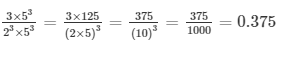(ii) 13/125

Solution:

The given rational number is 13/125.

It’s seen that, 125 = 53 is of the form 2m x 5″, where m = 0 and n = 3.

So, the given number has terminating decimal expansion.

∴ 13/ 125 = (13 x 23)/ (125 x 23) = 104/1000 = 0.104

(iv) 7/80

Solution:

The given rational number is 7/80.

It’s seen that, 80 = 24 x 5 is of the form 2m x 5n, where m = 4 and n = 1.

So, the given number has terminating decimal expansion.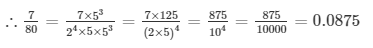(v) 14588/625

Solution:

The given rational number is 14588/625.

It’s seen that, 625 = 54 is of the form 2m x 5n, where m = 0 and n = 4.

So, the given number has terminating decimal expansion.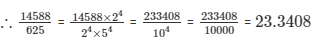(vi) 129/(22 x 57)

Solution:

The given number is 129/(22 x 57).

It’s seen that, 22 x 57 is of the form 2m x 5n, where m = 2 and n = 7.

So, the given number has terminating decimal expansion.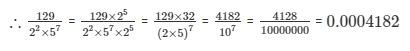3. Write the denominator of the rational number 257/5000 in the form 2m × 5n, where m, n are non-negative integers. Hence, write the decimal expansion, without actual division.

Solution:

The denominator of the given rational number is 5000.

⇒ 5000 = 23 x 54

It’s seen that, 23 x 54 is of the form 2m x 5n, where m = 3 and n = 4.

∴ 257/5000 = (257 x 2)/(5000 x 2) = 514/10000 = 0.0514 is its decimal expansion.

4. What can you say about the prime factorization of the denominators of the following rational:

(i) 43.123456789

Solution:

Since 43.123456789 has terminating decimal expansion. Hence, its denominator is of the form 2m x 5n, where m, n are non-negative integers.

(ii)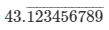Solution:

Since the given rational has non-terminating decimal expansion. So, its denominator has factors other than 2 or 5.

(iii)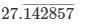Solution:

Since the given rational number has non-terminating decimal expansion. So, its denominator has factors other than 2 or 5.

(iv) 0.120120012000120000….

Solution:

Since 0.120120012000120000…. has non-terminating decimal expansion. So, its denominator has factors other than 2 or 5.

5. A rational number in its decimal expansion is 327.7081. What can you say about the prime factors of q, when this number is expressed in the form p/q? Give reasons.

Solution:

Since, 327.7081 has a terminating decimal expansion its denominator should be of the form 2m x 5n, where m, n are non-negative integers.

Further,

327.7081 can be expressed as 3277081/10000 = p/q

⇒ q = 1000 = 23 x 53

Hence, the prime factors of q has only factors of 2 and 5.

#### 1 Comment

1. Rahul singh munda

Very good